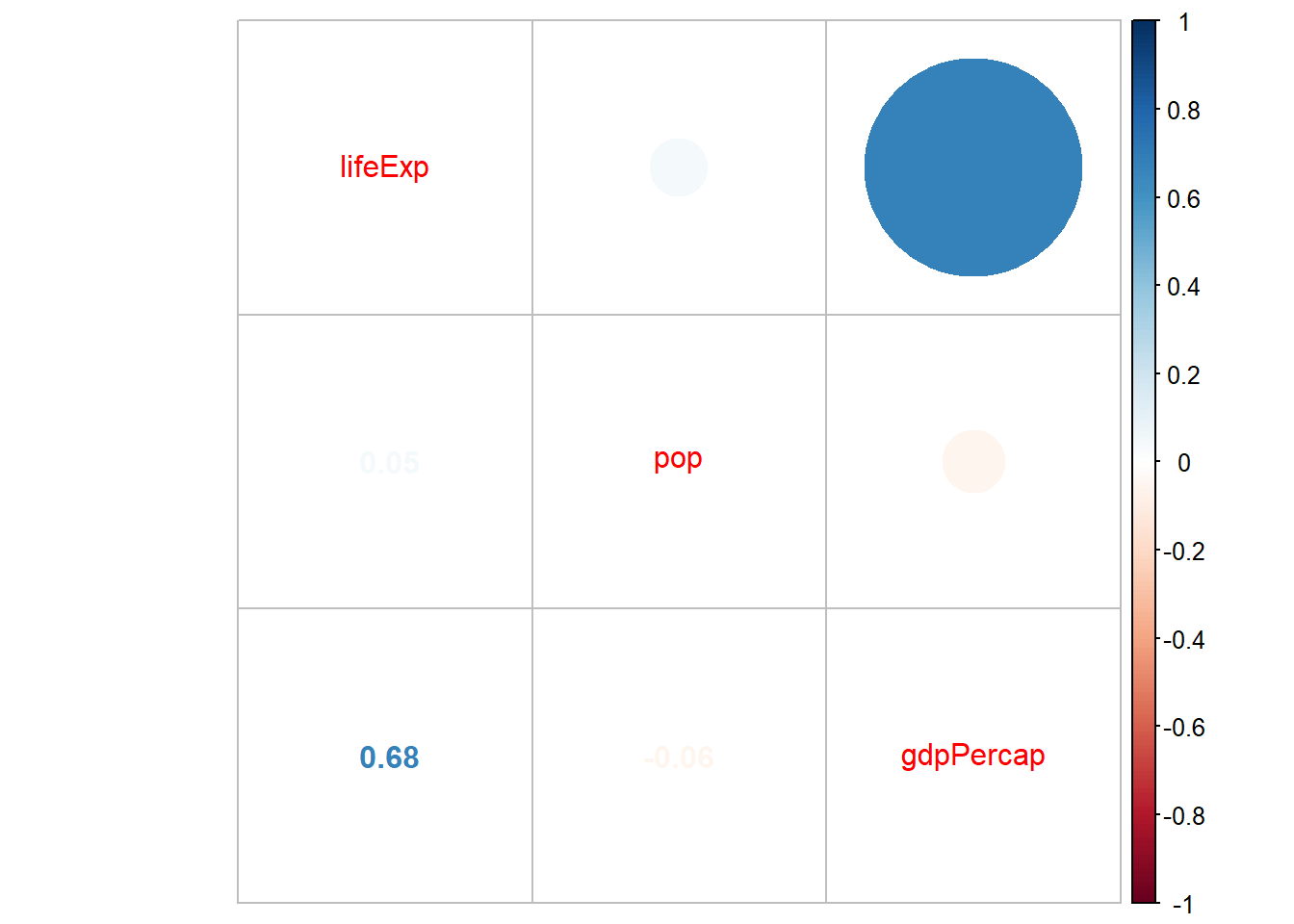# Chapter 4 Data Visualization I

## 4.1 What is ggplot2?

• The ggplot2 package is an R package for data visualization. With ggplot2, you can create nice plots with few lines(?) of codes as follows. Although the codes look complicated for now, you can create plots like this example very soon.
# Code chunk 1: an example plot using ggplot2
# This plot comes from
# https://www.r-graph-gallery.com/320-the-basis-of-bubble-plot.html

library(gapminder)  # to use the gapminder data
library(tidyverse)

# create data
gdp <- gapminder %>%
filter(year=="2007") %>%     # subset rows
dplyr::select(-year) %>%     # subset columns (here remove year with minus sign)
arrange(desc(pop)) %>%       # arrange rows in descending order of pop
mutate(country = factor(country, country))  # create a new variable country

# create a bubble plot
ggplot(data = gdp, mapping = aes(x = gdpPercap, y = lifeExp, size = pop, fill = continent)) +
geom_point(alpha = 0.5, shape = 21, color = "black") +
scale_size(range = c(.1, 24), name = "Population (M)") +
theme_minimal() +
theme(legend.position = "right") +
labs(
subtitle = "Life Expectancy vs. GDP per Capita",
y = "Life Expectancy",
x = "GDP per Capita",
title = "Scatter Plot",
caption = "Source: gapminder"
)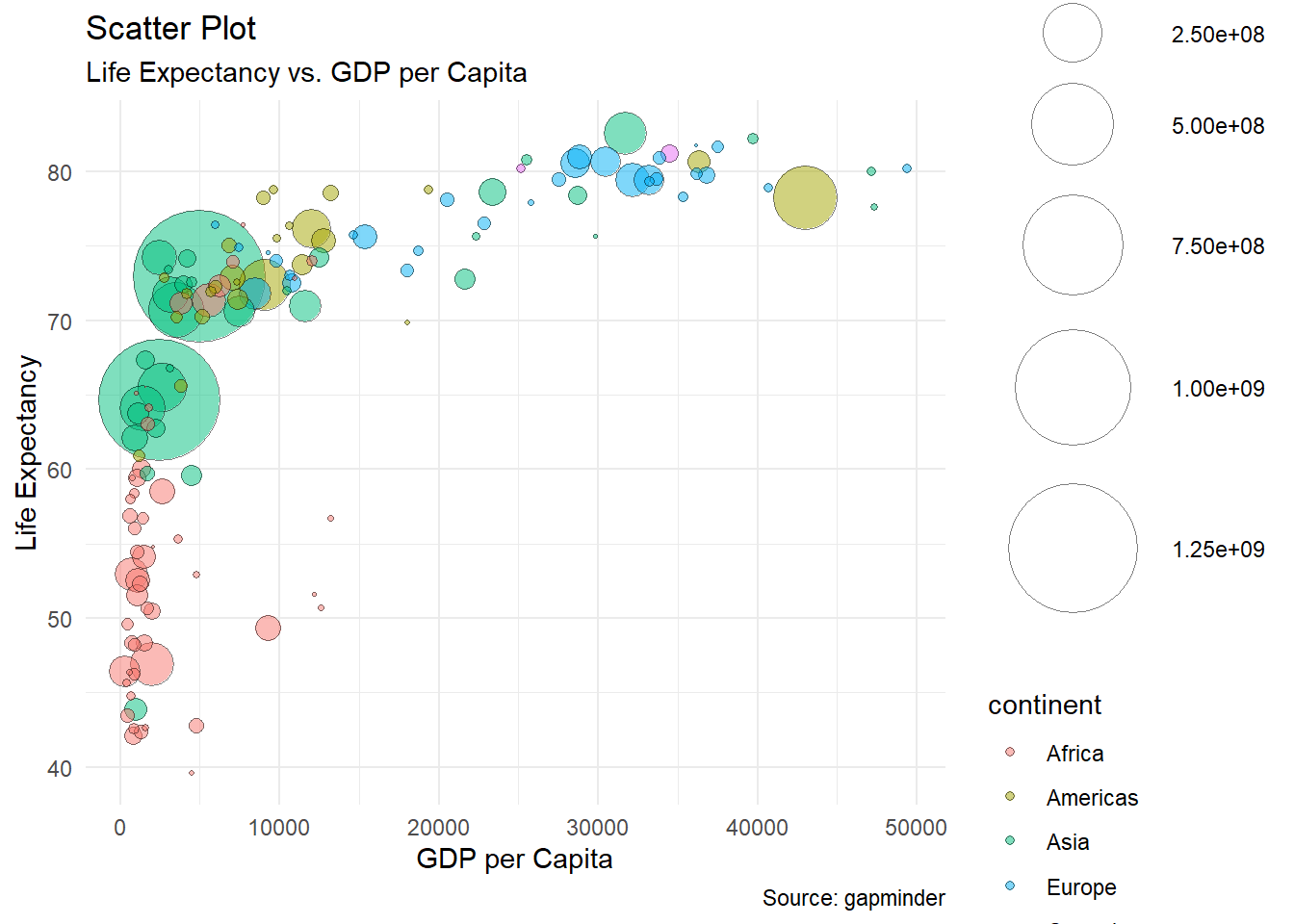• What can you tell from the plot?

• ggplot2 is a part of tidyverse which is a collection of R packages designed for data science in R.

• You can find an official R documentation for ggplot2 (or any R package)

## 4.2 Graphical components

• ggplot2 [@wickham2016ggplot2] was developed to create a graphic by combining few graphical components (e.g., data, coordinate systems, geometric objects, aesthetics, facets, themes) based on the grammar of graphics (gg in ggplot2 stands for grammar of graphics). @wickham2016ggplot2 explains the grammar of graphics as follows:

“Wilkinson (2005) created the grammar of graphics to describe the deep features that underlie all statistical graphics. The grammar of graphics is an answer to a question: what is a statistical graphic? The layered grammar of graphics (Wickham, 2009) builds on Wilkinson’s grammar, focussing on the primacy of layers and adapting it for embedding within R. In brief, the grammar tells us that a statistical graphic is a mapping from data to aesthetic attributes (colour, shape, size) of geometric objects (points, lines, bars). The plot may also contain statistical transformations of the data and is drawn on a specific coordinate system. Faceting can be used to generate the same plot for different subsets of the dataset. It is the combination of these independent components that make up a graphic.”

@wickham2016ggplot2

• The graphical components of the grammar of graphics include
• the data you want to visualize
• the geometric objects (geoms for short; e.g., circles, lines, polygons etc.) that appear on the plot
• a set of aesthetic mappings that describe how variables in the data are mapped to aesthetic properties (e.g., color, shape, linetype) of the geometric objects
• a statistical transformation (stats for short) used to calculate the data values used in the plot
• a position adjustment for arranging each geometric object on the plot
• a scale (e.g., range of values) for each aesthetic mapping used
• a coordinate system (coords for short) used to organize the geometric objects
• the facets or groups of data shown in different plots

## 4.3 ggplot2 syntax

• The basic idea of the ggplot2 package is to build a statistical graph by adding layers representing geometric objects (e.g., points, lines), and the aesthetic properties of the geometric objects (e.g., colors, line types) can be controlled by mapping (or relating) data to the aesthetic properties.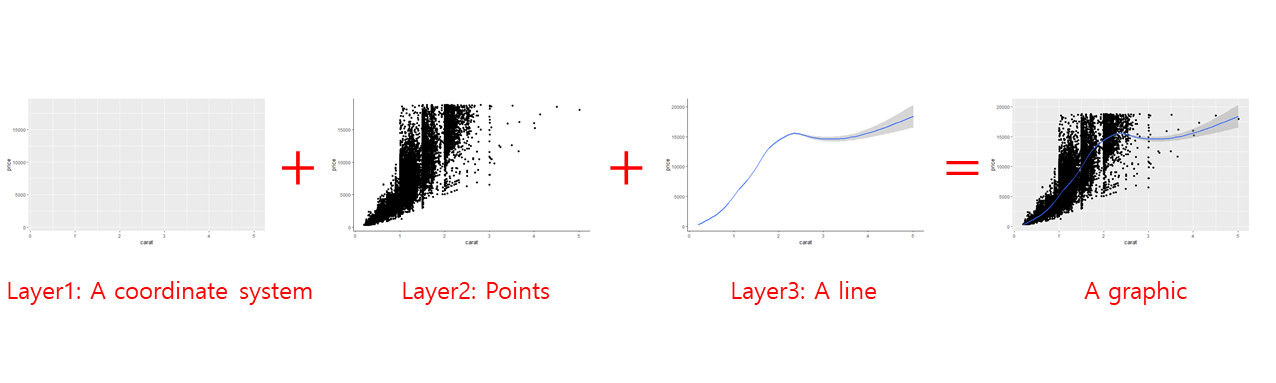A statistical graph can be created by adding layers

• You can create a plot by combining the graphical components of the grammar of graphics based on the following sytax. Essentially, you add layers representing graphical components to a plot with the character +. :
• ggplot(data = <DATA>) +
<GEOM FUNCTION>(mapping = aes(<AESTHETIC MAPPING>), stat = <STAT>, position = <POSITION>) +
<COORDINATE FUNCTION> +
<FACET FUNCTION> +
<SCALE FUNCTION> + <THEME FUNCTION>

## 4.4 Learning objectives of this lecture

• Students can explain the meaning of each graphical component
• Students understand the basics ggplot2 syntax to combine graphical components
• Students can create common plots using ggplot2

## 4.5 Installing and laoding ggplot2

• You can install the ggplot2 package (or any package)
• using install.packages() function
• using the Packages pane
# Code chunk 2: install packages
# from CRAN-like repositories or from local files.
install.packages("ggplot2")
• Once you install the ggplot2 package, you need to load the ggplot2 package onto memory whenever you use the package.
# Code chunk 3: load packages
# library() loads a package into memory
library(ggplot2)
• The ggplot2 package is a part of tidyverse. You can install all the packages in the tidyverse and load the core tidyverse packages by running the following code.
# Code chunk 4
install.packages("tidyverse")
library(tidyverse) # ggplot2 will be also available 

## 4.7 Graphical components of ggplot2

• Data
• Geometric objects (geom for short)
• Aesthetic mappings
• Statistical transformations (stats for short)
• Scales
• A coordinate system (coord for short)
• A faceting

### 4.7.1 Data

• “The data are what you want to visualize.” [@wickham2016ggplot2]

• In order to create plots from data, you first need to import (or read) your own data into R. We will talk about data importing using tidyverse’s readr package later. For now, let’s just assume that you already imported your data into R.

• After importing your data, you may need to get your own data into a useful form for visualization and modeling. This process is often called data wrangling [@wickham2016r]. For example, data wrangling include selecting variables and rows, creating new variables, and merging data sets. Tidyverse provides a set of R packages which help you to wrangle your data. In later lecture of this course, you will learn dplyr, tidyr, purrr, stringr packages in tidyverse for data wrangling. For now, let’s assume that we already have our data in the desired format and just focus in learning ggplot2.

# Code chunk 5
# diamonds is a built-in data in ggplot2
#, meaning that you can use diamonds data after you load ggplot2 package.
# ?diamonds display the help document for data.
# diamonds is a tibble, which is data structure in tidyverse.
# we will talk data structure later.
diamonds
## # A tibble: 53,940 x 10
##    carat cut       color clarity depth table price     x     y     z
##    <dbl> <ord>     <ord> <ord>   <dbl> <dbl> <int> <dbl> <dbl> <dbl>
##  1  0.23 Ideal     E     SI2      61.5    55   326  3.95  3.98  2.43
##  2  0.21 Premium   E     SI1      59.8    61   326  3.89  3.84  2.31
##  3  0.23 Good      E     VS1      56.9    65   327  4.05  4.07  2.31
##  4  0.29 Premium   I     VS2      62.4    58   334  4.2   4.23  2.63
##  5  0.31 Good      J     SI2      63.3    58   335  4.34  4.35  2.75
##  6  0.24 Very Good J     VVS2     62.8    57   336  3.94  3.96  2.48
##  7  0.24 Very Good I     VVS1     62.3    57   336  3.95  3.98  2.47
##  8  0.26 Very Good H     SI1      61.9    55   337  4.07  4.11  2.53
##  9  0.22 Fair      E     VS2      65.1    61   337  3.87  3.78  2.49
## 10  0.23 Very Good H     VS1      59.4    61   338  4     4.05  2.39
## # ... with 53,930 more rows
• The interactive code chunk below was created by the tutorial package. You can directly execute R code in this code chunk.
eyJsYW5ndWFnZSI6InIiLCJzYW1wbGUiOiIjIFBsZWFzZSB0eXBlIGBkaWFtb25kc2AgYmVsb3cgYW5kIHJ1blxuIyBUaGVyZSBhcmUgNTMsOTQwIHJvd3MgYW5kIDEwIGNvbHVtbnMuIENhbiB5b3UgY2hlY2sgdGhlc2UgbnVtYmVyIHdoZW4geW91IHR5cGUgYGRpYW1vbmRzYD9cbiMgXCJjYXJhdCBjdXQgY29sb3IgLi4uIFwiIGFyZSB0aGUgbmFtZXMgb2YgdmFyaWFibGVzLiBcbiMgXCI8ZGJsPiA8b3JkPiA8b3JkPiAuLi4gXCIgYXJlIHRoZSBgZGF0YSB0eXBlYCB3aGljaCB3ZSB3aWxsIHRhbGsgbW9yZSBsYXRlci4gXG4jIDxkYmw+IGluZGljYXRlcyBgZG91YmxlYCAob3IgcmVhbCBudW1iZXIgc3VjaCBhcyAxLjUpXG4jIDxvcmQ+IGluZGljYXRlcyBgb3JkZXJlZCBmYWN0b3JgIChlLmcuLCBzbWFsbCA8IG1lZGl1bSA8IGxhcmdlKVxubGlicmFyeShnZ3Bsb3QyKSAgIyA8LSB5b3UgbmVlZCB0byBsb2FkIHRoZSBwYWNrYWdlIGZpcnN0IHRvIHVzZSBhbnkgZGF0YXNldCBvciBmdW5jdGlvbiBpbiB0aGUgcGFja2FnZSJ9

### 4.7.2 Geometric objects (geoms)

• “Geometric objects, geoms for short, represent what you actually see on the plot” [@wickham2016ggplot2]. For example, geometric objects include points, lines, bars, etc in a plot.

• Geometric objects can be added to a plot as a new layer using a geom function (i.e., geom_*()). For example, data points, which is a geometric object, can be added to a plot as a new layer using the geom_point() function.

• In the cheatsheet, you will find many geom functions and their corresponding geometric objects. For example, we often use the following geom functions:

• geom_point() for scatter plot
• geom_line() for line plot
• geom_histogram() for histogram
• In ggplot2, you can create plots by

1. calling the ggplot() function to begin a plot (or to initialize a ggplot object or to create a blank canvas) that you can add layers to
• An object is a technical terminology. In fact, everything (e.g., vectors, matrices, data frames, lists, functions) in R is an object, a data structure having some values and functions. In computer science, “a data structure is a way to store and organize data in order to facilitate access and modifications” [@cormen2009introduction]. The official definition of an object in R can be found here. We will talk more about data structure in R later.
2. specifying aesthetic mappings to specify how you want to map variables to visual aspects of geometric objects
• Each geom has its own aesthetic properties. For example, the aesthetic properties of points in a plot include points’ color, size, and shape. You can change the color, size, and shape of points in a plot using aesthetic mapping.
3. adding a new layer representing a geometric object to a plot using a geom function
• In short, you create a ggplot object using the ggplot() function, and add additional layer using the geom functions as follows (check the “Basics” section in the cheatsheet):

# Code chunk 6: initialize a ggplot object
# ggplot() initializes a ggplot object.
# In ggplot(), you need to specify 1) a dataset, and 2) aesthetic mapping.
# In the example below, x-position and y-position are mapped into carat and price variables.
# No geometric object yet.
ggplot(data = diamonds, aes(x = carat, y = price))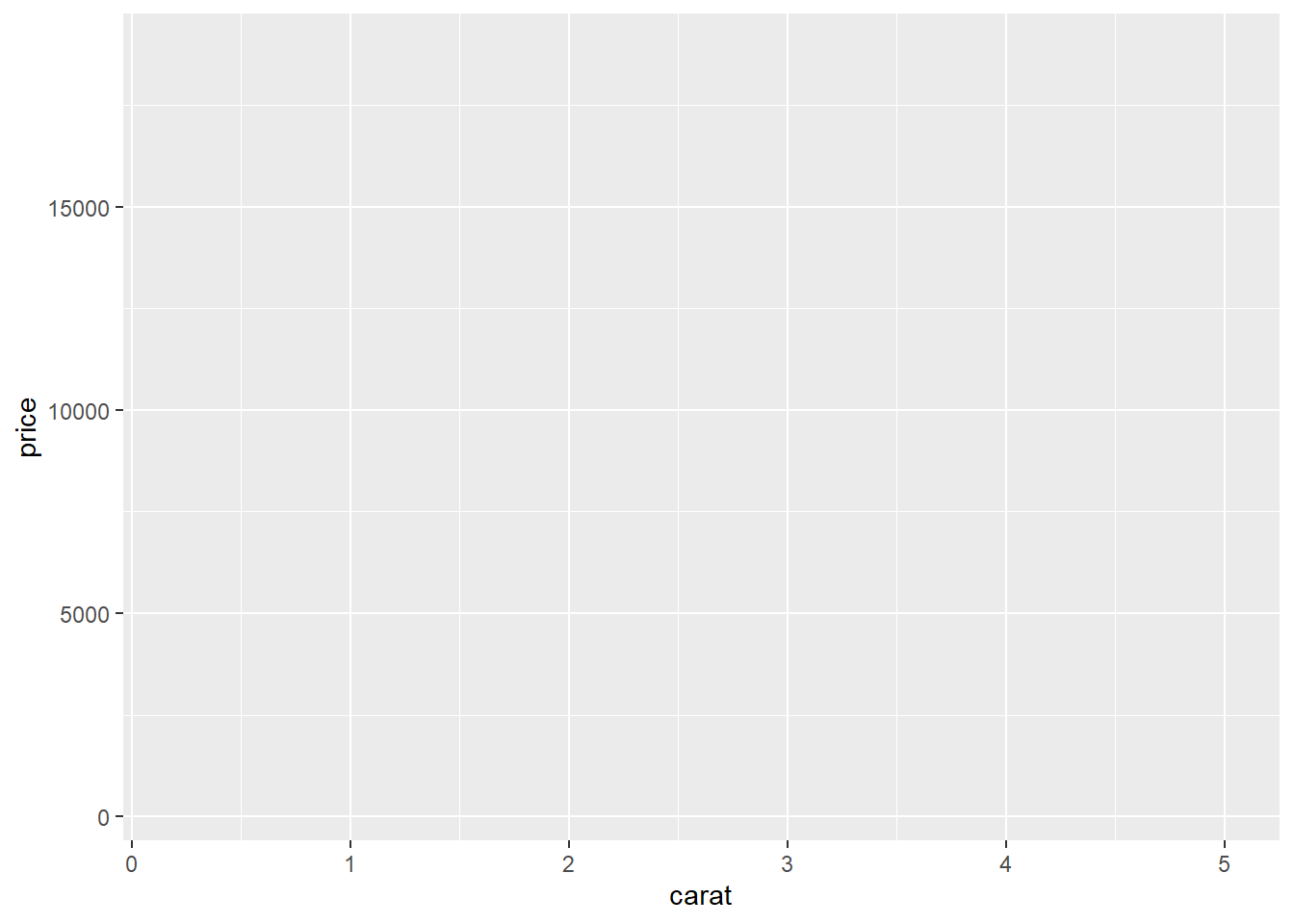# Code chunk 7: add a geometric object
# geom_points() adds a new layer to a plot by drawing points to produce a scatter plot
ggplot(data = diamonds, aes(x = carat, y = price)) + geom_point()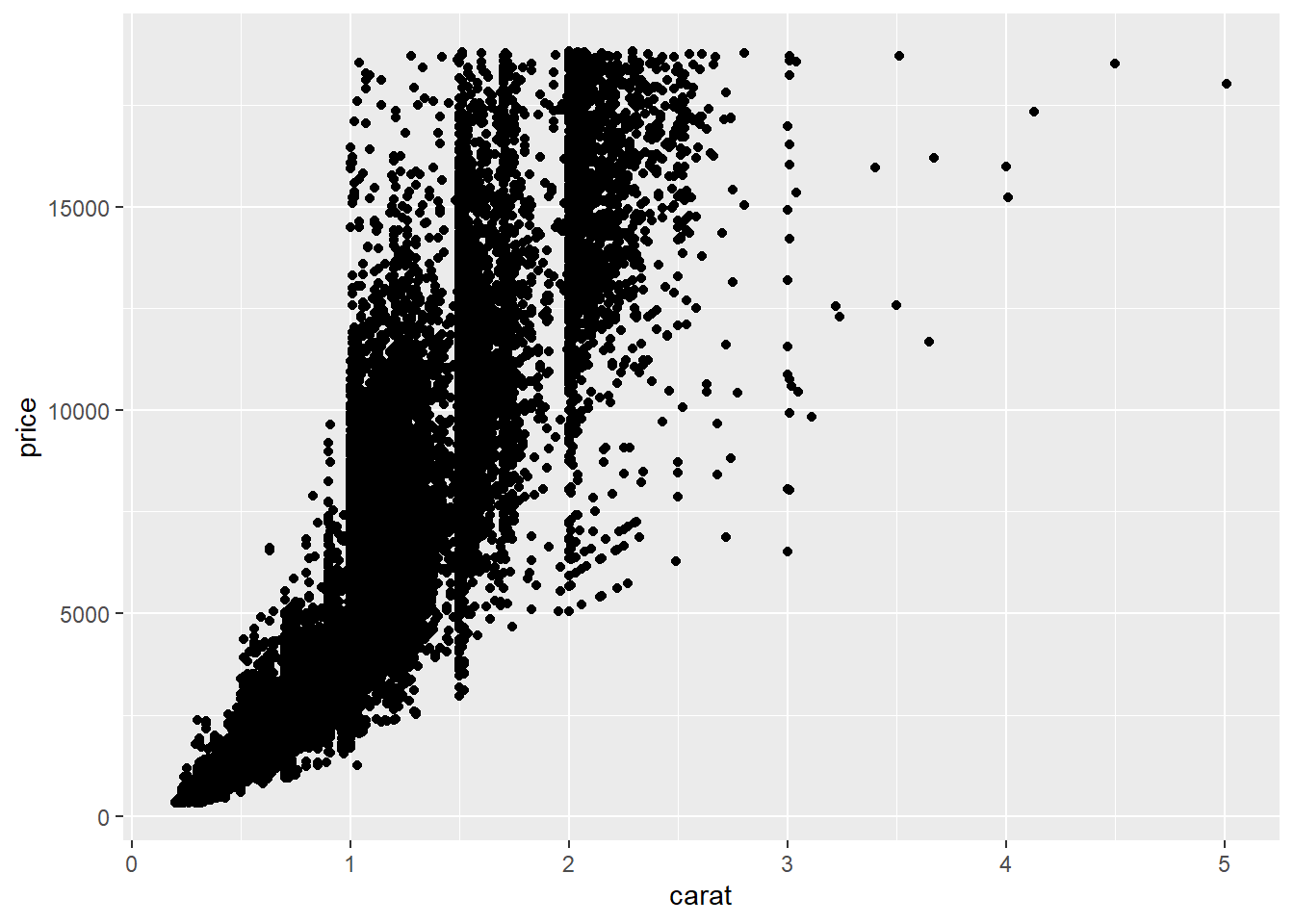# Code chunk 8: add another geometric object
# geom_smooth() adds an additional layer to the plot by drawing a smoothed line to capture the trend in the scatterplot
ggplot(data = diamonds, aes(x = carat, y = price)) + geom_point() + geom_smooth()
## geom_smooth() using method = 'gam' and formula 'y ~ s(x, bs = "cs")'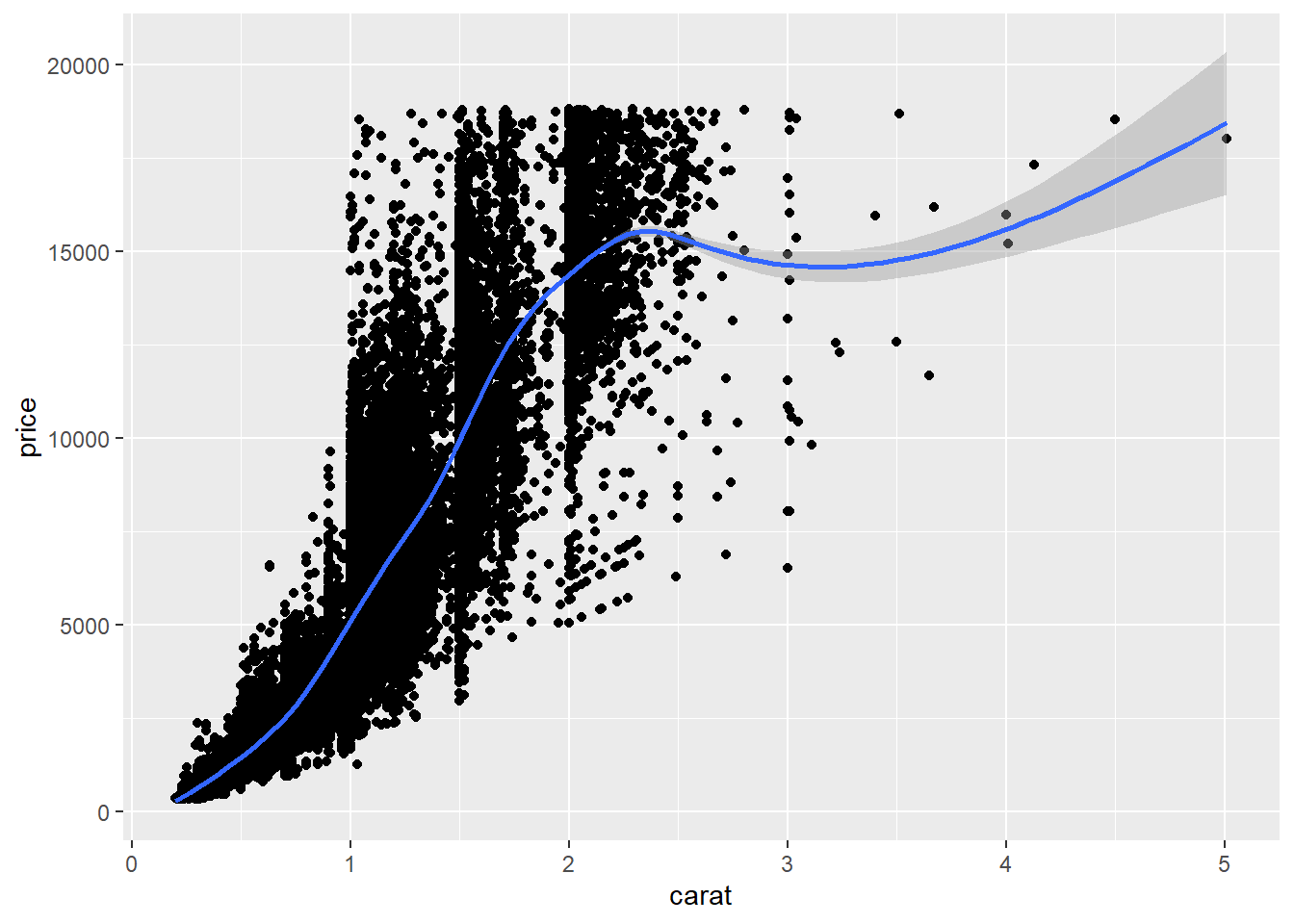Exercise 2-1. Your first ggplot2 plot

• Let’s create your first plot using mtcars data, which is a built-in data in R.

• First, let’s check the variables in the mtcars by just typing mtcars in the interactive R code chunk below.

eyJsYW5ndWFnZSI6InIiLCJzYW1wbGUiOiIjIHR5cGUgYG10Y2Fyc2AgdG8gZGlzcGxheSB0aGUgZGF0YXNldFxuIyBgP210Y2Fyc2Agd2lsbCBkaXNwbGF5IGEgaGVscCBkb2N1bWVudCBmb3IgYG10Y2Fyc2AgZGF0YXNldCJ9
• Second, create a scatter plot between disp(x-axis) and mpg(y-axis) in the mtcars dataset with a smoothed line like the one in the Code chunk 8. If you type ?mtcars, then you can find mtcars is a dataset for motor trend car road test, mpg is a mile/gallon (how many miles can car go with a gallon), and disp is an engine size of a car.
eyJsYW5ndWFnZSI6InIiLCJzYW1wbGUiOiIjIGNoZWNrIHRoZSBDb2RlIGNodW5rIDgifQ==
• What can you tell from the plot about the relationship between fuel efficiency (i.e., mpg) and engine size (i.e., disp)?

### 4.7.3 Aesthetic mappings

• Once you add geometric objects (e.g., points as in the above example) to a plot, you can change the visual (or aesthetic) properties of the geometric objects (e.g., color of the points). You can find some aesthetic properties in R here (e.g., lintypes of a line, shapes of a point, color of a point).

• Often, you may want to change the visual (or aesthetic) properties of the geometric objects by mapping variables in the data to aesthetic properties of geoms using the following syntax: <aesthetic property> = <variable names>. For example, shape = cyl maps the shape aesthetic (e.g., circle, triangle, cross) of points to cyl variable (number of cylinders in the mpg dataset) so that you can encode additional information to a plot using different color of points.

• “A set of aesthetic mappings describe how variables in the data are mapped to aesthetic properties of the layer” [@wickham2016ggplot2]

• “To describe the way that variables in the data are mapped to things that we can perceive on the plot (the "aesthetics"), we use the aes function. The aes function takes a list of aesthetic-variable pairs like these: aes(x = weight, y = height, colour = age). Here we are mapping x-position to weight, y-position to height and colour to age. The first two arguments can be left without names, in which case they correspond to the x and y variables.” [@wickham2016ggplot2]

• Aesthetic properties in ggplot2 include

• position (e.g., x and y coordinates)
• color (outside color)
• fill (inside color)
• shape (of points; e.g., circle, triangle)
• linetype (e.g., solid line, dotted line)
• size
• alpha (transparency)
# Code chunk 9: aesthetic mapping
# color = color maps the variable color in the dataset to the color aesthetics of points to encode further information in the graphic.
ggplot(data = diamonds, aes(x = carat, y = price, color = color)) + geom_point()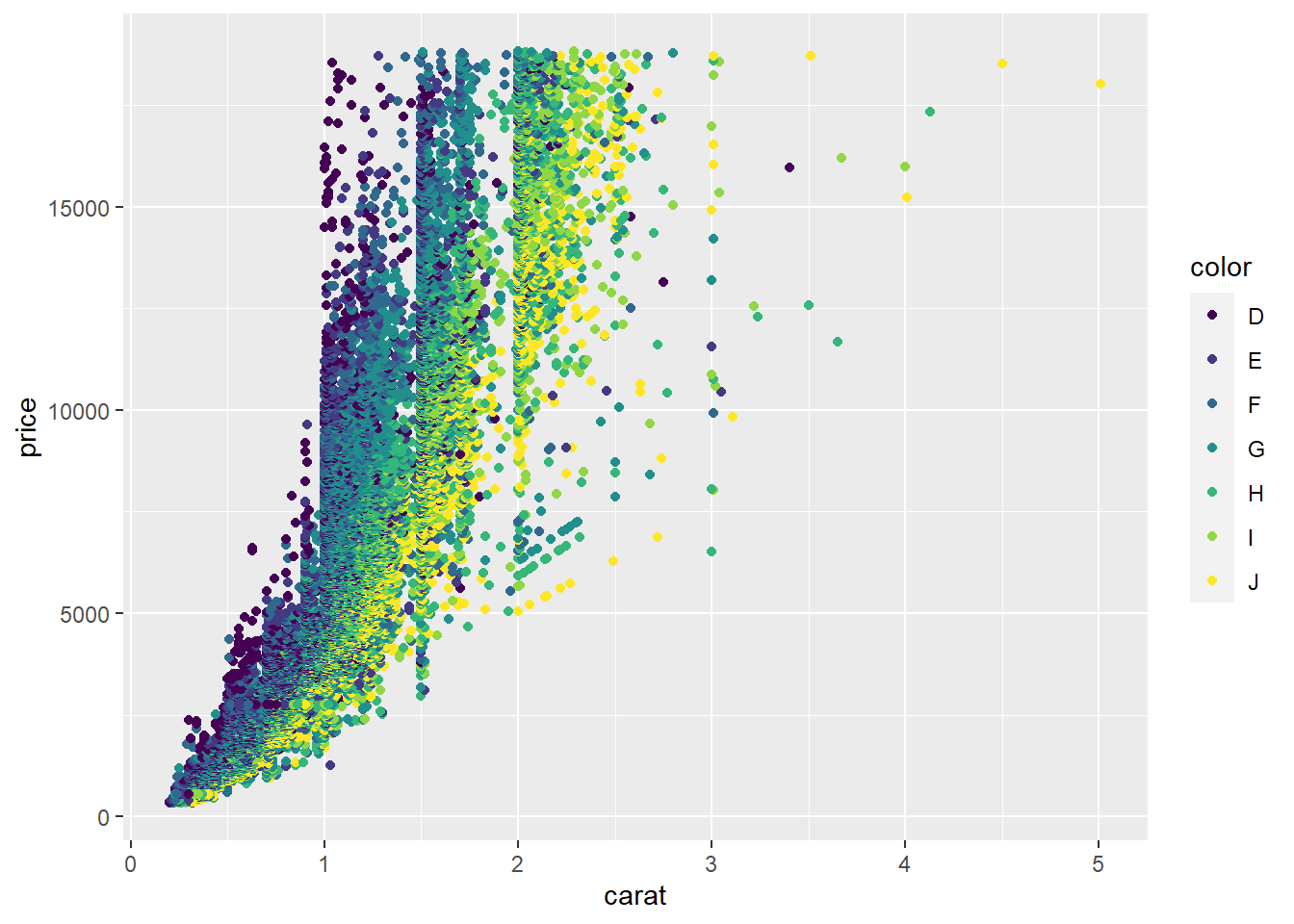# Code chunk 10: aesthetic mapping
# shape = cut maps the shape aesthetics of points to the variable cut in the dataset to encode further information in the graphic.
# Note that the graphic is not so informative because points are overplotted. Sometimes, facetting may handle overplotting.
# We will talk about facetting later in this lecture.
ggplot(data = diamonds, aes(x = carat, y = price, shape = cut)) + geom_point()
## Warning: Using shapes for an ordinal variable is not advised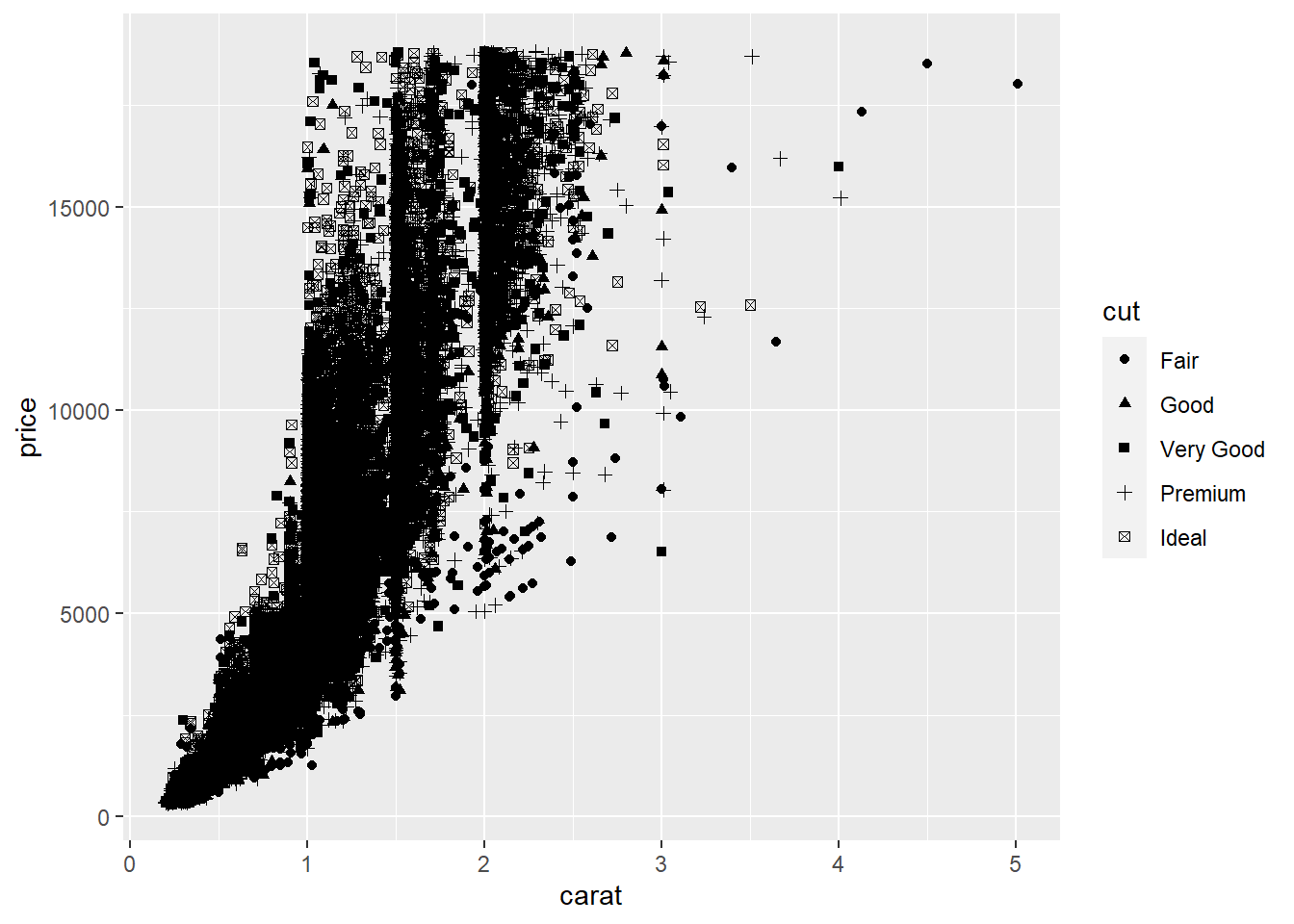# Code chunk 11: set aesthetic properties to a constant
# We can set aesthetic properties to a constant outside aes() function.
ggplot(data = diamonds, aes(x = carat, y = price)) + geom_point(color = "blue")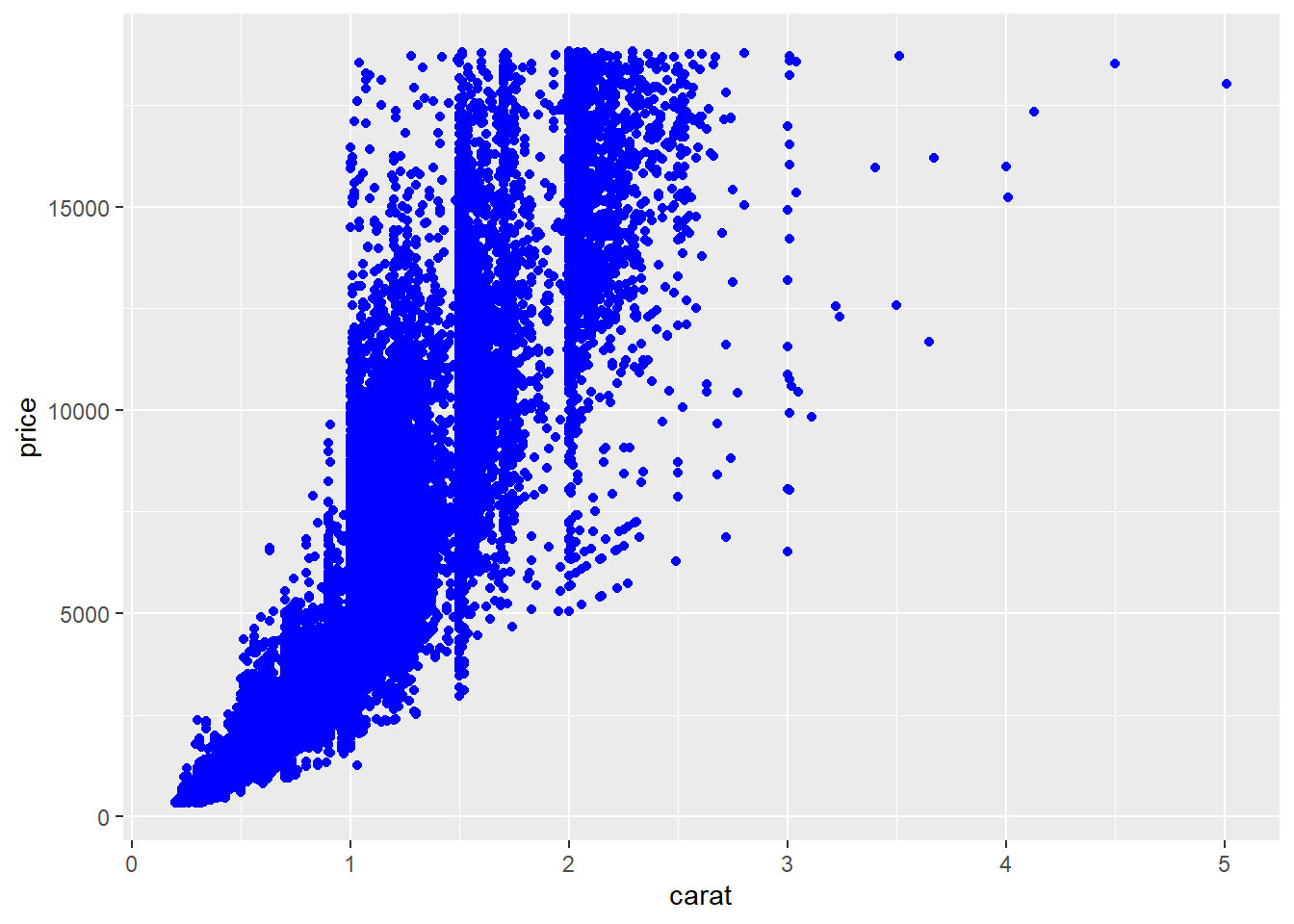Exercise 2-2. Aesthetic mapping

• mpg is similar to mtcars but is a built-in tibble in ggplot2. Plot hwy (mile per gallon: y axis) against displ (engine size: x axis)
• Recall ggplot(data = <dataset name>, aes(<aesthetic mapping>)) + geom_*() ... is a basic format for creating a plot in ggplot2 (check the cheatsheet).
• x and y positions are also aesthetic properties in ggplot2, meaning that you need x = <variable name for x axis> and y = <variable name for y axis>.
eyJsYW5ndWFnZSI6InIiLCJzYW1wbGUiOiIjIGNoZWNrIEV4ZXJjaXNlIDItMSJ9
• Based on the plot from above, map the cyl variable (number of cylinders) to color aesthetics.
eyJsYW5ndWFnZSI6InIiLCJzYW1wbGUiOiIjIGNoZWNrIENvZGUgY2h1bmsgOSBhbmQgMTAifQ==
• Explain what happens? Can you see the additional information are further encoded in the graph?

• This is what happens when mapping x, y, and color aesthetics to hyw, displ, and cyl variables: R creates a new dataset that contains all the data to be displayed on the plot.

x y color
1.8 29 4
1.8 29 4
2.0 31 4
2.0 30 4
2.8 26 6
2.8 26 6
3.1 27 6
1.8 26 4
1.8 25 4
2.0 28 4

### 4.7.4 Scales

• In the previous table, computers don’t know how to display colors based on 4, 6, … Computers need a hexadecimal code for colors such as FF6C91. The mapping from the data to the final values that computers can use to display aesthetics is called a scale. In this sense, a scale controls aesthetic mapping from data to aesthetics.
x y color
1.8 29 #FF6C91
1.8 29 #FF6C91
2.0 31 #FF6C91
2.0 30 #FF6C91
2.8 26 #00C1A9
2.8 26 #00C1A9
3.1 27 #00C1A9
1.8 26 #FF6C91
1.8 25 #FF6C91
2.0 28 #FF6C91
• To control the aesthetic mapping, you can use a scale function (i.e., scale_*()). Please check the Scales section in the cheatsheet. For example, scale_x_continuous() and scale_y_continuous() allow us to change the default scales for continuous x and y aesthetics. If you see the reference guide, you will find scale_x_continuous() and scale_y_continuous() functions allow you to change the name, break points, limits, etc. of the continuous x and y axis.
# Code chunk 12: create a scatter plot
# you assign a name p1 to a ggplot object.
# By assigning a ggplot object to the variable p1, you can easily add different layers to p1.
p1 <- ggplot(mpg, aes(displ, hwy)) + geom_point()
p1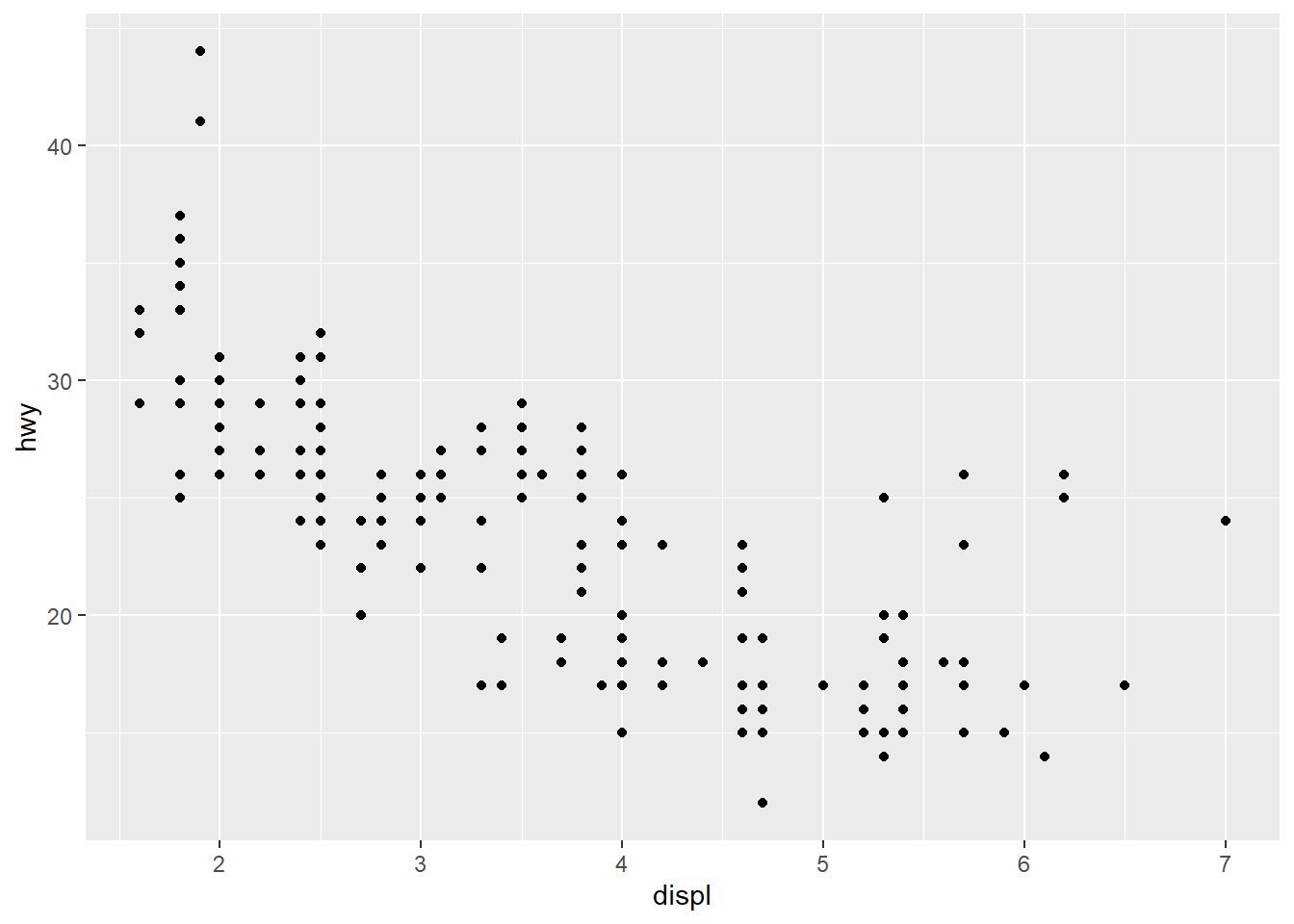# Code chunk 13: change the axis labels
p1 +
scale_x_continuous("Engine displacement (L)") +
scale_y_continuous("Highway MPG")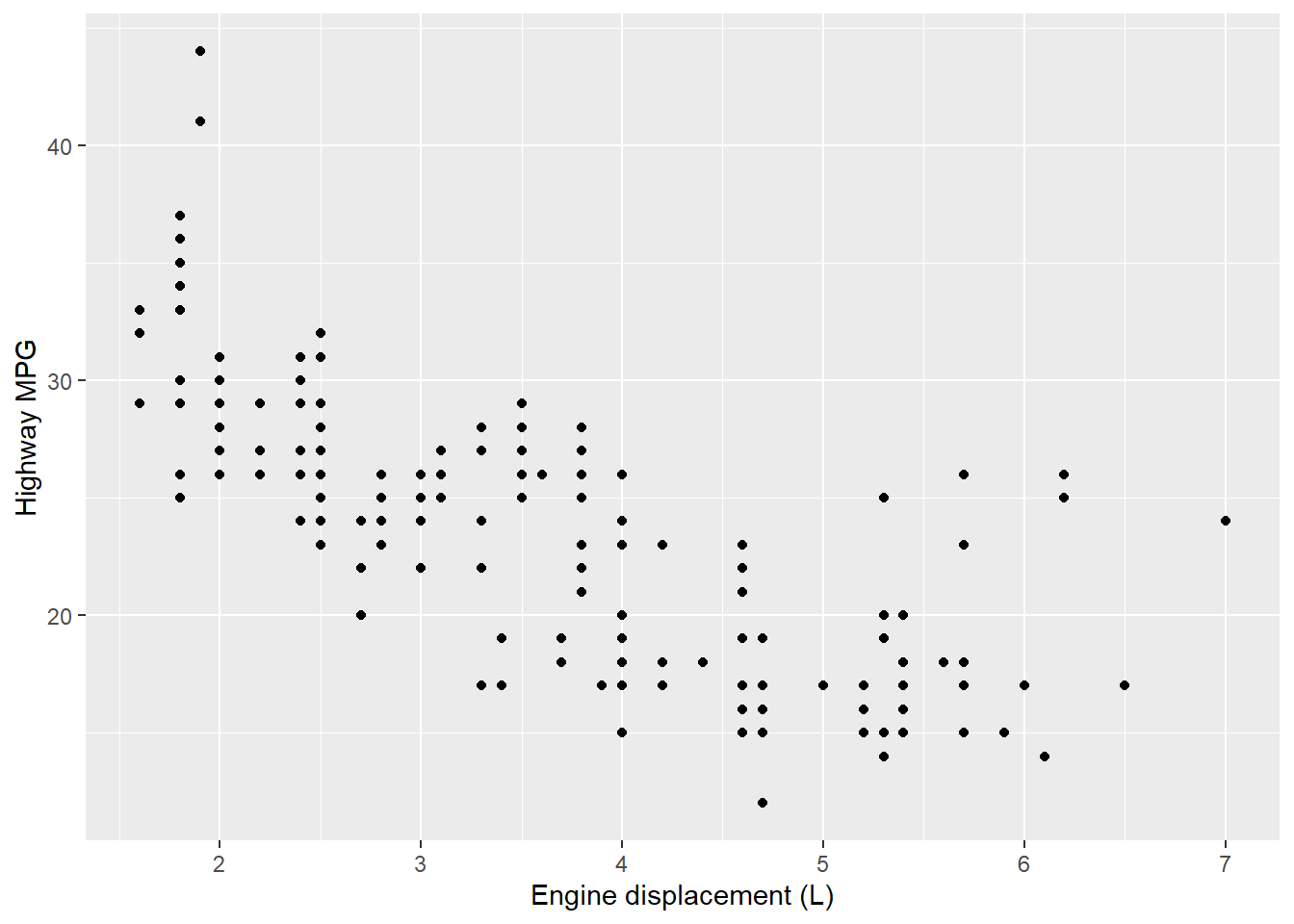# Code chunk 14: change the axis labels
# use the short-cut labs()
p1 + labs(x = "Engine displacement (L)", y = "Highway MPG")# Code chunk 15: modify the axis limits
p1 + scale_x_continuous(limits = c(2, 6))
## Warning: Removed 27 rows containing missing values (geom_point).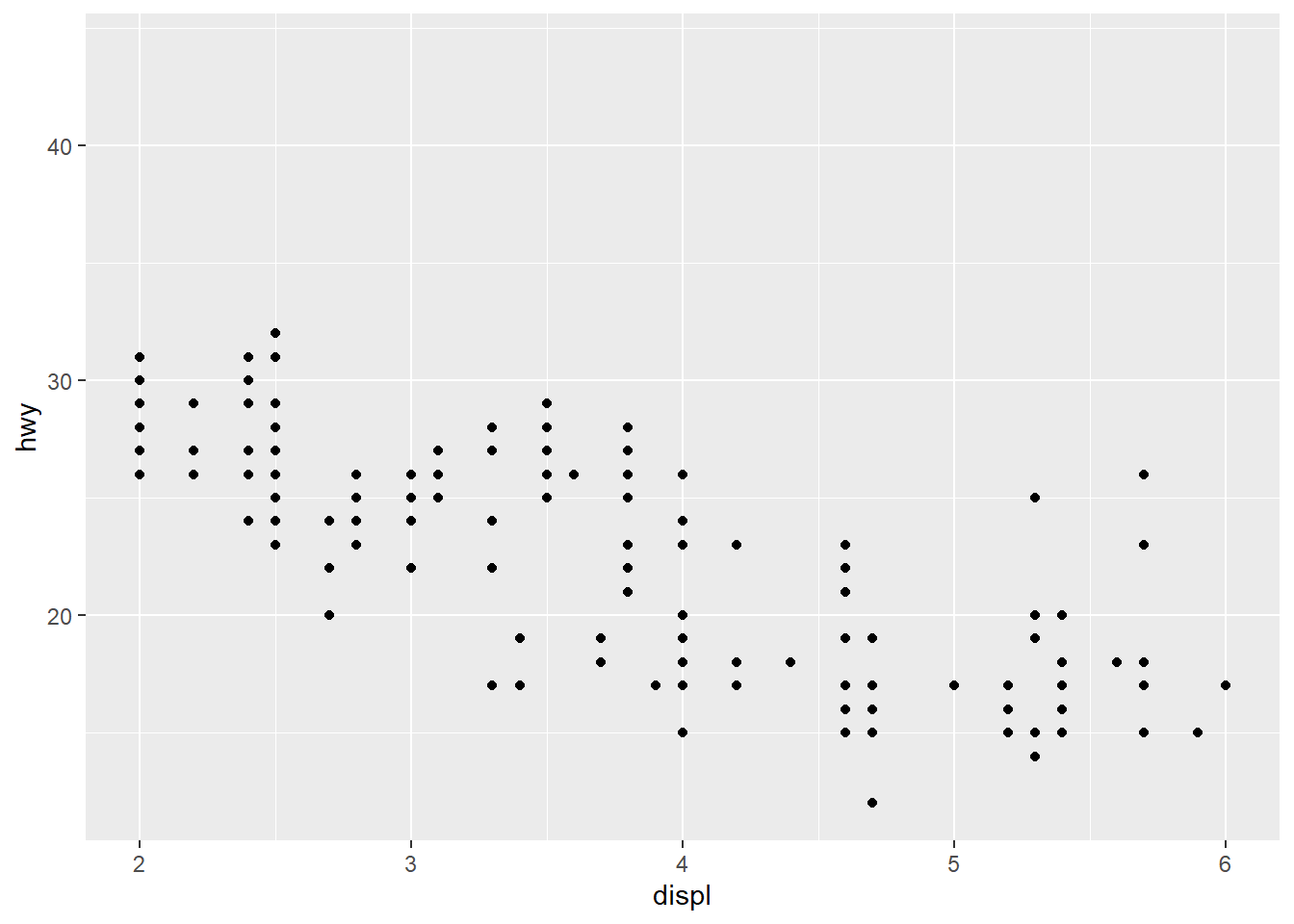# Code chunk 16: modify the axis limits
# use the short hand functions xlim() and ylim()
p1 + xlim(2, 6)
## Warning: Removed 27 rows containing missing values (geom_point).# Code chunk 17: modify the axis limits
#  choose where the ticks appear
p1 + scale_x_continuous(breaks = c(2, 4, 6))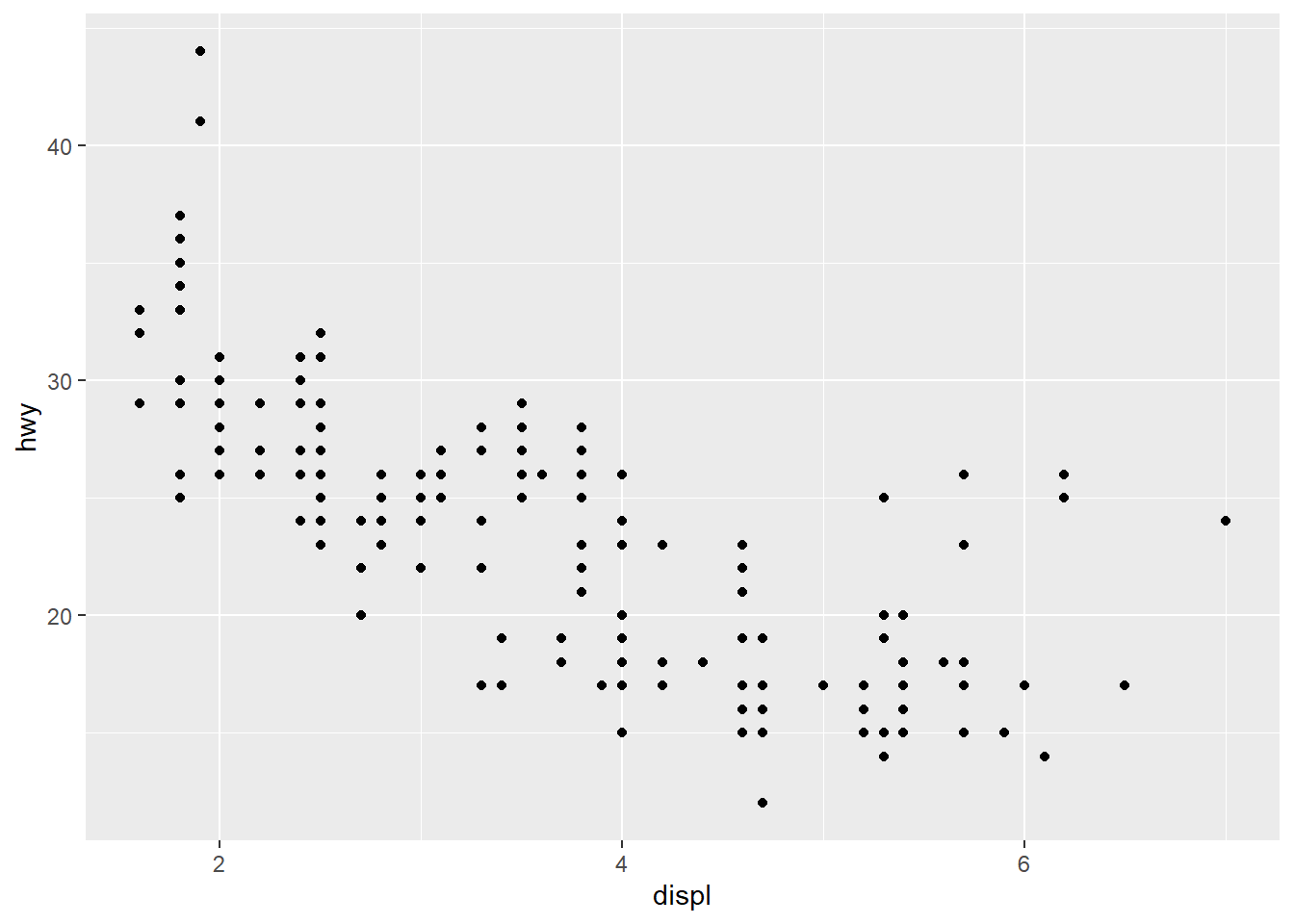# Code chunk 18: choose your own labels
p1 + scale_x_continuous(breaks = c(2, 4, 6), label = c("two", "four", "six"))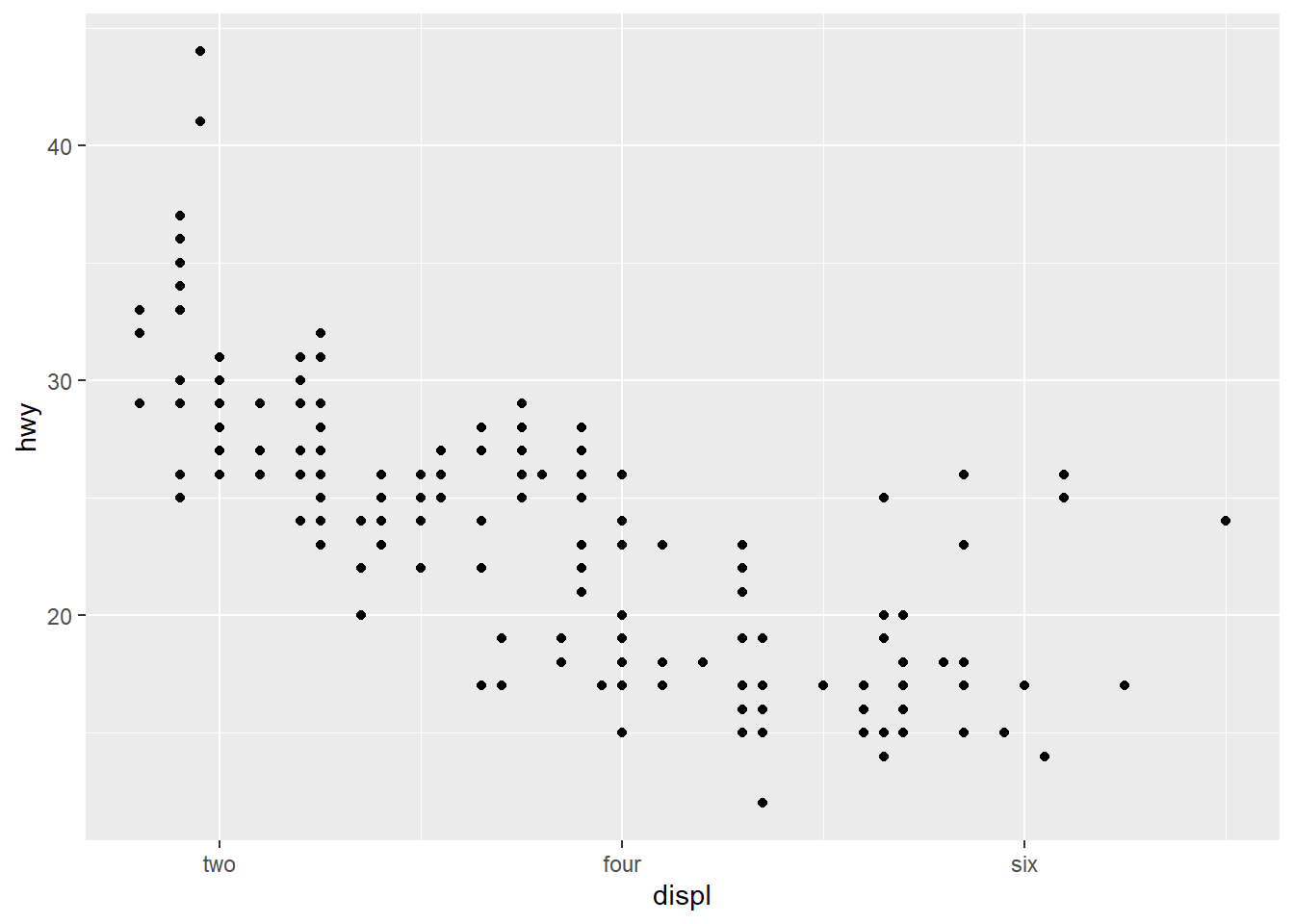# Code chunk 19: add title and axis labels
ggplot(data = mpg, mapping = aes(x = displ, y = hwy, color=drv)) +
geom_point() + geom_smooth(method="lm") +
labs(title ="MPG vs Engine size", x = "Engine size", y = "MPG")
## geom_smooth() using formula 'y ~ x'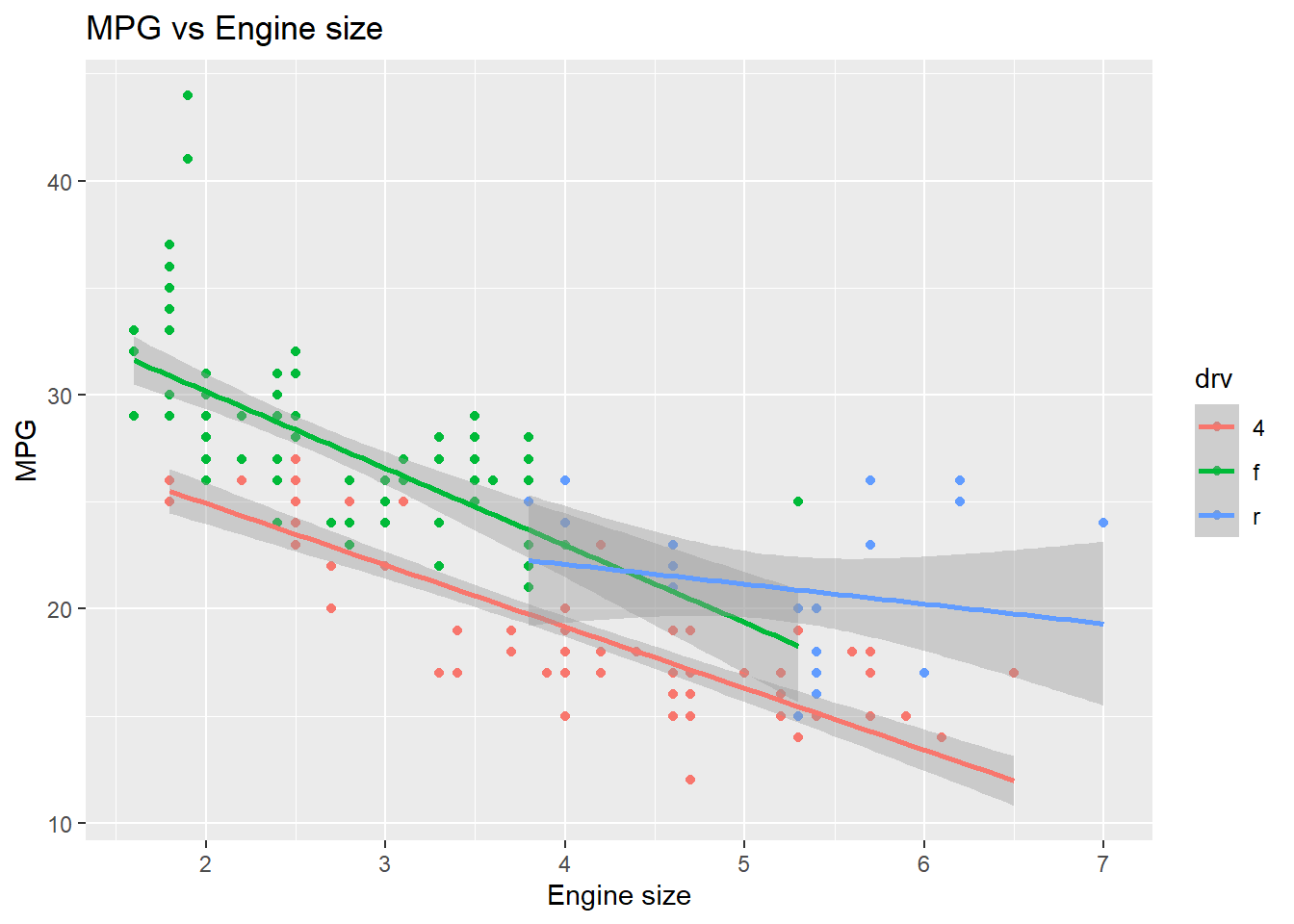# Code chunk 20: Create your own discrete scale
ggplot(data = mpg, mapping = aes(x = displ, y = hwy, color=drv)) +
geom_point() + geom_smooth(method="lm") +
labs(title ="MPG vs Engine size", x = "Engine size", y = "MPG") +
scale_colour_manual(name = "Drive", values = c("lightpink", "darkseagreen", "lightblue"))
## geom_smooth() using formula 'y ~ x'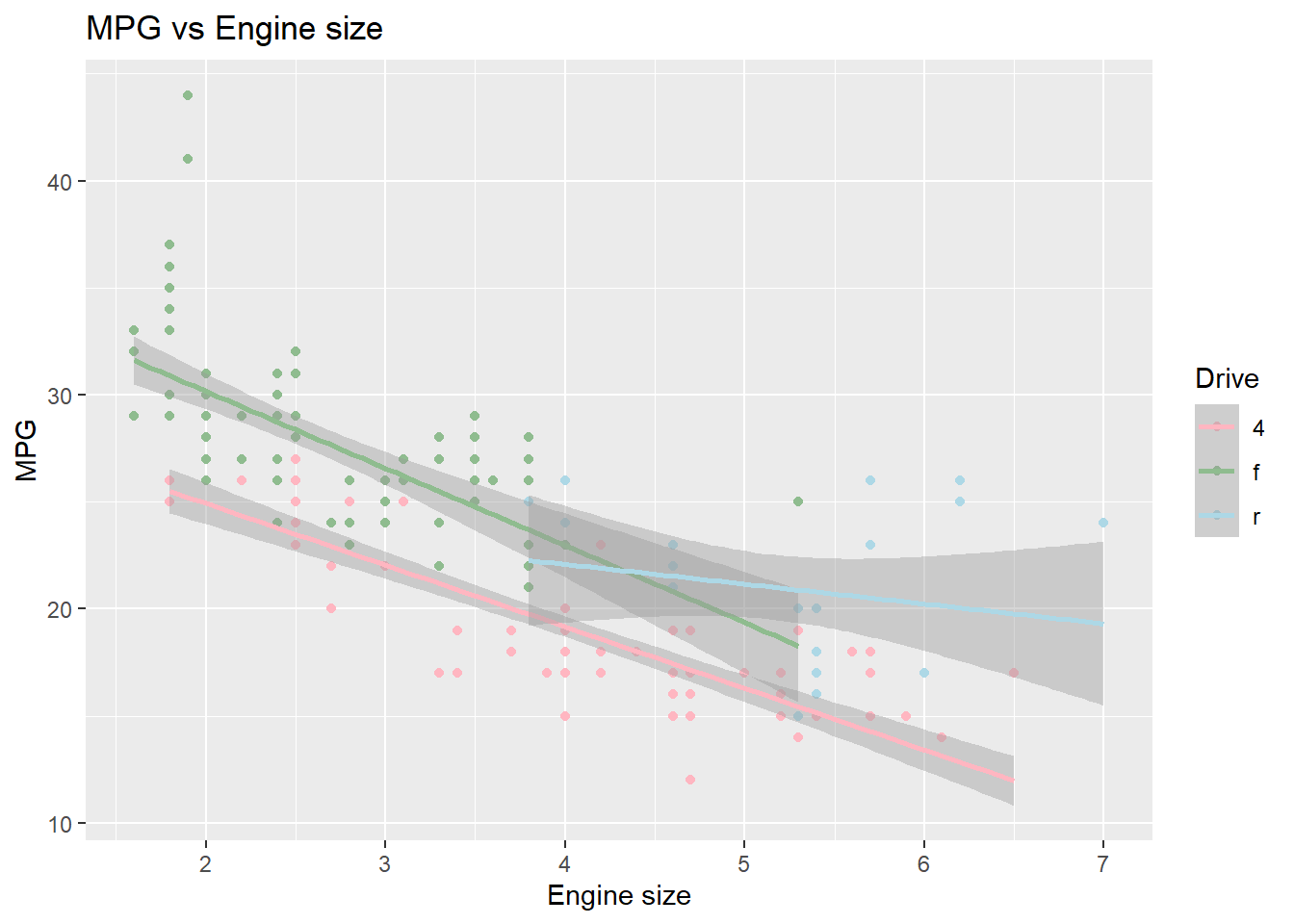# Code chunk 21
ggplot(data = mpg, mapping = aes(x = displ, y = hwy, color=cty)) + geom_point()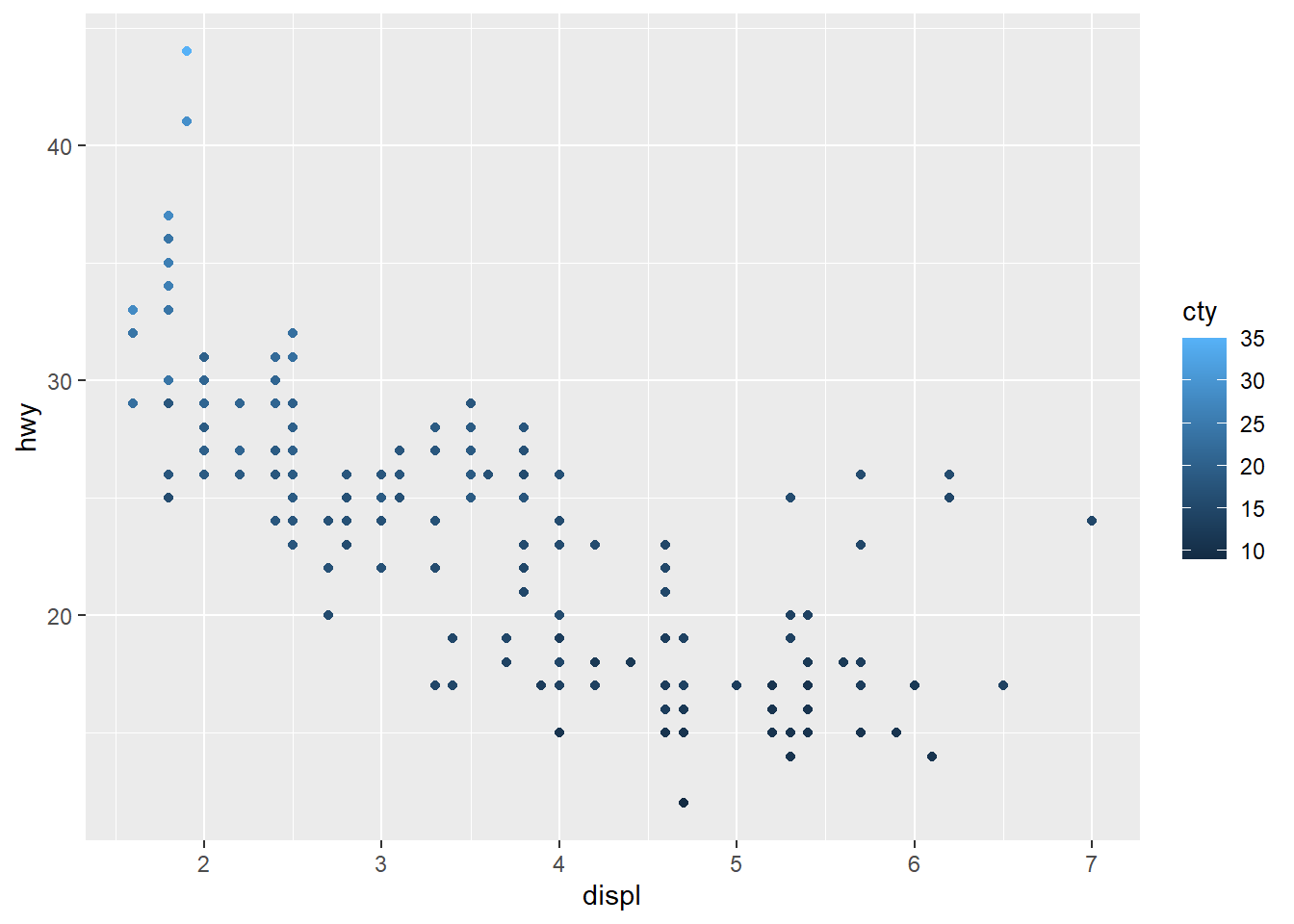# Code chunk 22:
ggplot(data = mpg, mapping = aes(x = displ, y = hwy, color=cty)) +
geom_point() +
scale_colour_gradient(name = "City MPG", low = "red", high = "blue")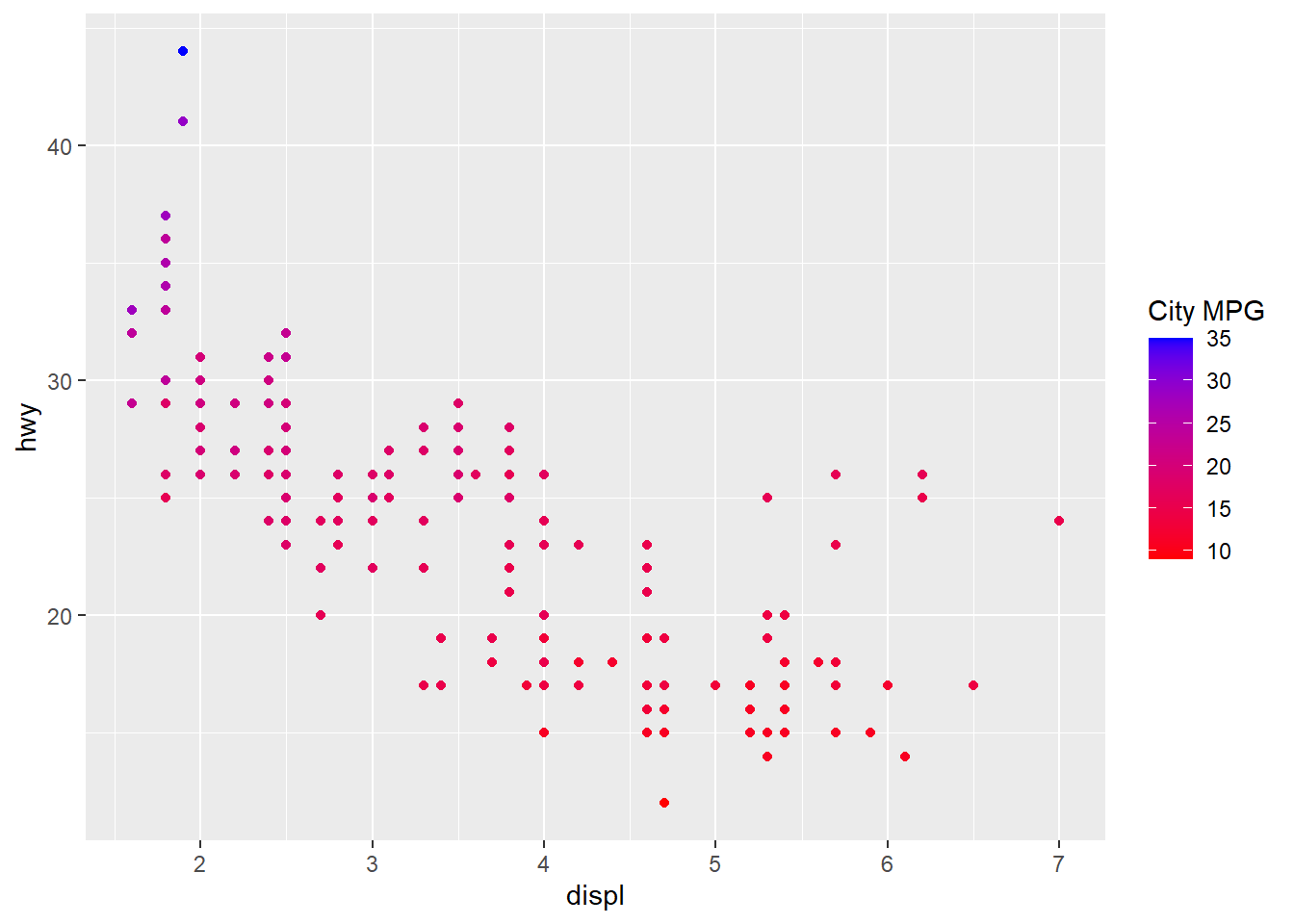### 4.7.5 Statistical transformations

# Code chunk 23: create histogram
# historam shows the distribution of a single variable.
# where does the count in the plot come from?
ggplot(data = diamonds, aes(x = carat)) + geom_histogram()
## stat_bin() using bins = 30. Pick better value with binwidth.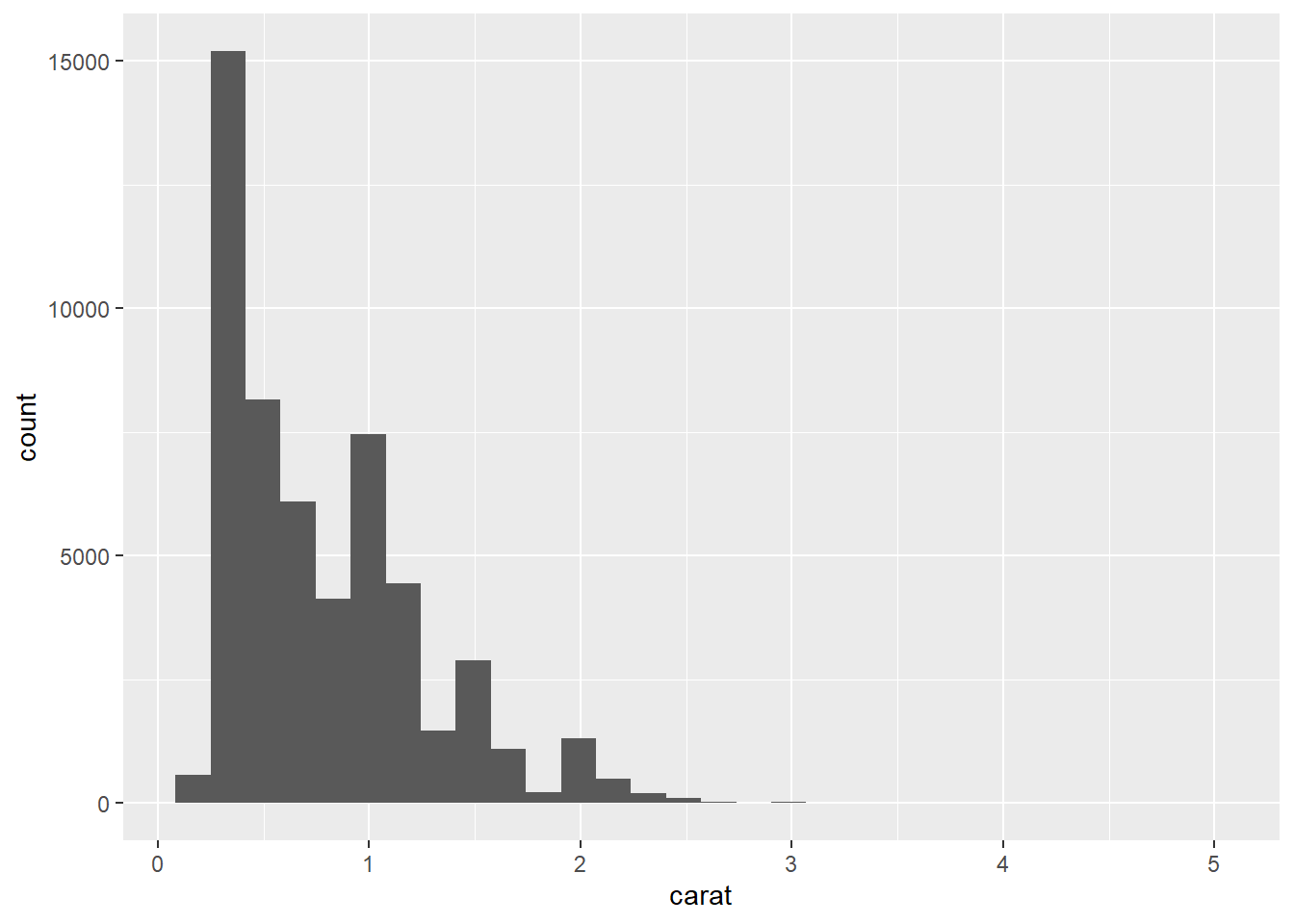• To create the histogram above, we need to calculate count (the number of diamonds in each bin or interval) from the dataset diamonds. This example tells us that some plots require new values calculated from raw data. Statistical transformations (stats for short) create new variables to plot.

• How geom_histogram() works?

• "A stat takes a dataset as input and returns a dataset as output, and so a stat can add new variables to the original dataset. For example, stat_bin, the statistic used to make histograms, produces the following variables:
• count, the number of observations in each bin
• density, the density of observations in each bin (percentage of total / bar width)
• x, the centre of the bin" [@wickham2016ggplot2]
• “These generated variables can be used instead of the variables present in the original dataset. For example, the default histogram geom assigns the height of the bars to the number of observations (count), but if you’d prefer a more traditional histogram, you can use the density (density). The following example shows a density histogram of carat from the diamonds dataset.” [@wickham2016ggplot2]
• To map the variable created by stats to aesthetics, the names of generated variables must be surrounded with ...

# Code chunk 24: create histogram with density
# the variable density is created by stat_bin()
ggplot(diamonds, aes(carat)) + geom_histogram(aes(y = ..density..), binwidth = 0.1)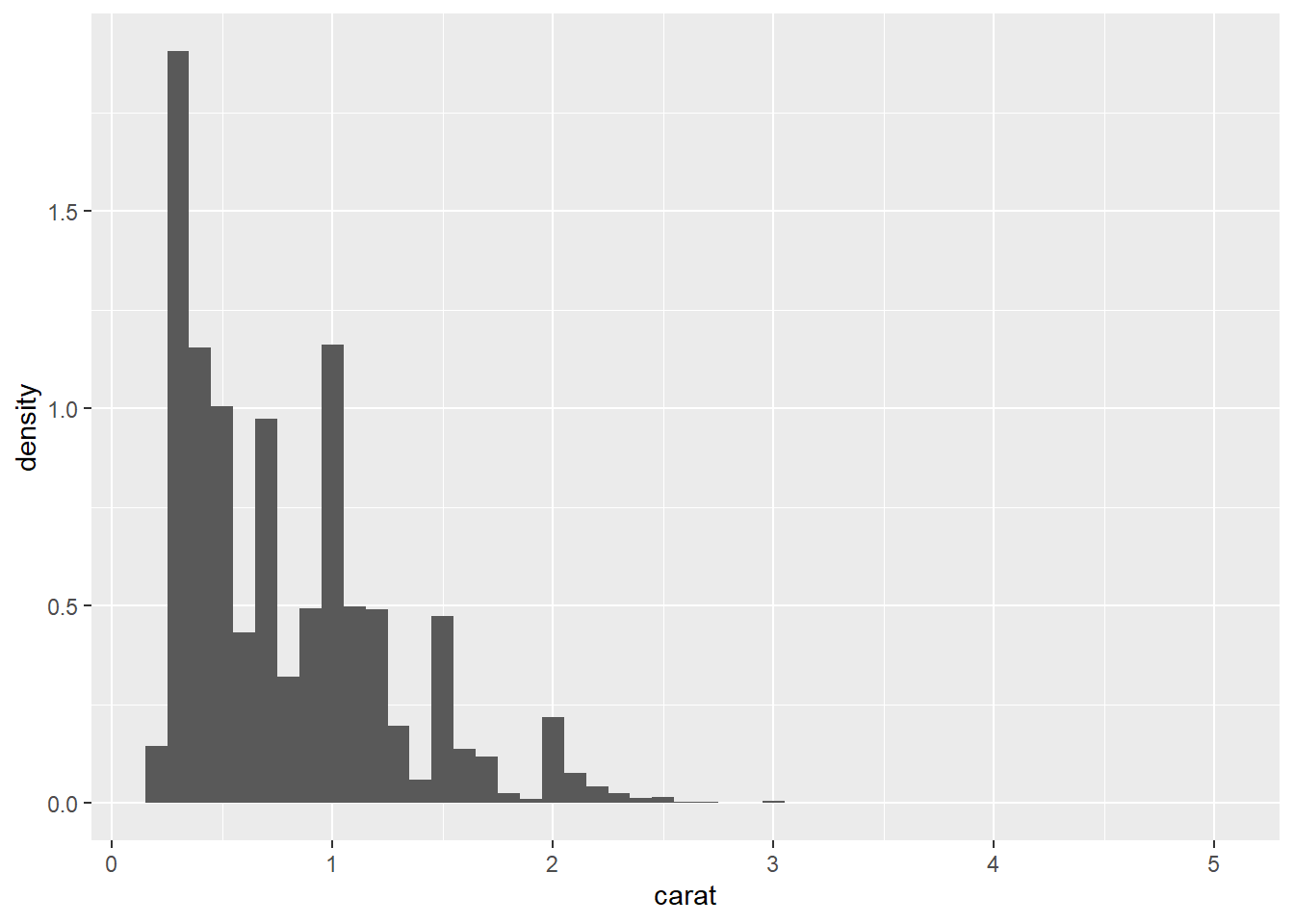• Every geom has a default stats.
# An alternative way to build a histogram using stat_bin()
ggplot(diamonds, aes(carat)) + stat_bin()
## stat_bin() using bins = 30. Pick better value with binwidth.• Position adjustments determine how to arrange geoms that would otherwise occupy the same space.
# The discrete analogue of histogram is the bar plot
s <- ggplot(mpg, aes(fl, fill = drv))
s + geom_bar()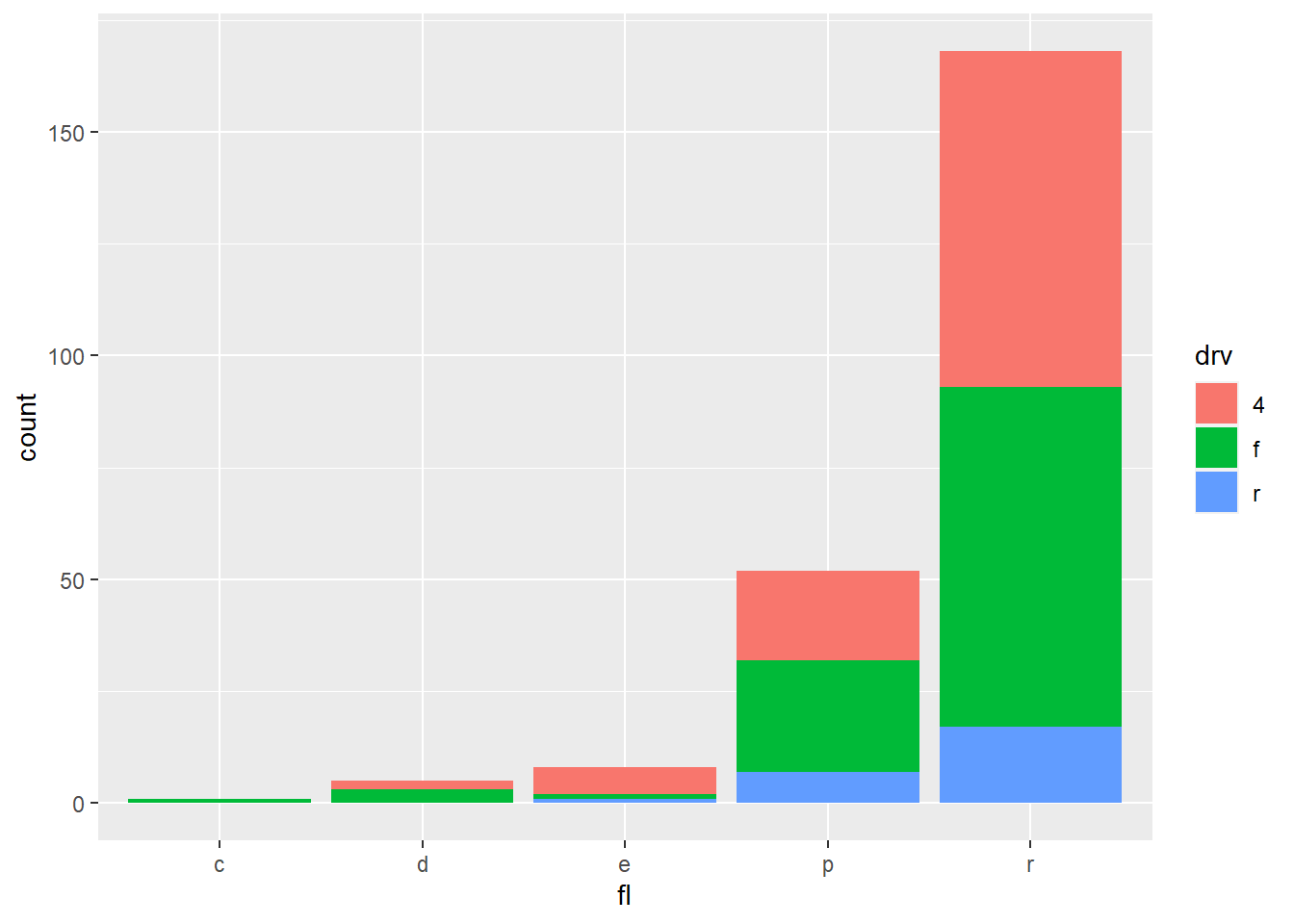# Stack elements on top of one another
s + geom_bar(position = "stack")# Arrange elements side by side
s + geom_bar(position = "dodge")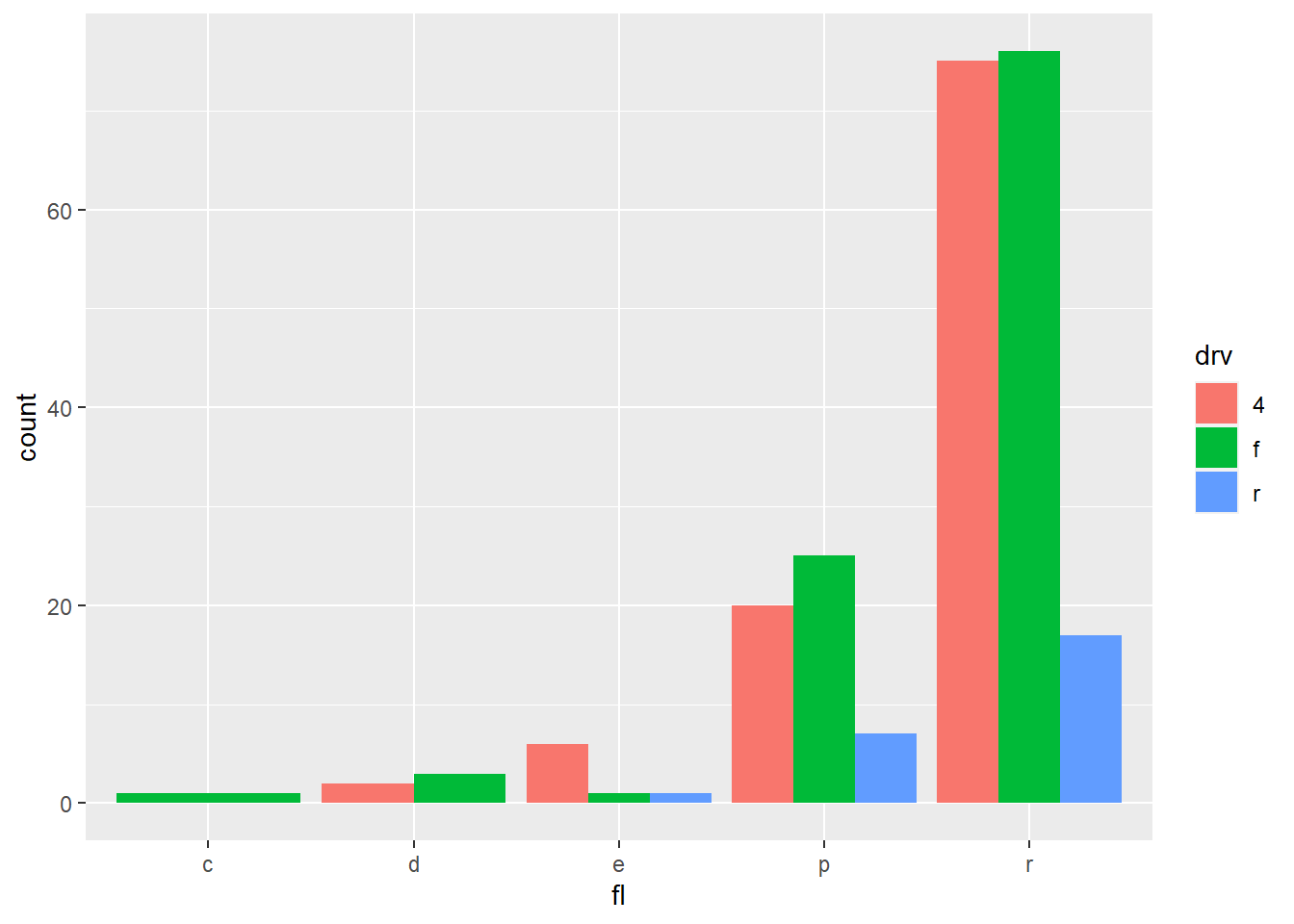# Stack elements on top of one another,normalize height
s + geom_bar(position = "fill")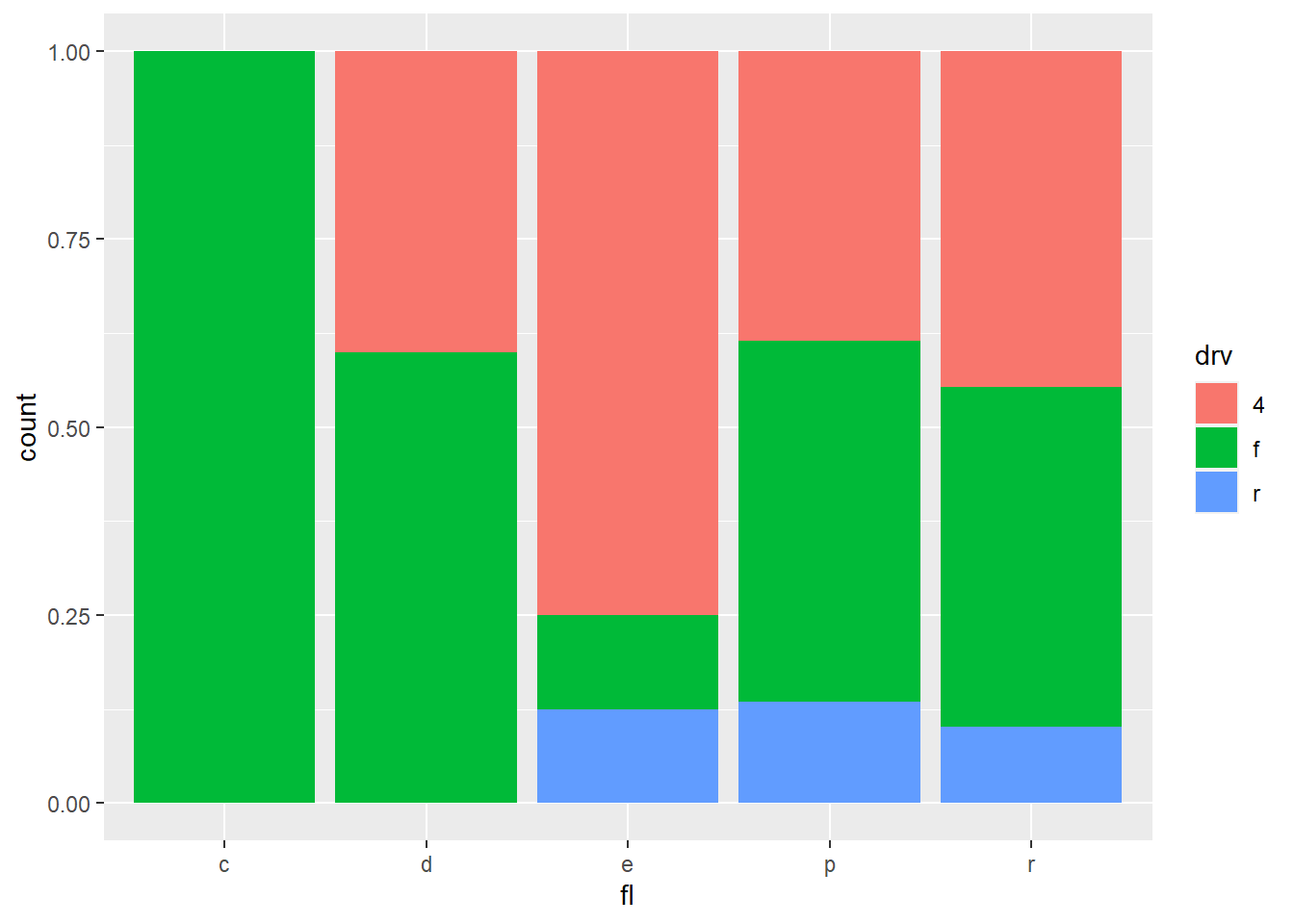### 4.7.7 A faceting

• “A faceting specification describes how to break up the data into subsets and how to display those subsets as small multiples. This is also known as conditioning or latticing/trellising.” [@wickham2016ggplot2]

• “There are two types of faceting provided by ggplot2: facet_grid and facet_wrap. Facet grid produces a 2d grid of panels defined by variables which form the rows and columns, while facet wrap produces a 1d ribbon of panels that is wrapped into 2d” [@wickham2016ggplot2]

# facet into rows
ggplot(data = diamonds, aes(x = carat)) + geom_histogram() + facet_grid(rows = vars(color))
## stat_bin() using bins = 30. Pick better value with binwidth.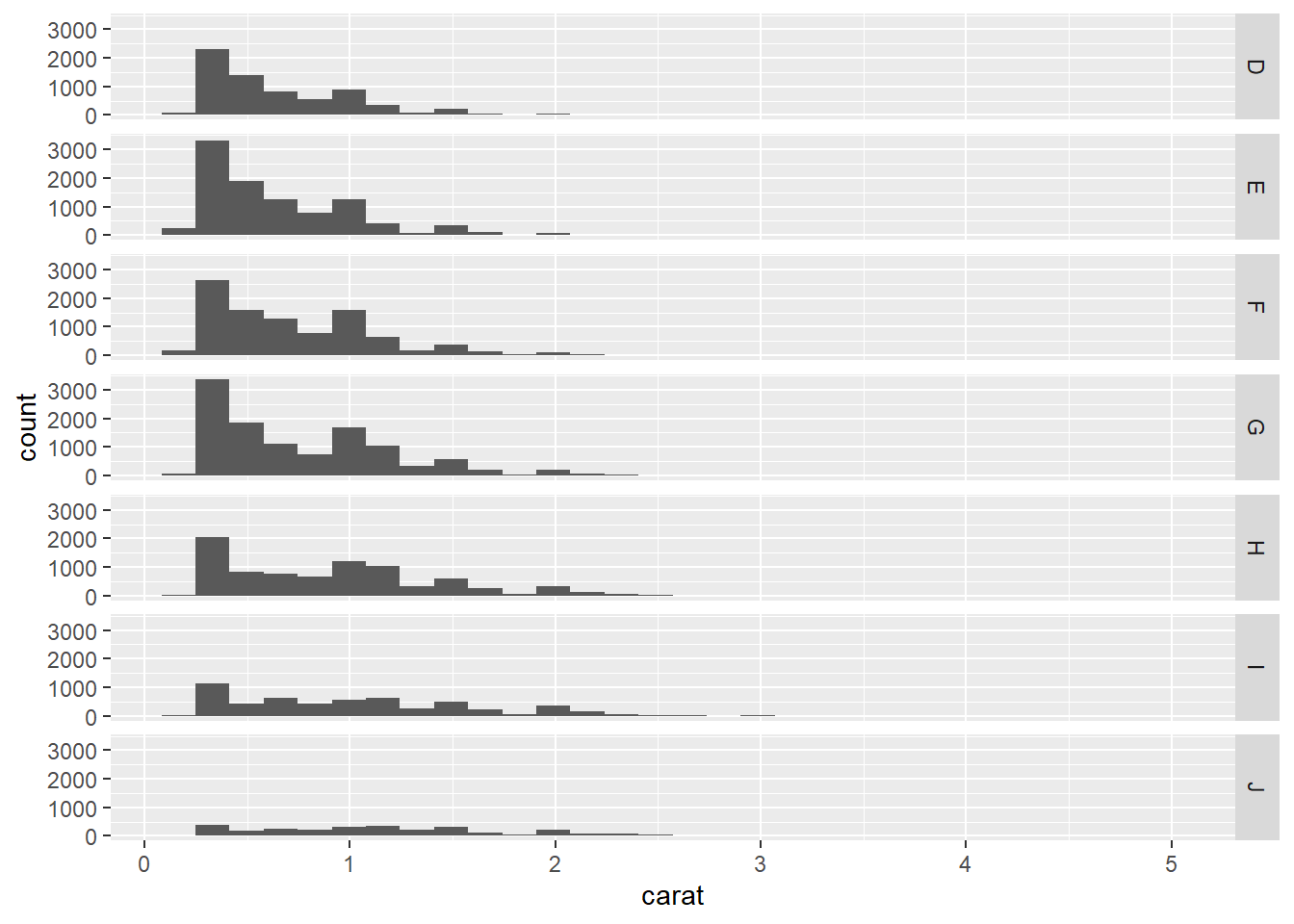# facet into columns
ggplot(data = diamonds, aes(x = carat)) + geom_histogram() + facet_grid(cols = vars(color))
## stat_bin() using bins = 30. Pick better value with binwidth.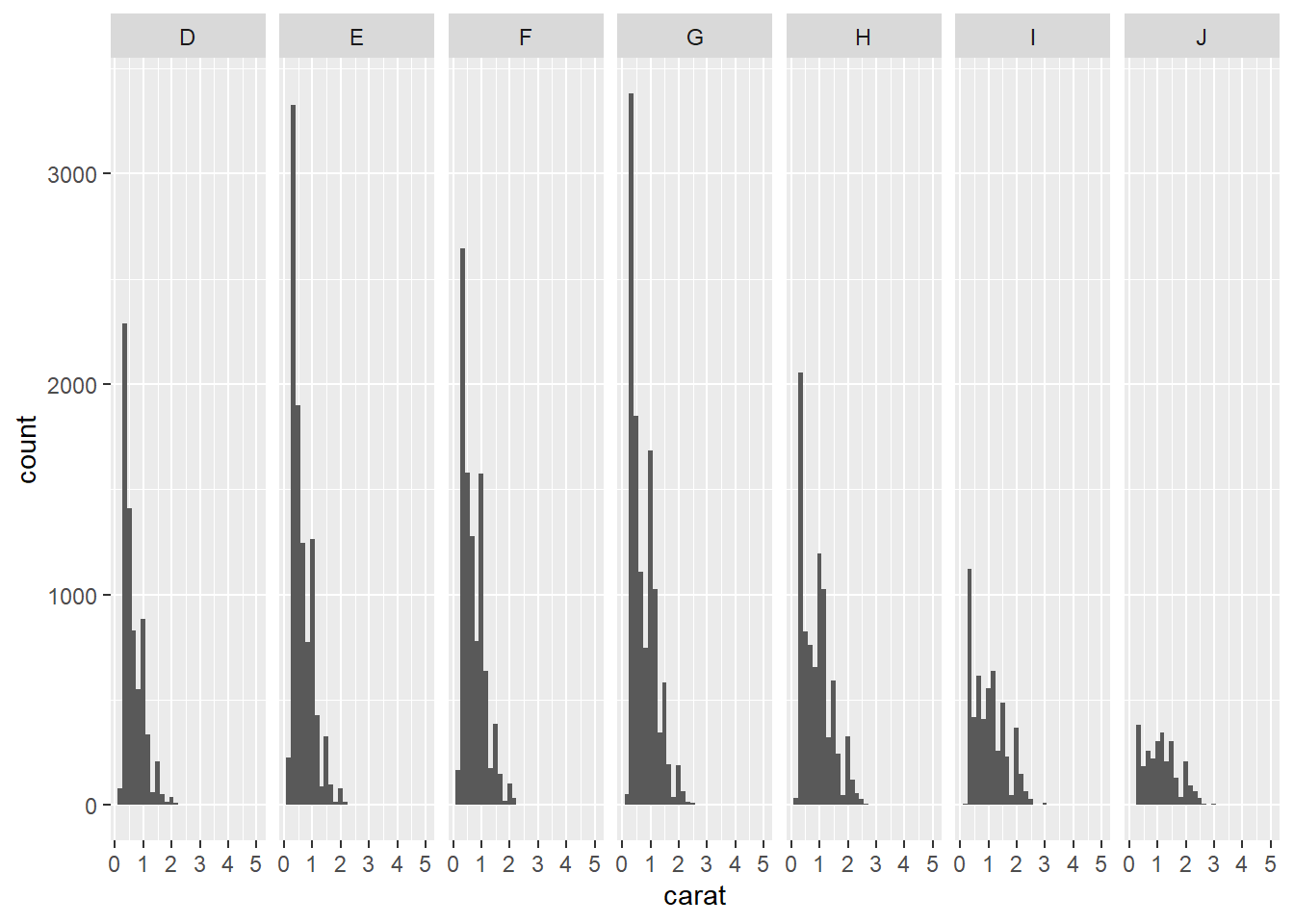Exercise 2-3. A faceting

• Using mpg data, plot a scatter plot between hwy (y) vs cty (x).

• facet into rows using cyl.

• facet into columns using cyl.

• facet into rows using cyl and columns using year

### 4.7.8 Grouping

• “In many situations, you want to separate your data into groups, but render them in the same way. When looking at the data in aggregate you want to be able to distinguish individual subjects, but not identify them. This is common in longitudinal studies with many subjects, where the plots are often descriptively called spaghetti plots.” [@wickham2016ggplot2]

• Oxboys is a dataset in the nlme package. Oxboys includes the height of a selection of boys from Oxford, England versus a standardized age.

library(nlme)
# head() will display the first 6 observations
head(Oxboys)
## Grouped Data: height ~ age | Subject
##   Subject     age height Occasion
## 1       1 -1.0000  140.5        1
## 2       1 -0.7479  143.4        2
## 3       1 -0.4630  144.8        3
## 4       1 -0.1643  147.1        4
## 5       1 -0.0027  147.7        5
## 6       1  0.2466  150.2        6
# This plot does not make sense
# x =  and y =  can be omitted within aes()
ggplot(Oxboys, aes(age, height)) + geom_line()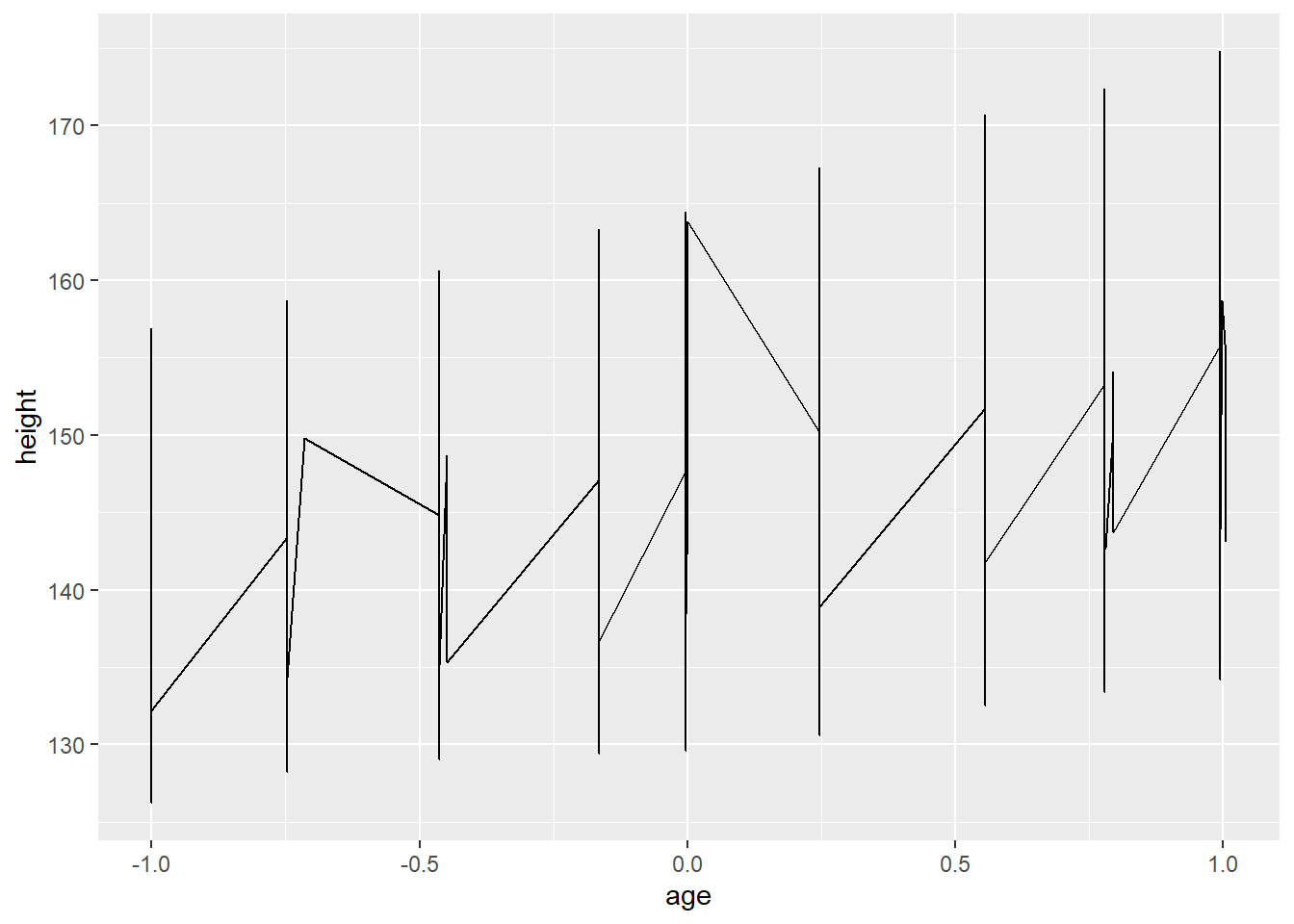# With  group = Subject, you will have a separate line for each subject
ggplot(Oxboys, aes(age, height, group = Subject)) + geom_line()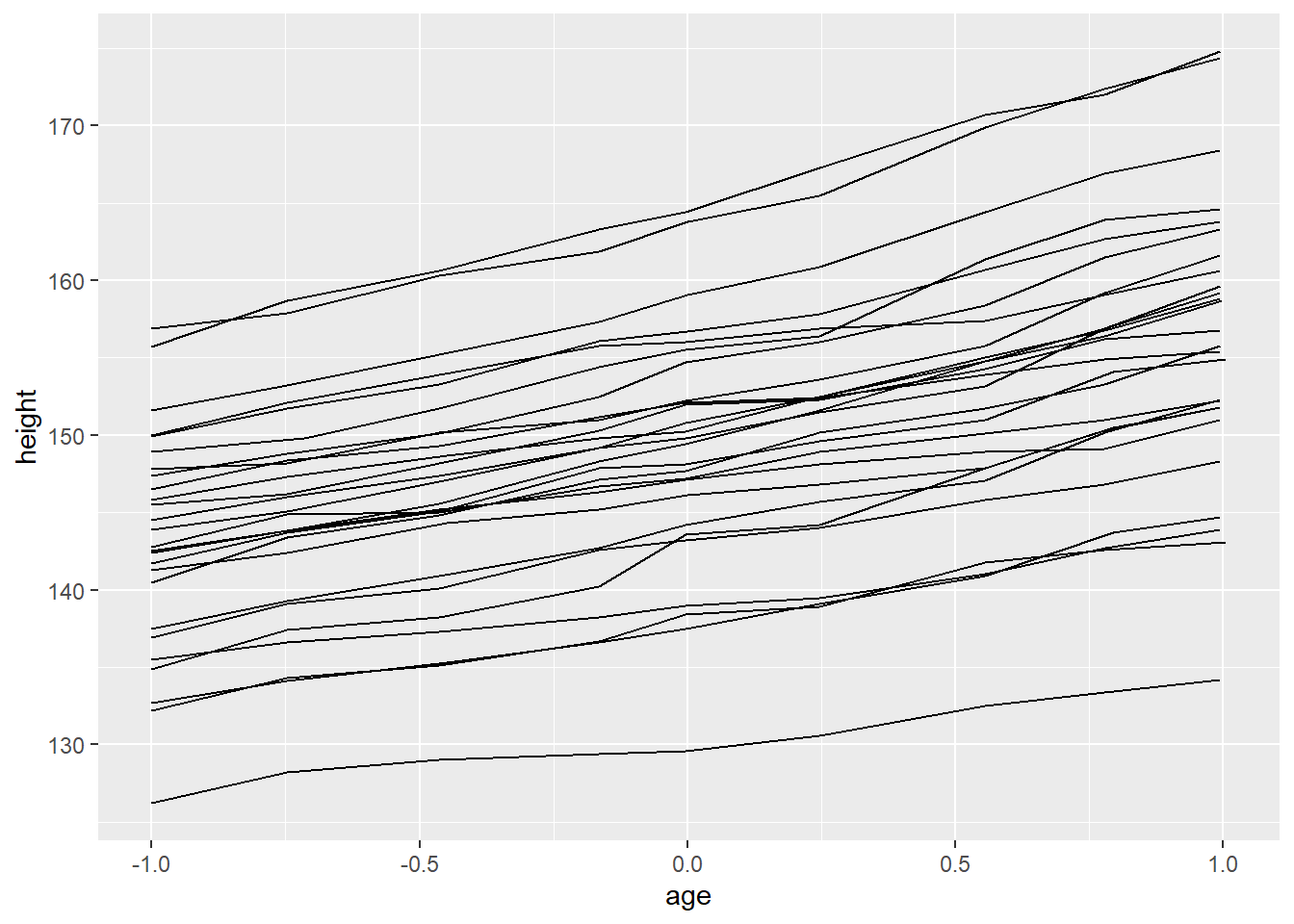# group = Subject in ggplot() will affect all the geom functions
# Therefore, a smoothed line is created for each subject
# However, in many cases, this is not what we need
# Often, we need a single smoothed line for entire subjects
ggplot(Oxboys, aes(age, height, group = Subject)) + geom_line() + geom_smooth()
## geom_smooth() using method = 'loess' and formula 'y ~ x'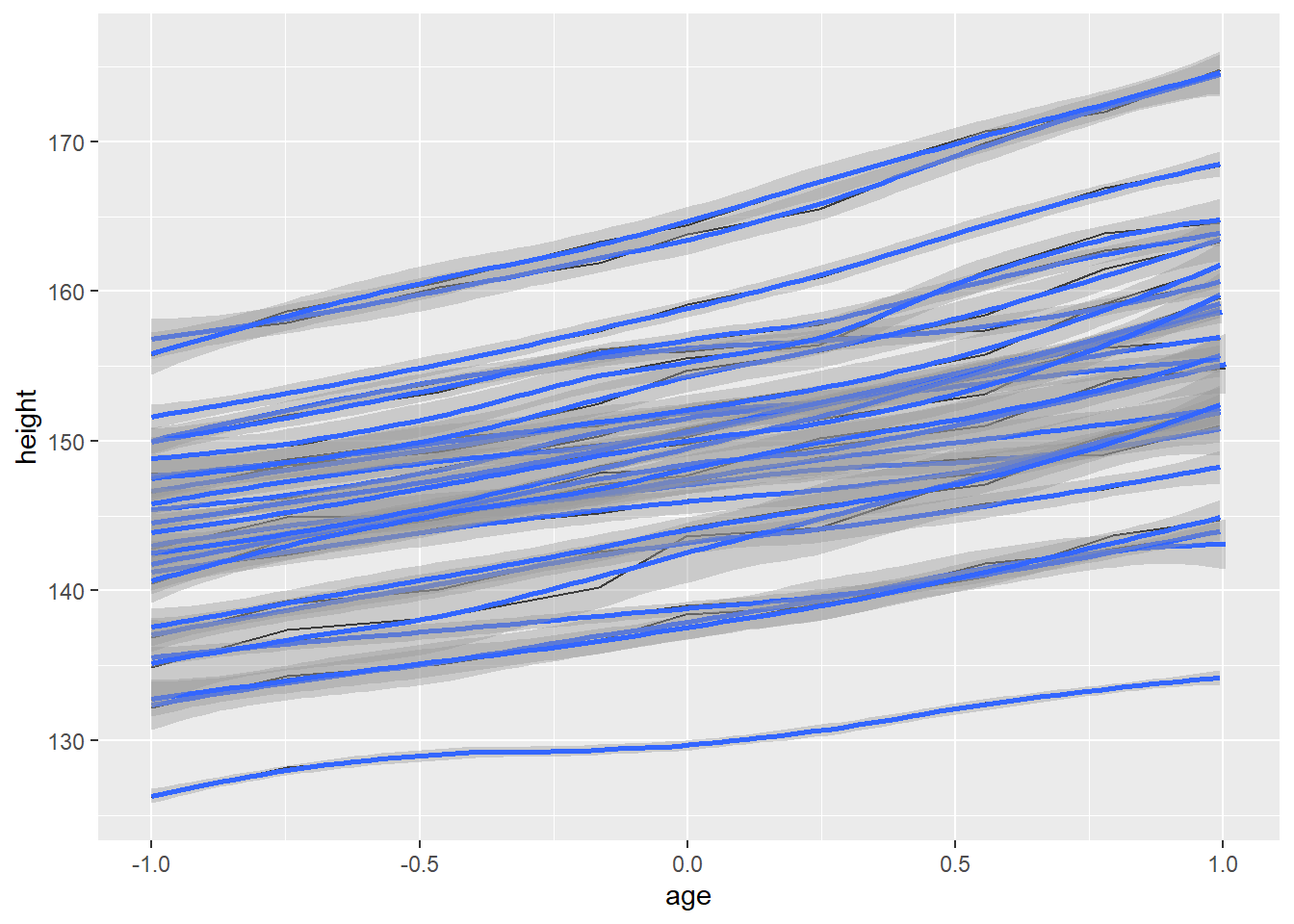# group = 1 override the default grouping
ggplot(Oxboys, aes(age, height, group = Subject)) + geom_line() + geom_smooth(aes(group = 1))
## geom_smooth() using method = 'loess' and formula 'y ~ x'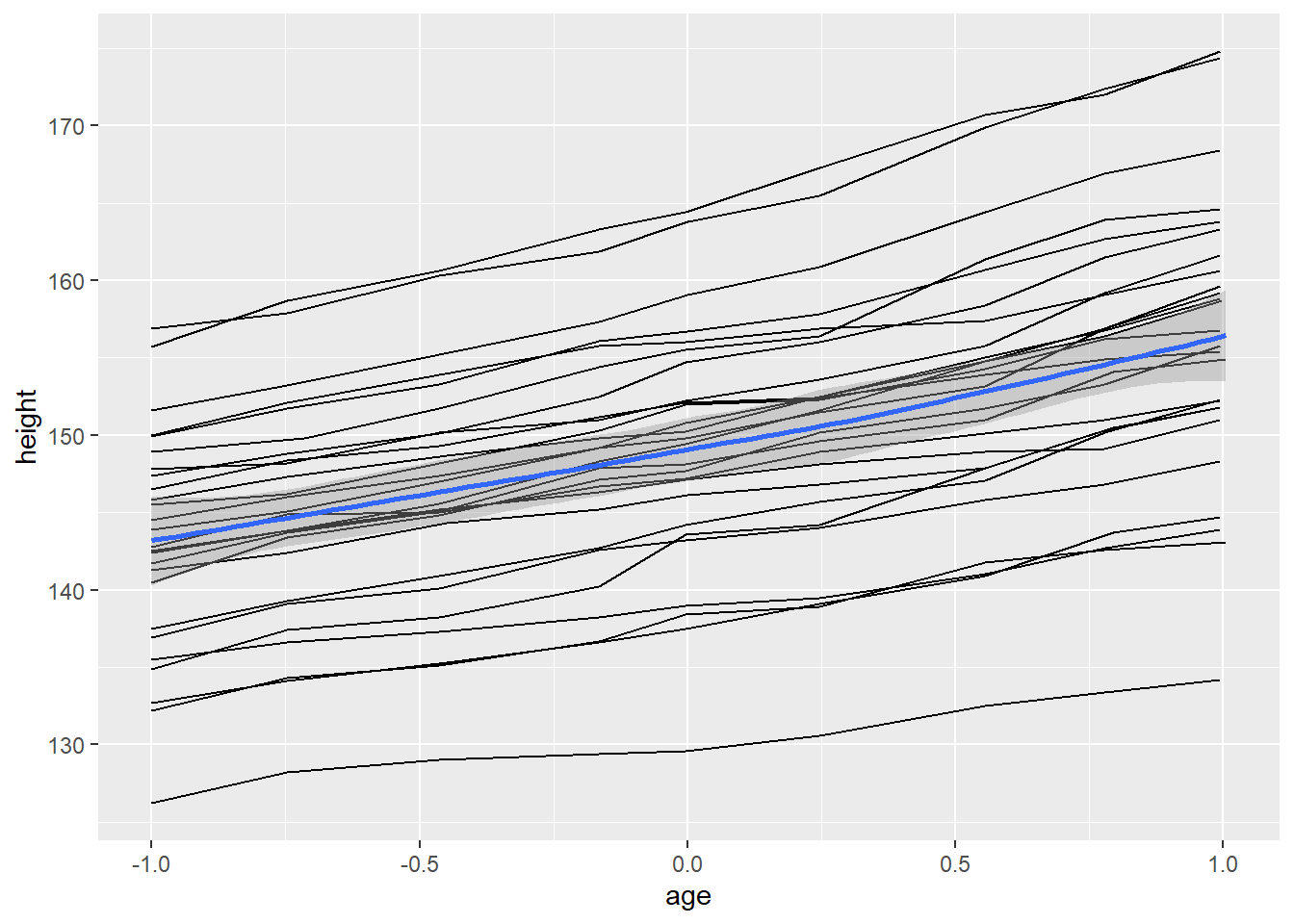# facet is also useful for visualizing longitudinal data
ggplot(Oxboys, aes(age, height)) + geom_line() + facet_wrap(vars(Subject))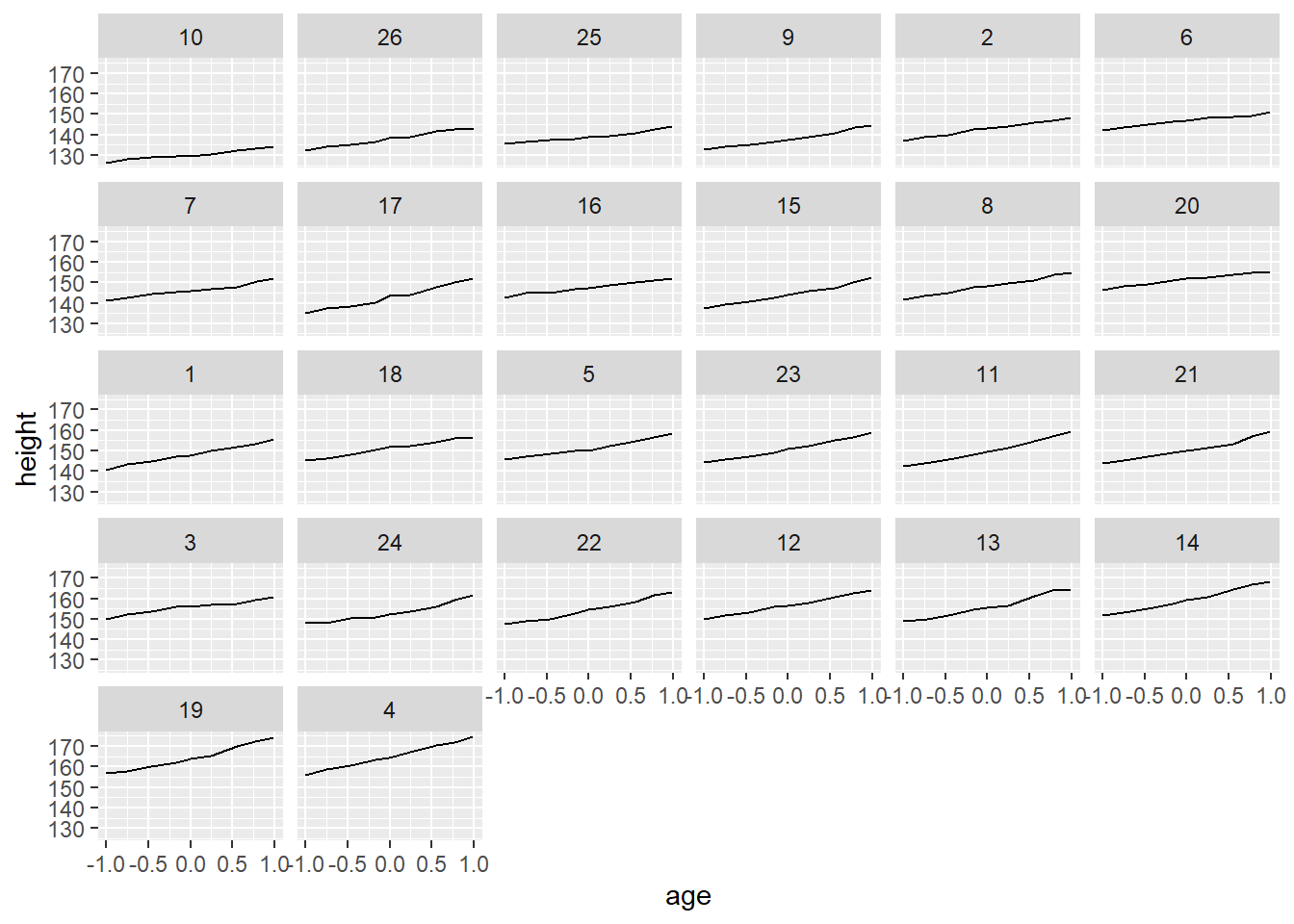## 4.8 Themes

“Themes are a powerful way to customize the non-data components of your plots: i.e. titles, labels, fonts, background, gridlines, and legends.” More details are available at https://ggplot2.tidyverse.org/reference/theme.html

ggplot(mpg, aes(x = hwy, y = cty)) + geom_point()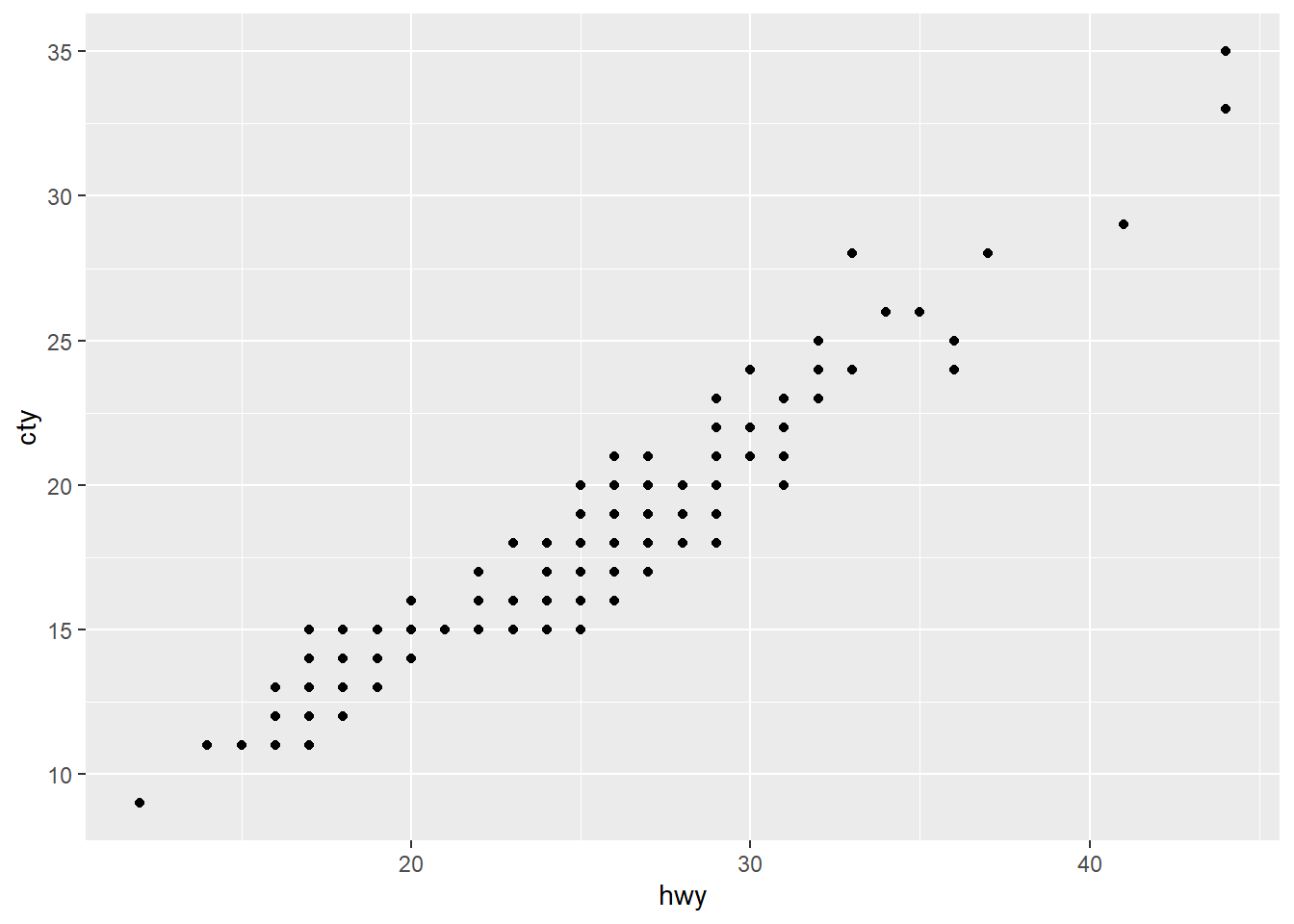ggplot(mpg, aes(x = hwy, y = cty)) + geom_point() + theme(panel.background = element_rect(fill = "white", colour = "grey50"))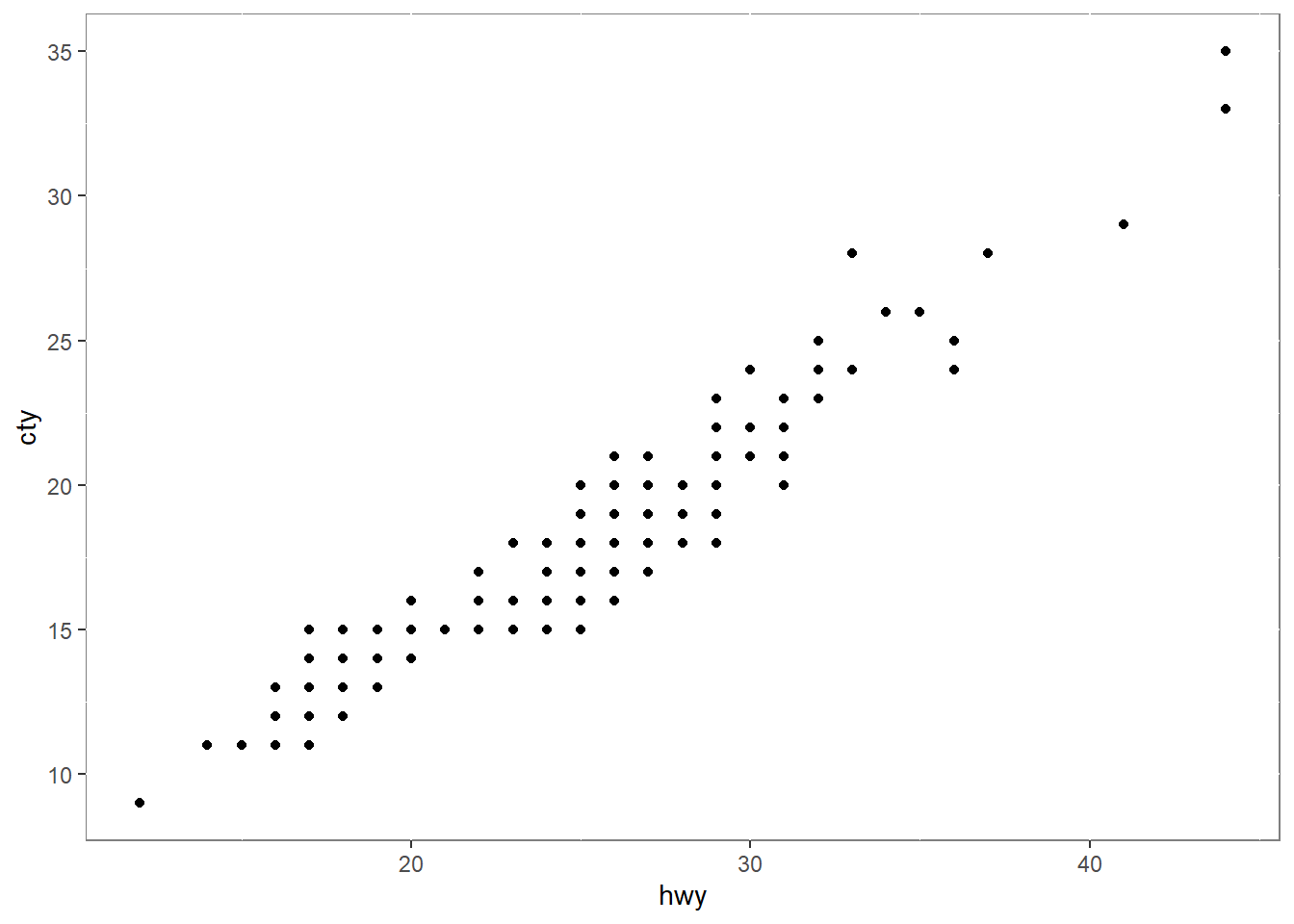ggplot(mpg, aes(x = hwy, y = cty)) + geom_point() + theme_classic()## 4.9 Saving a ggplot

• ggsave() is a convenient function for saving a plot. It defaults to saving the last plot that you displayed.
ggsave("mtcars.pdf")

## 4.10 Frequently used plots

• This section introduces some plots which are used frequently in various fields.

• We usually create plots for two reasons:

• for understanding our data
• it’s not necessary to create pretty plots
• for presenting to others
• it’s necessary to create pretty plots
• To quickly create plots without controlling the details, we use qplot() in the ggplot2 package.

### 4.10.1 qplot()

• qplot(), short for quick plot is a function in the ggplot2 package. qplot() makes it easy to produce complex plots, often requiring several lines of code using other plotting systems, in one line.

• The syntax of qplot() is qplot(<AESTHETIC MAPPGIN>, <DATA>, <GEOMS>)

### 4.10.2 Scatterplots

• scatterplots are used to show the relationship between two numerical variables.
ggplot(data = mpg, aes(x = displ, y = hwy)) + geom_point()# qplot syntax: qplot(<AESTHETIC MAPPGIN>, <DATA>, <GEOMS>)
qplot(displ, hwy, data = mpg)ggplot(data = mpg, aes(x = displ, y = hwy, color = class)) + geom_point()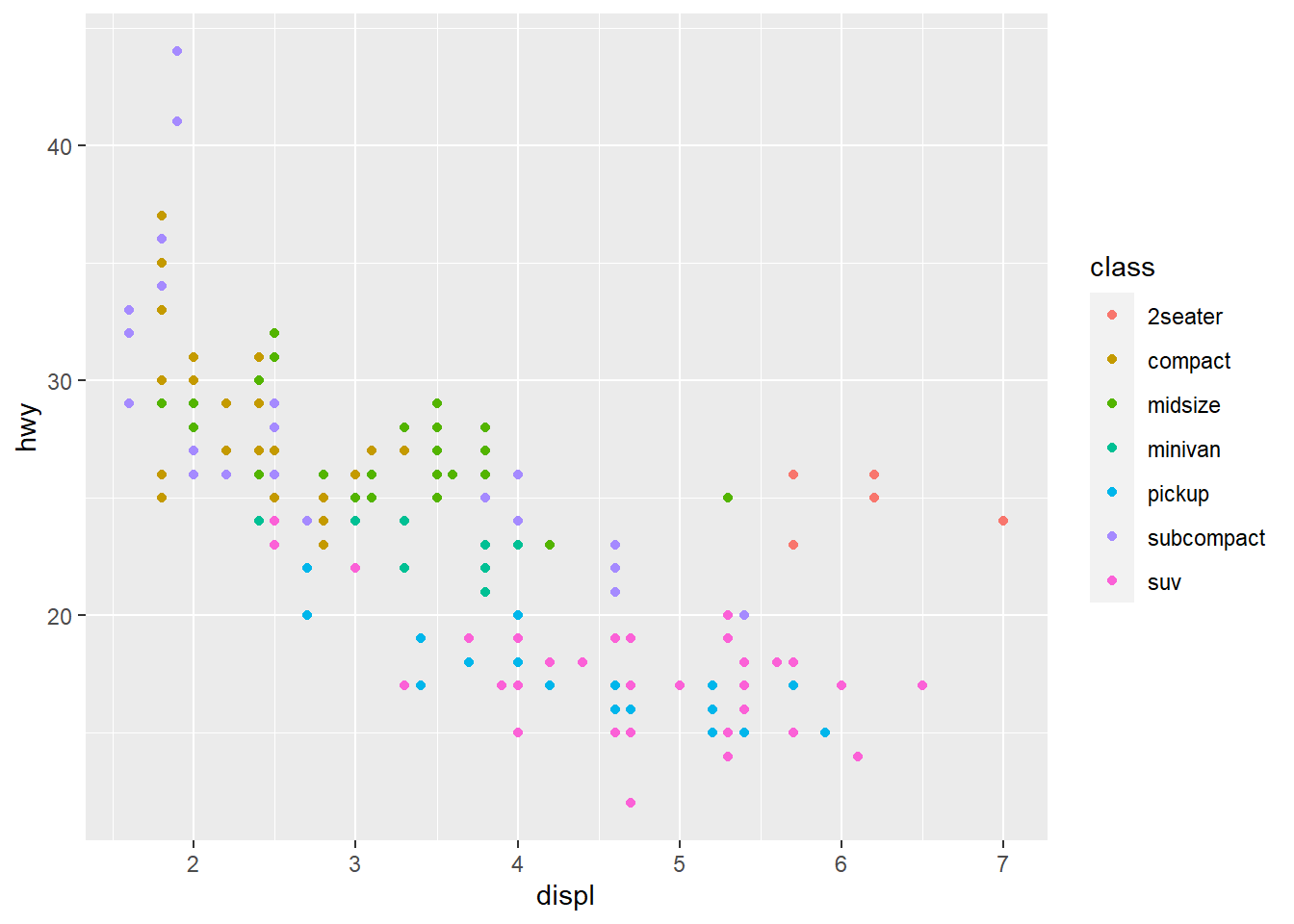# qplot syntax: qplot(<AESTHETIC MAPPGIN>, <DATA>, <GEOMS>)
qplot(displ, hwy, data = mpg, color = class)ggplot(data = mpg, aes(x = displ, y = hwy, color = class, shape = drv)) + geom_point()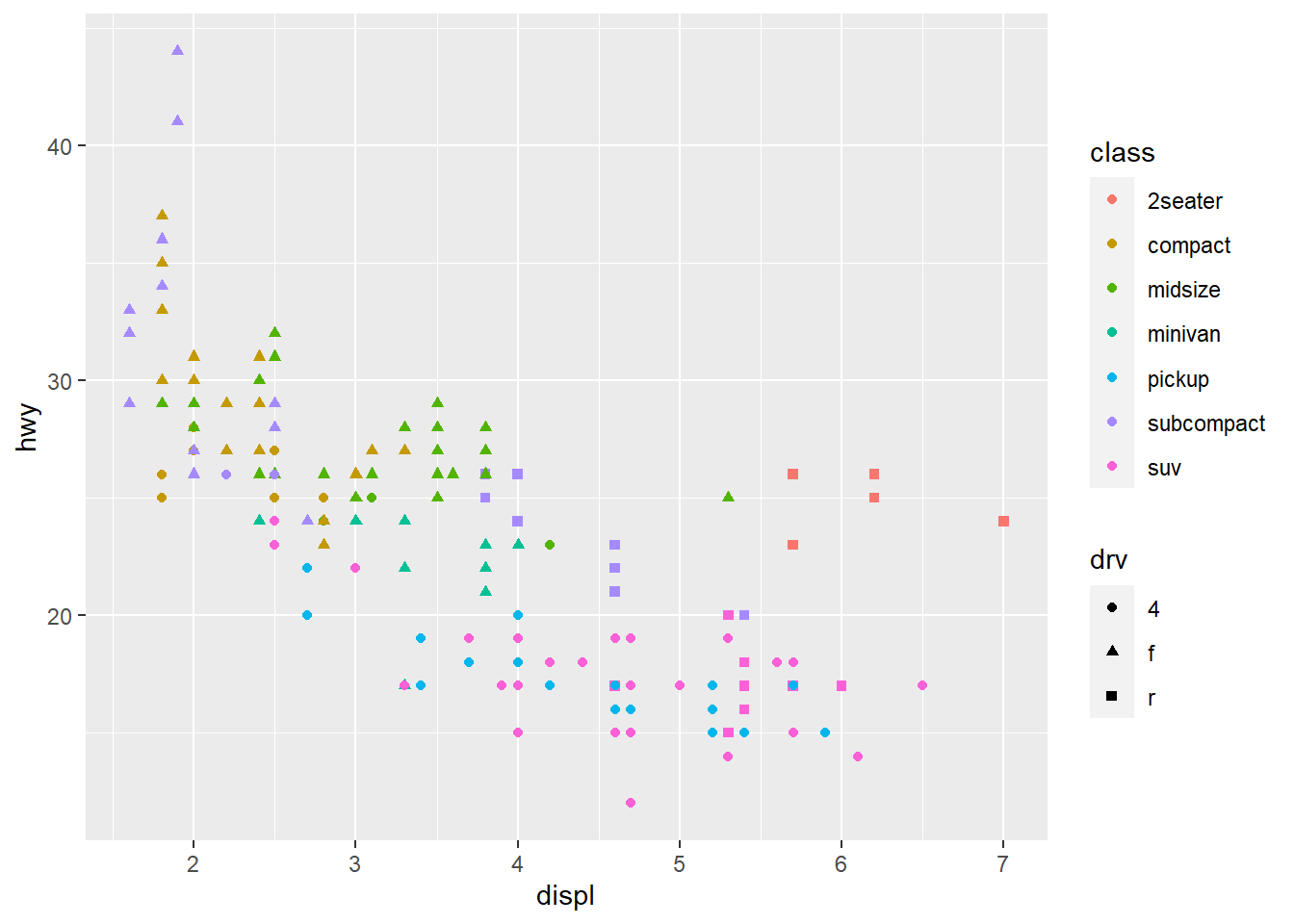# qplot syntax: qplot(<AESTHETIC MAPPGIN>, <DATA>, <GEOMS>)
qplot(displ, hwy, data = mpg, color = class, shape = drv)ggplot(data = mpg, aes(x = displ, y = hwy)) + geom_point() + geom_smooth(method = "lm")
## geom_smooth() using formula 'y ~ x'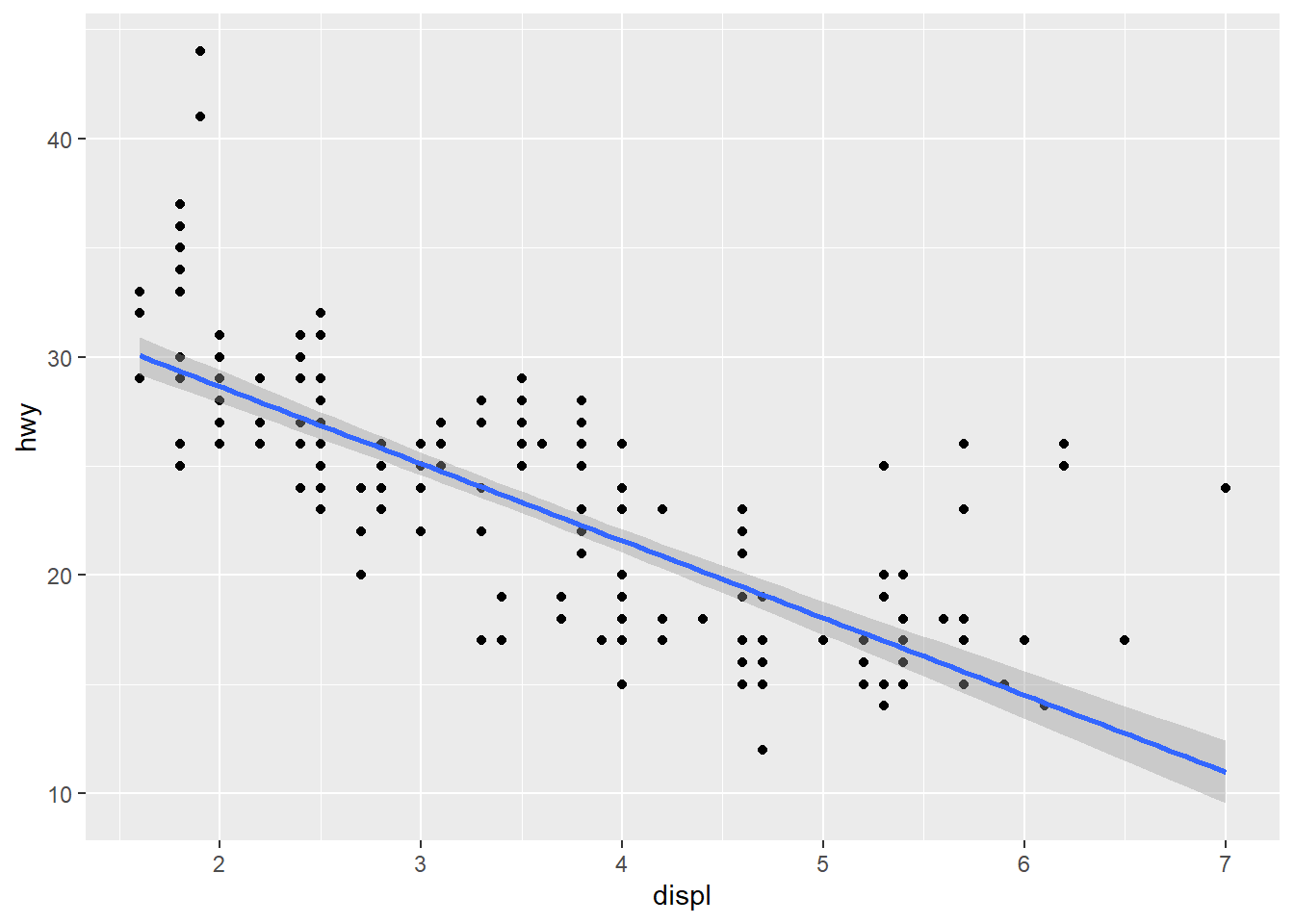# qplot syntax: qplot(<AESTHETIC MAPPGIN>, <DATA>, <GEOMS>)
qplot(displ, hwy, data = mpg, geom = c("point", "smooth")) 
## geom_smooth() using method = 'loess' and formula 'y ~ x'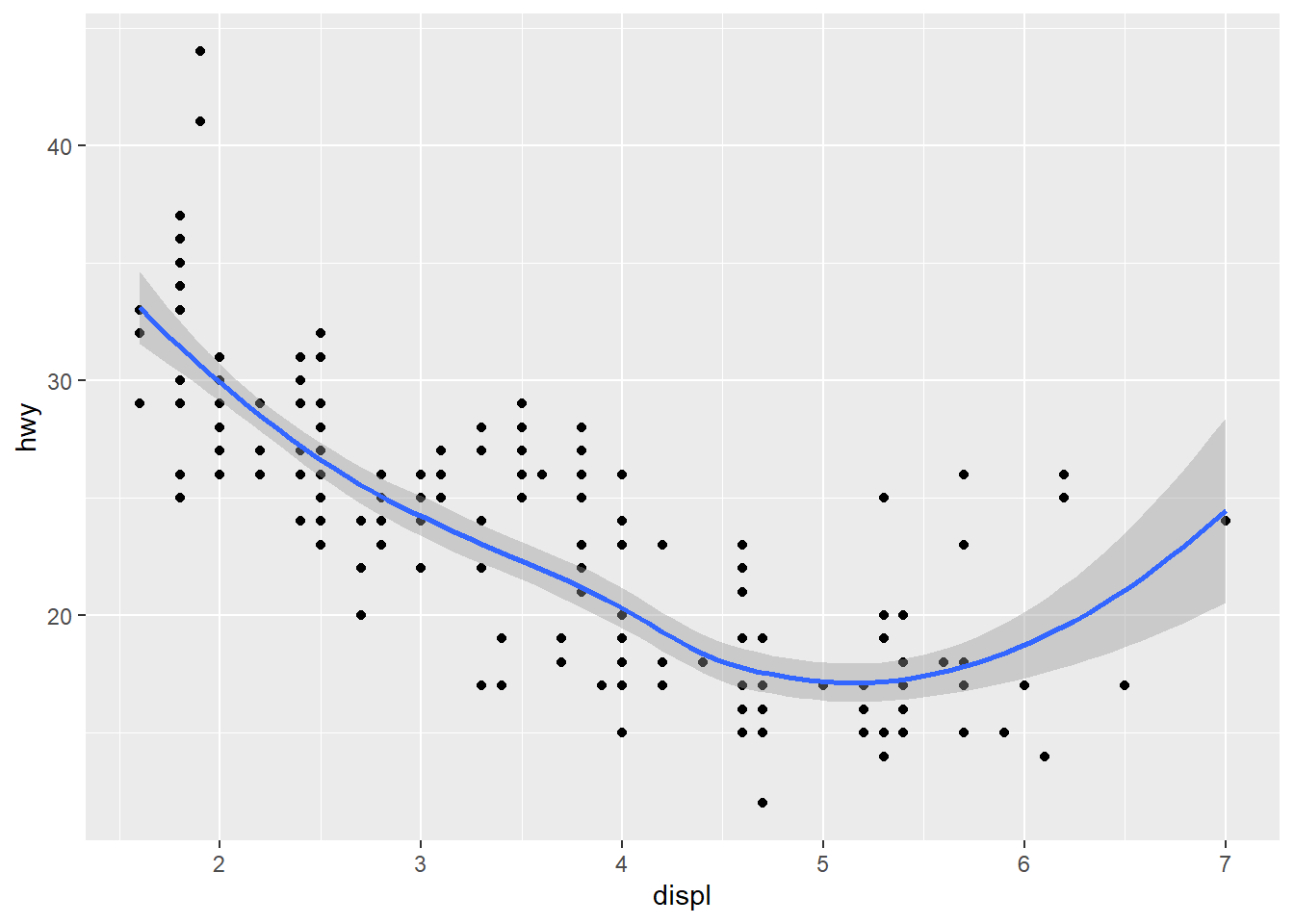### 4.10.3 Histogram

• Histograms are used to show the distribution of a numerical variable.
ggplot(data = diamonds, aes(x = carat)) + geom_histogram()
## stat_bin() using bins = 30. Pick better value with binwidth.qplot(carat, data = diamonds, geom = "histogram")
## stat_bin() using bins = 30. Pick better value with binwidth.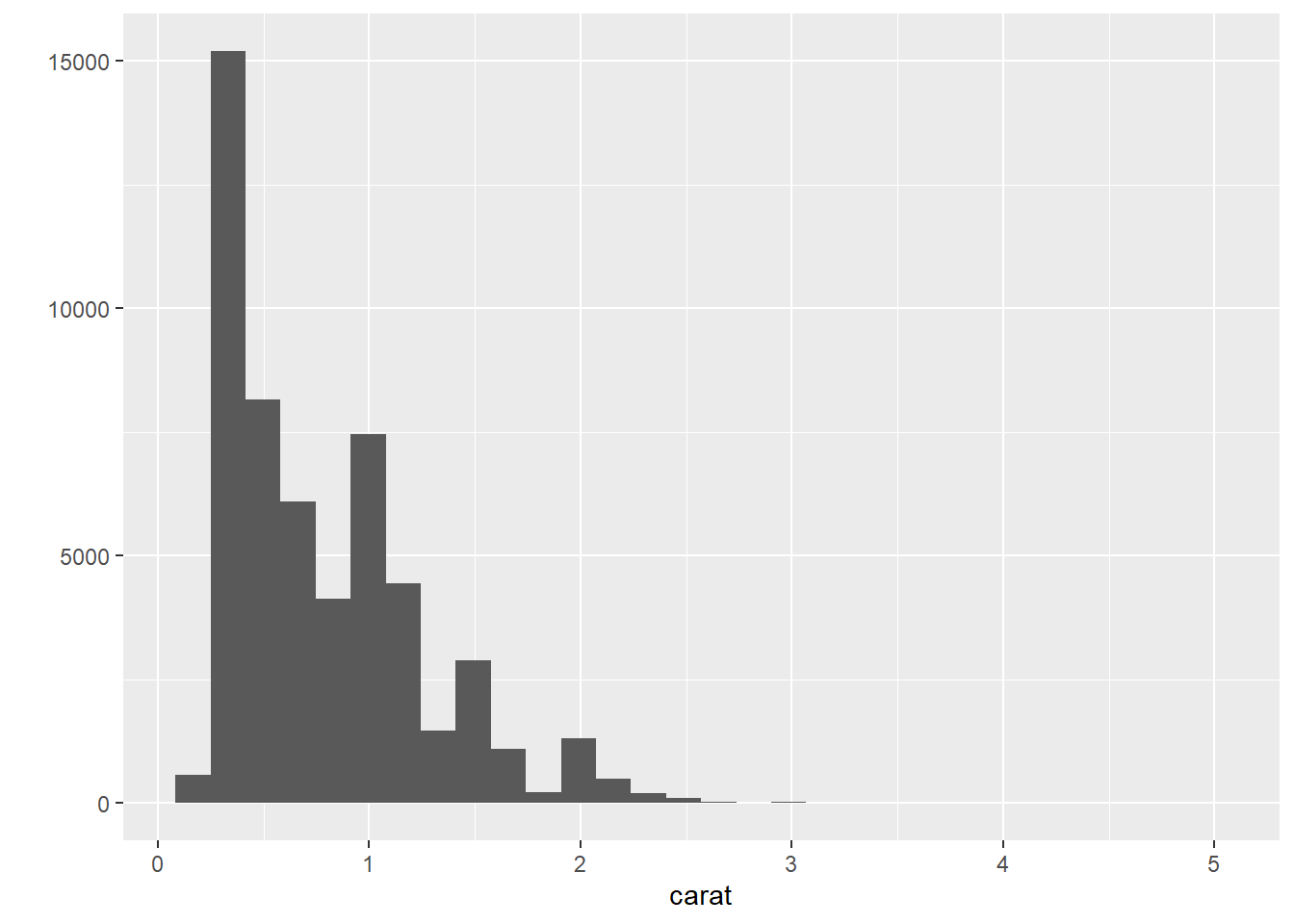# binwidth controls the width of bins
ggplot(data = diamonds, aes(x = carat)) + geom_histogram(binwidth = 0.05) + xlim(c(0,3))
## Warning: Removed 32 rows containing non-finite values (stat_bin).
## Warning: Removed 2 rows containing missing values (geom_bar).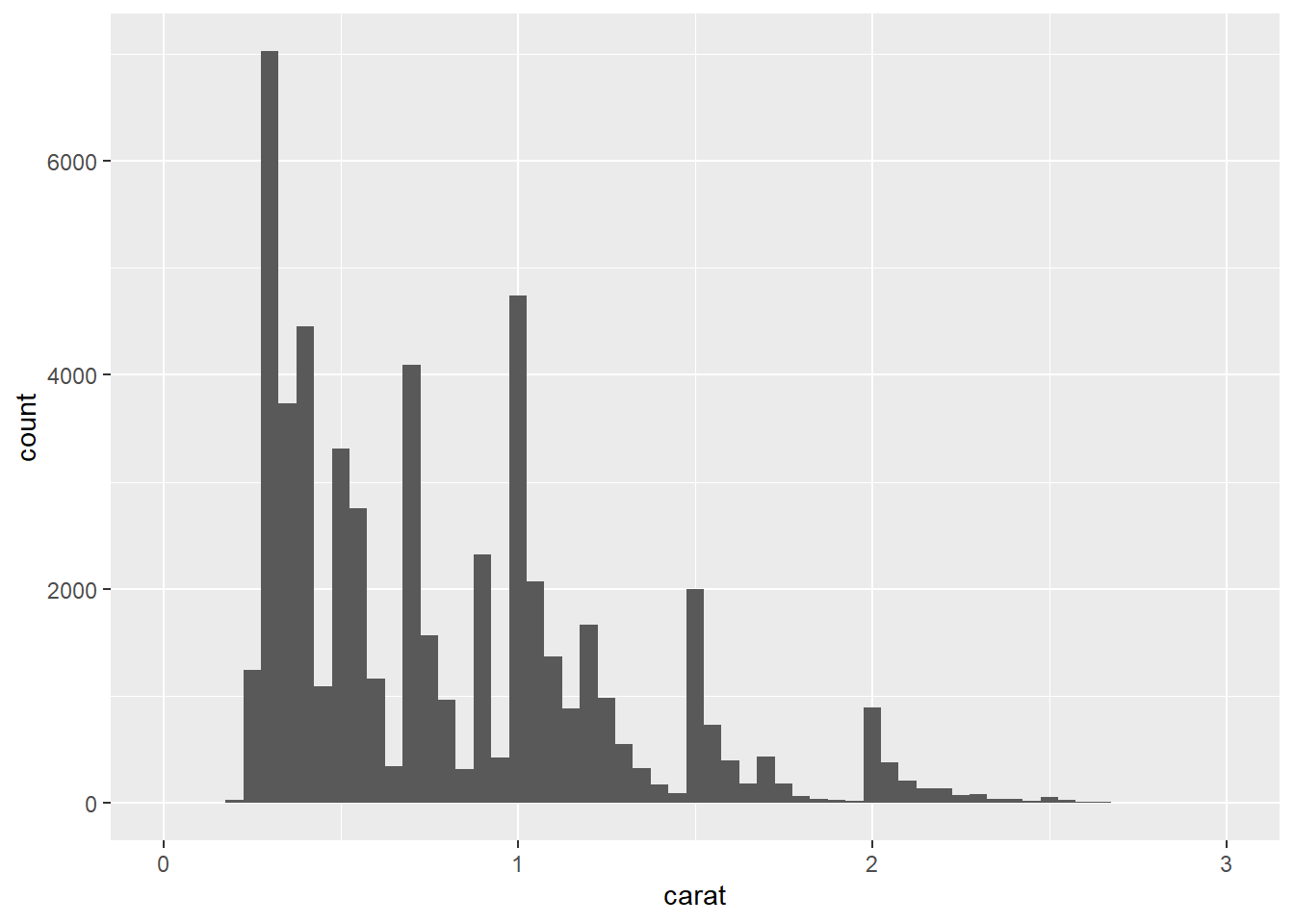qplot(carat, data = diamonds, geom = "histogram", binwidth = 0.05, xlim = c(0,3))
## Warning: Removed 32 rows containing non-finite values (stat_bin).
## Warning: Removed 2 rows containing missing values (geom_bar).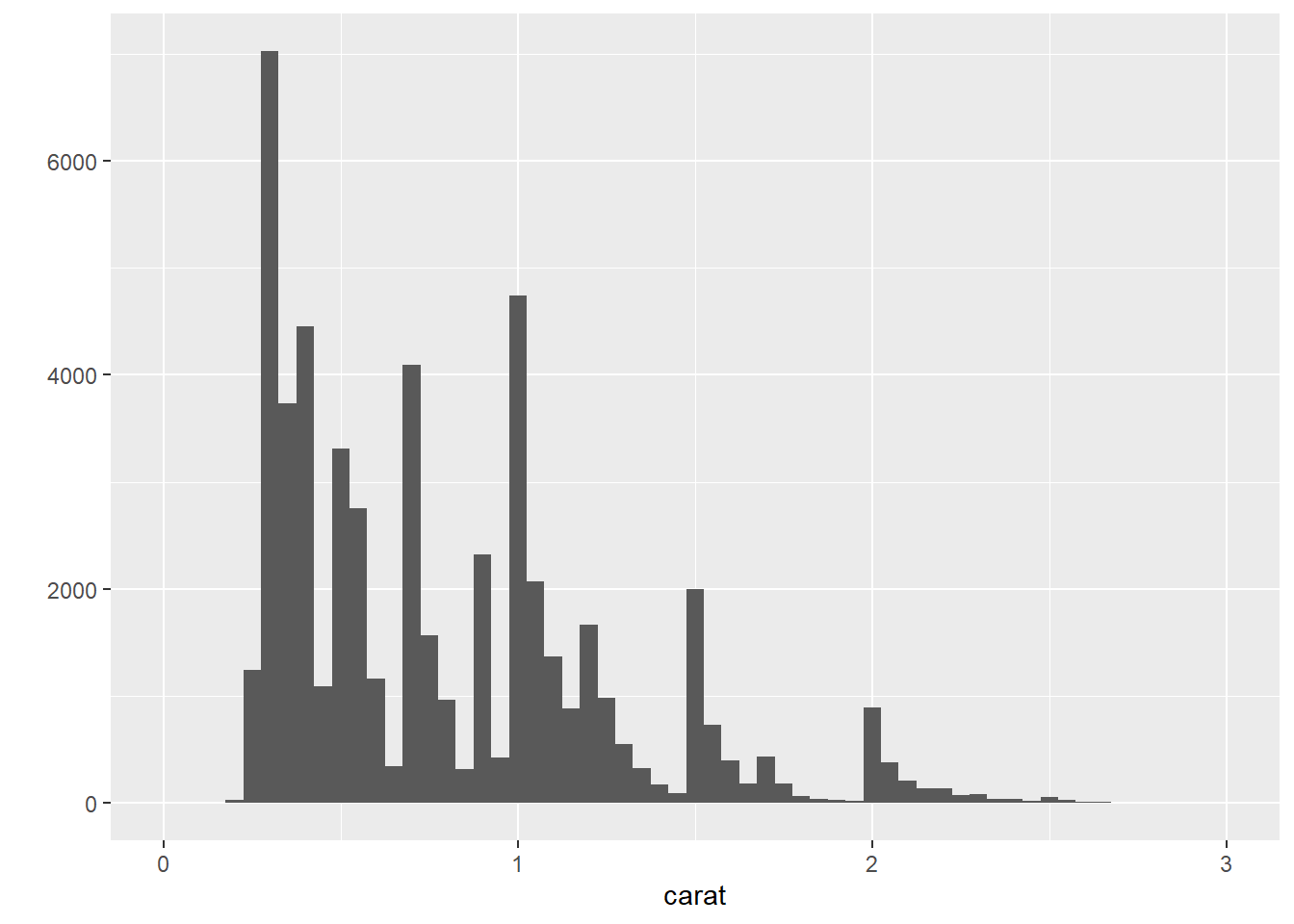ggplot(data = mpg, aes(x = hwy, fill = drv)) + geom_histogram() 
## stat_bin() using bins = 30. Pick better value with binwidth.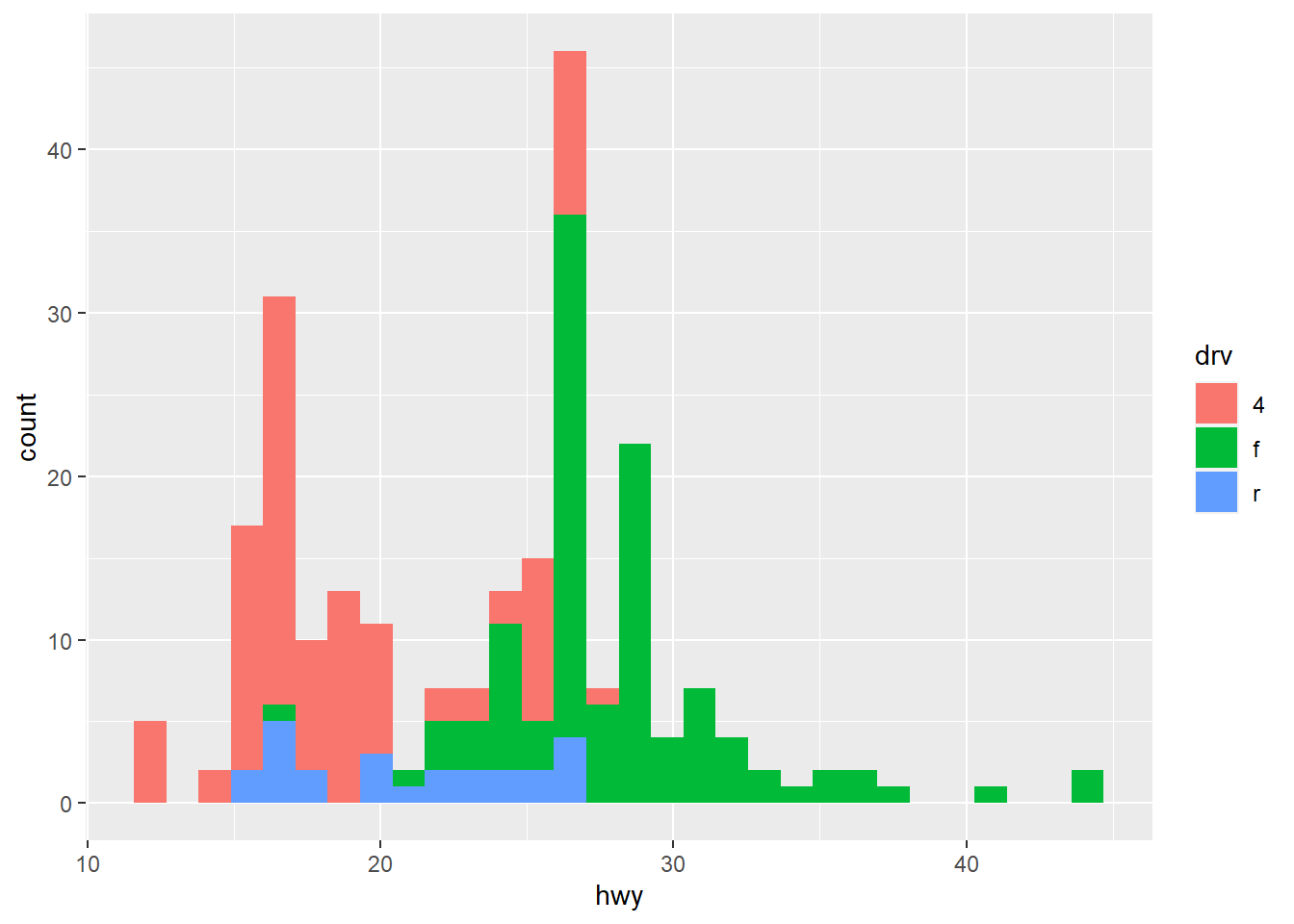qplot(hwy, data = mpg, geom = "histogram", fill = drv)
## stat_bin() using bins = 30. Pick better value with binwidth.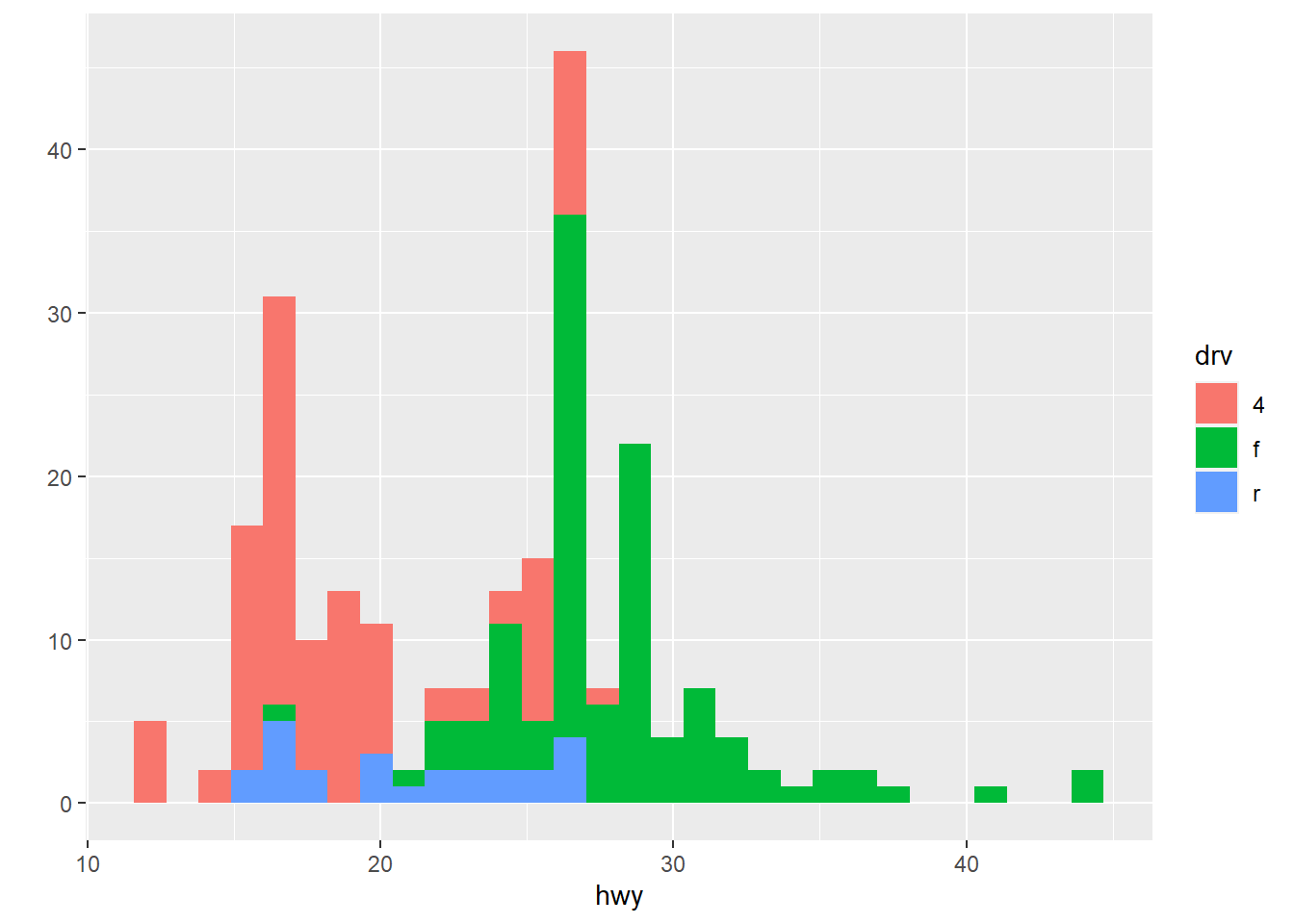### 4.10.4 Density plots

• Density plots are used to show the distribution of a numerical variable.
ggplot(data = diamonds, aes(x = carat, color = color)) + geom_density()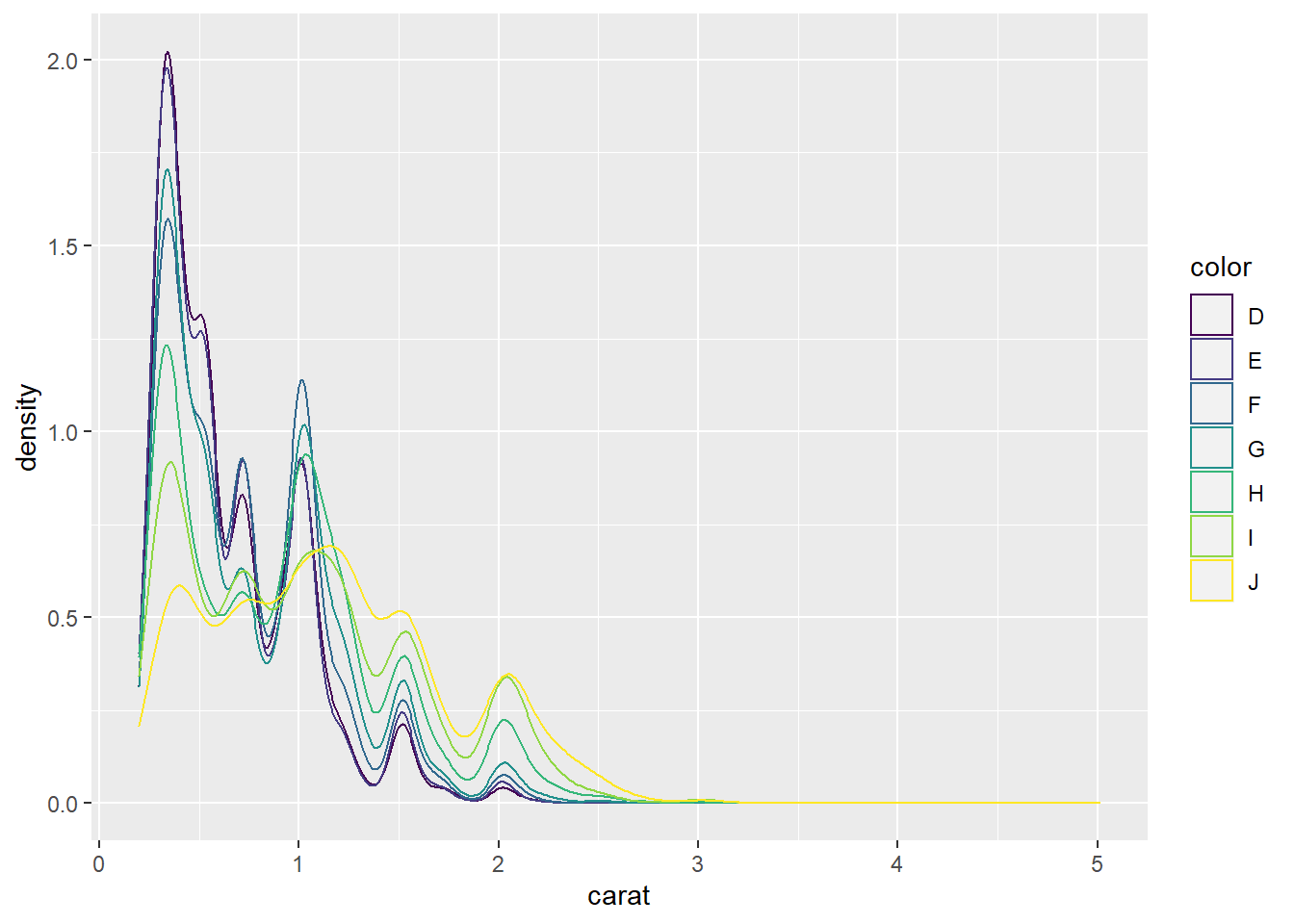qplot(carat, data = diamonds, geom = "density", color = color)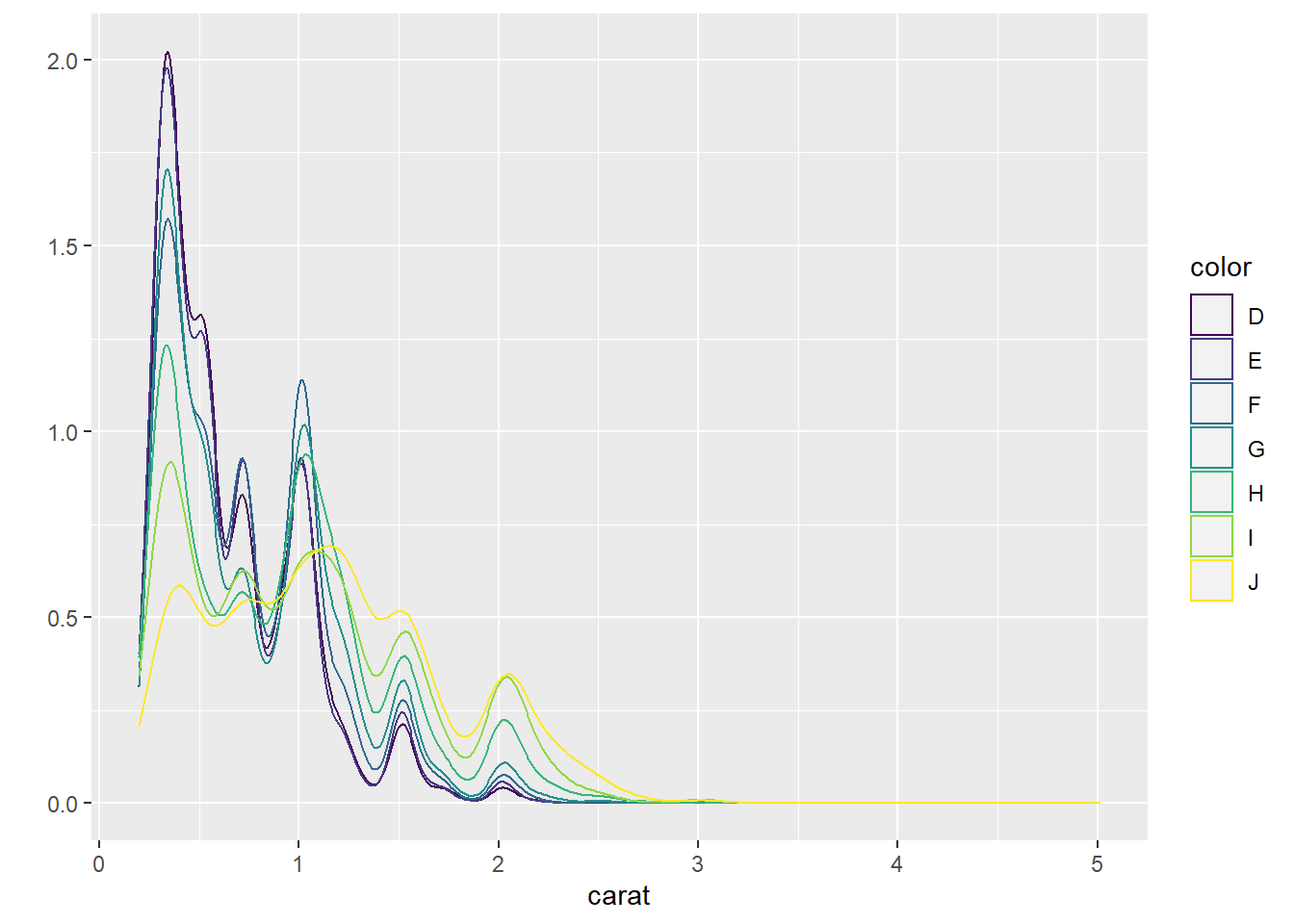### 4.10.5 Barplots

• Barplots are used to show the distribution of a categorical variable.
ggplot(data = diamonds, aes(x = clarity)) + geom_bar()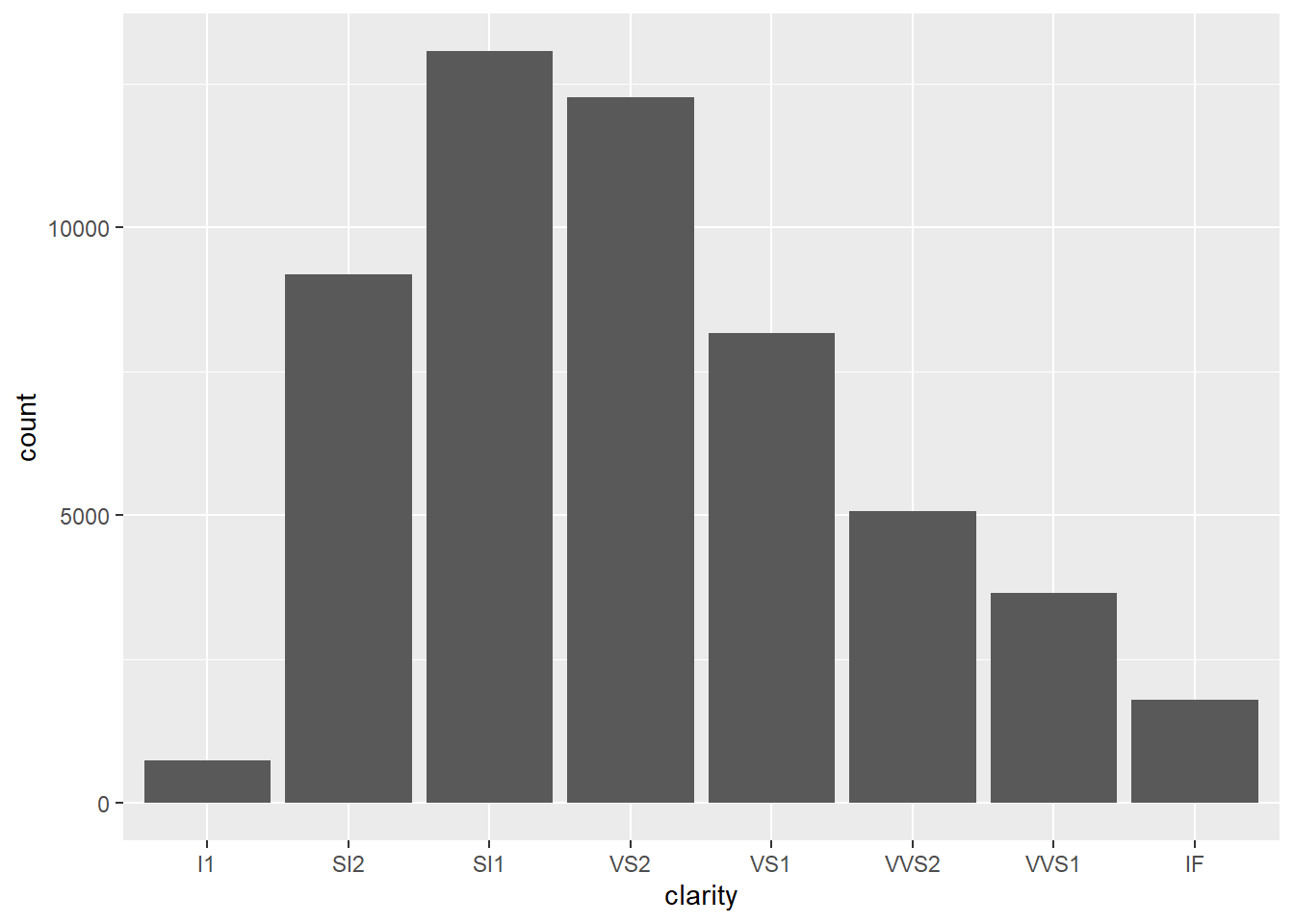qplot(clarity, data = diamonds, geom = "bar")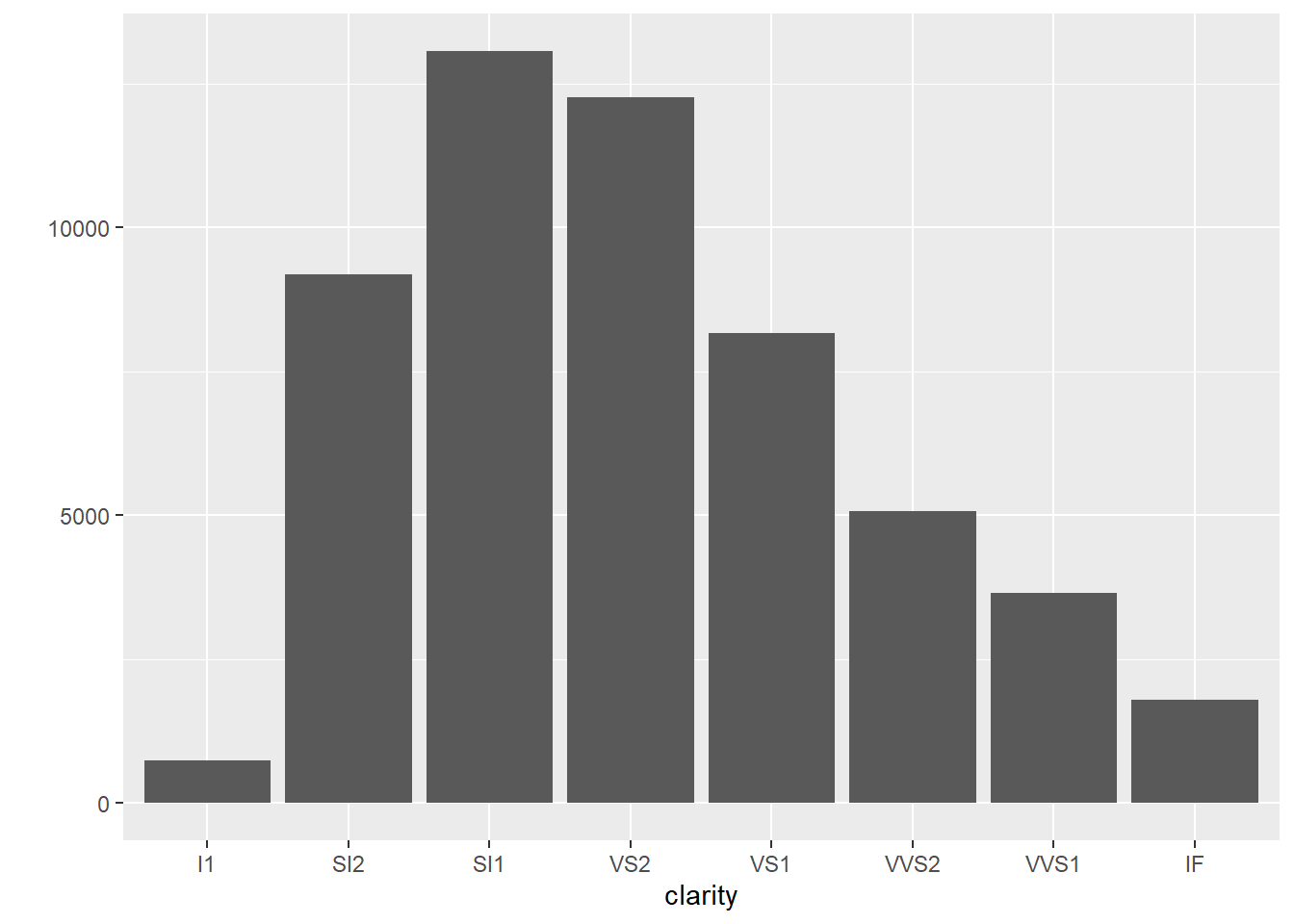ggplot(data = diamonds, aes(x = clarity, fill = cut)) + geom_bar()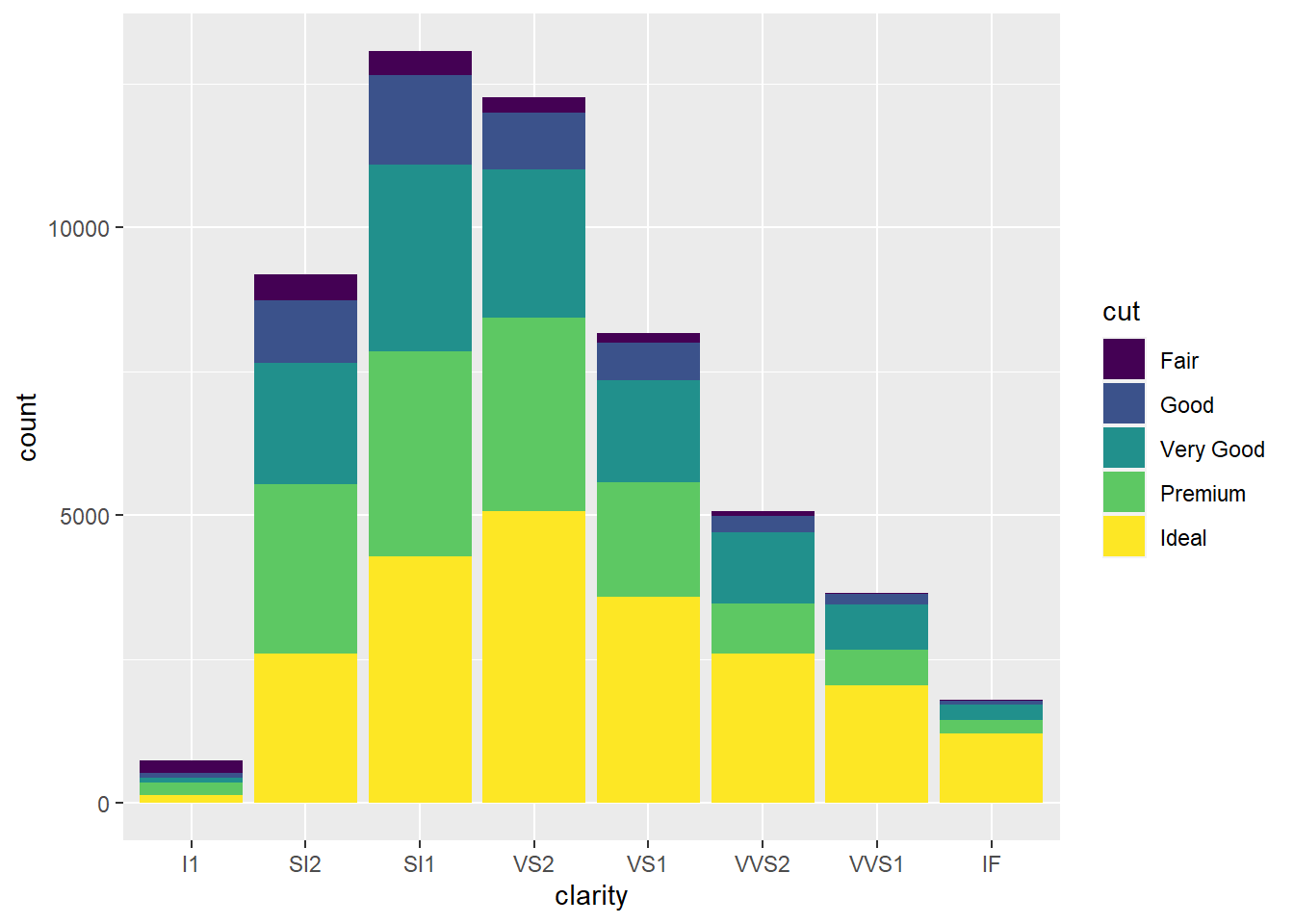qplot(clarity, data = diamonds, geom = "bar", fill = cut)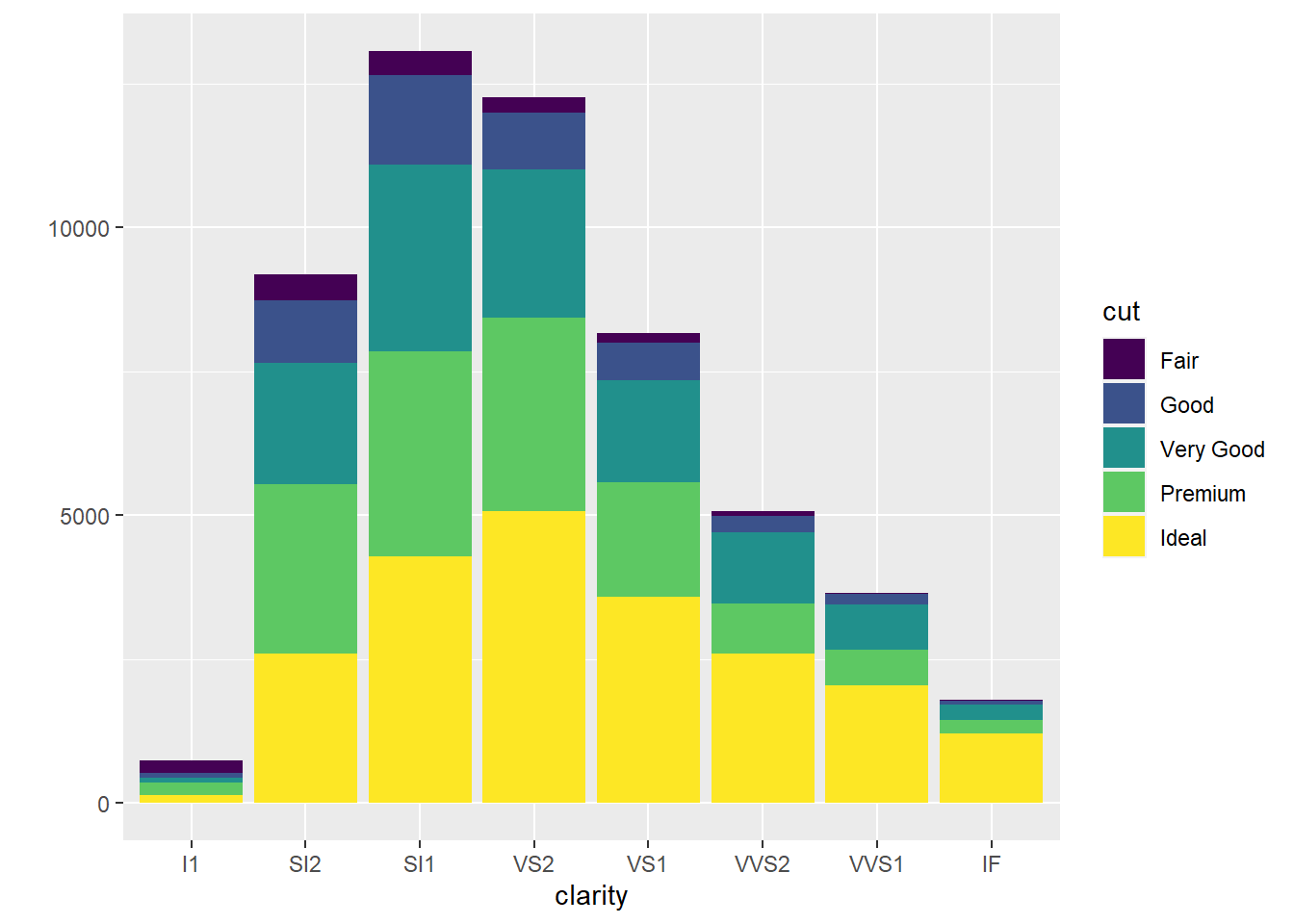### 4.10.6 Boxplots

• Boxplots are used to show a distribution of a numerical data based on five number summary(i.e., minimum, first quartile Q1, median, third quartile Q3, and maximum).

• Boxplots are often used to detect outliers based on interquartile range (IQR). Please check here for more details about boxplots.

ggplot(data = diamonds, aes(y = price)) + geom_boxplot()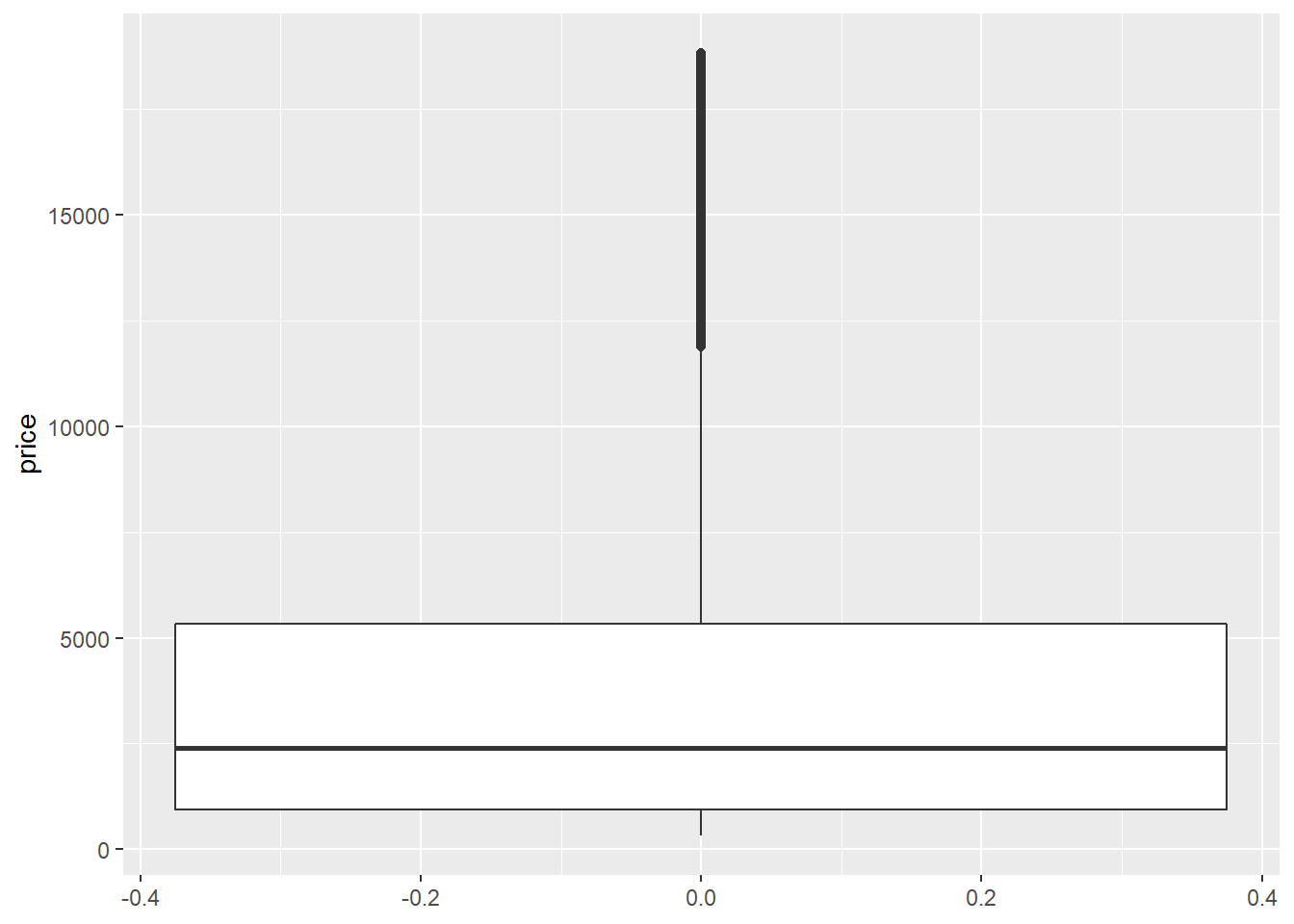qplot(y = price, data = diamonds, geom = "boxplot")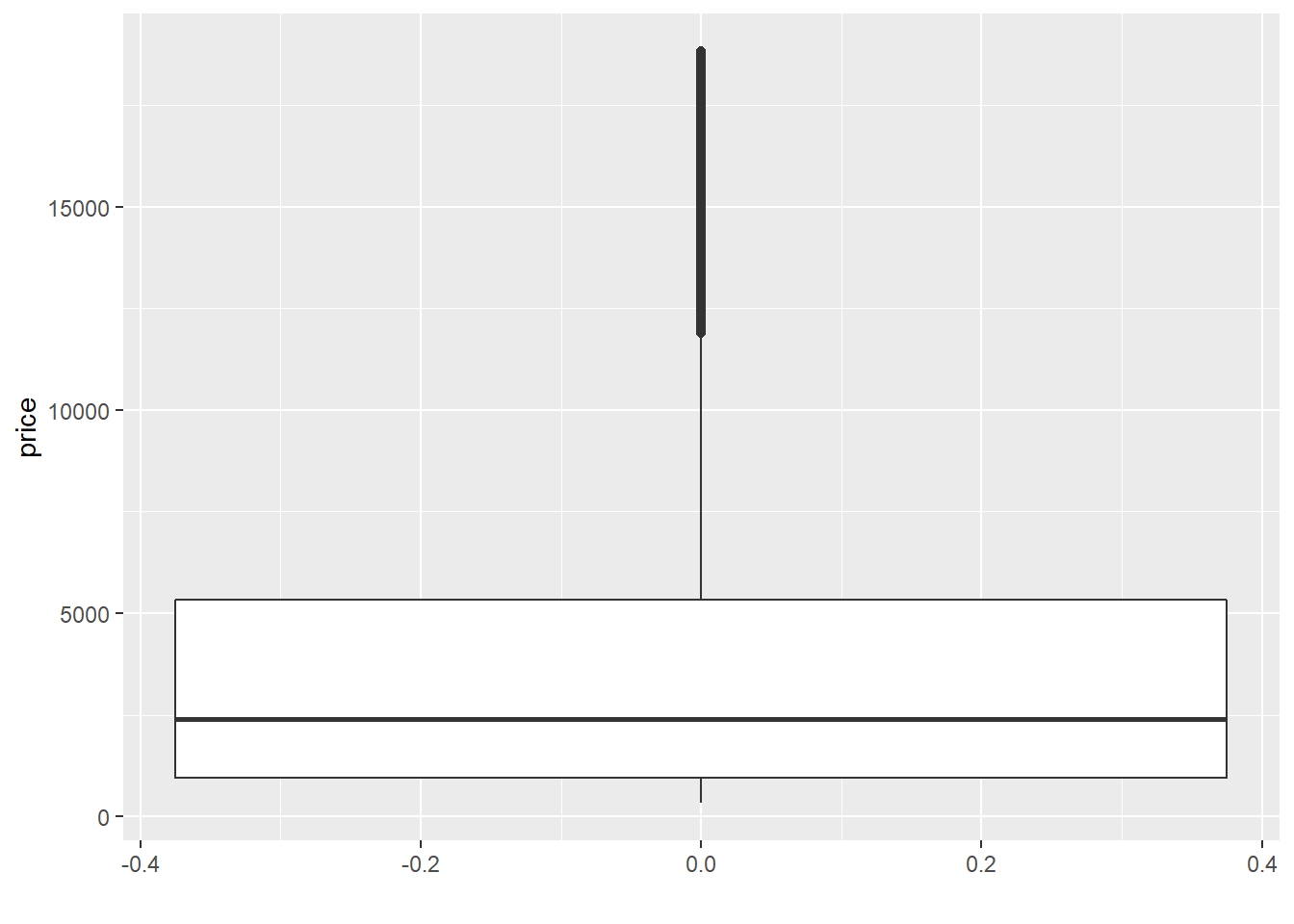ggplot(data = diamonds, aes(x = cut, y = price)) + geom_boxplot()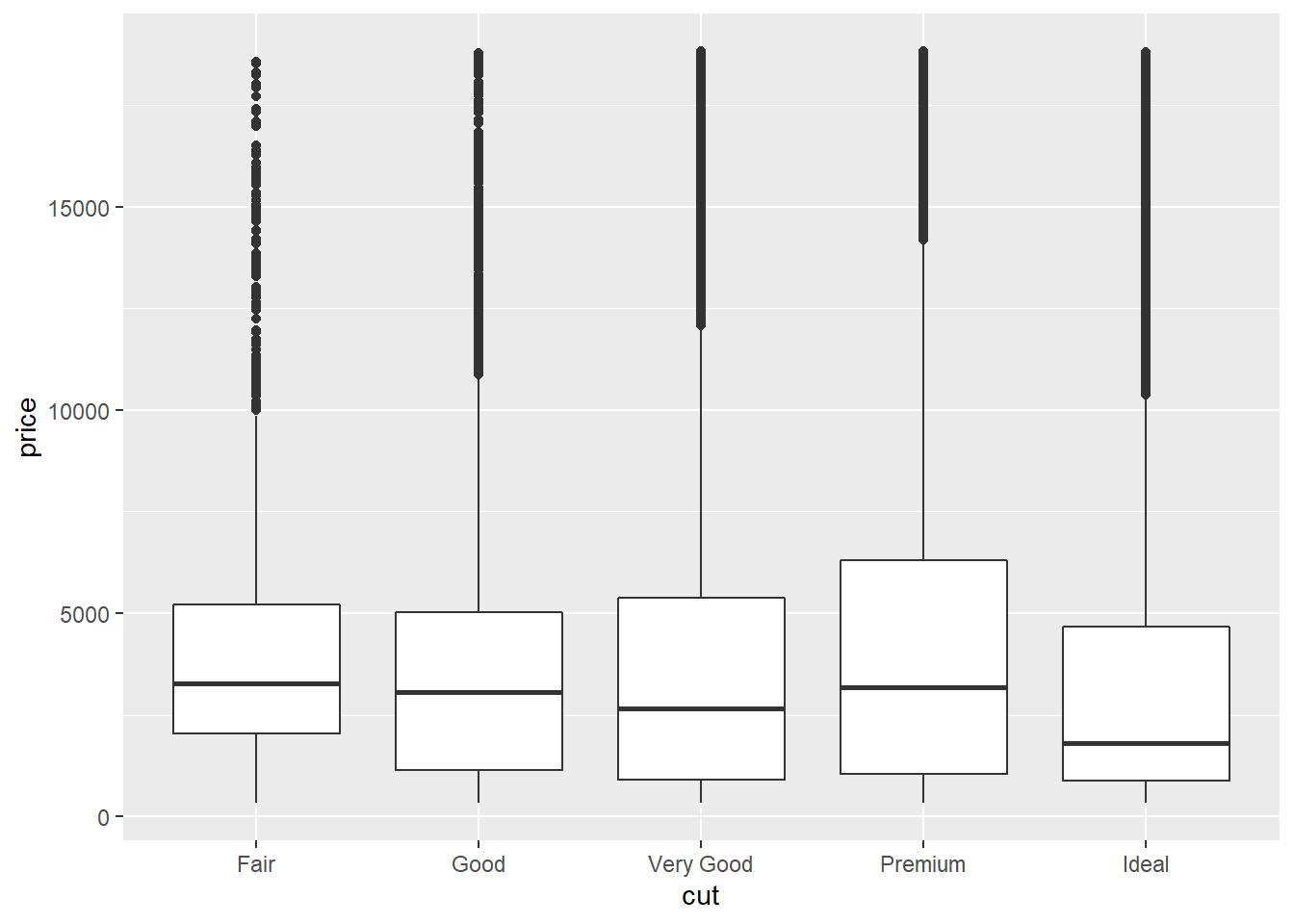### 4.10.7 The corrplot Package

library(corrplot)
## corrplot 0.90 loaded
corrplot.mixed(cor(mtcars))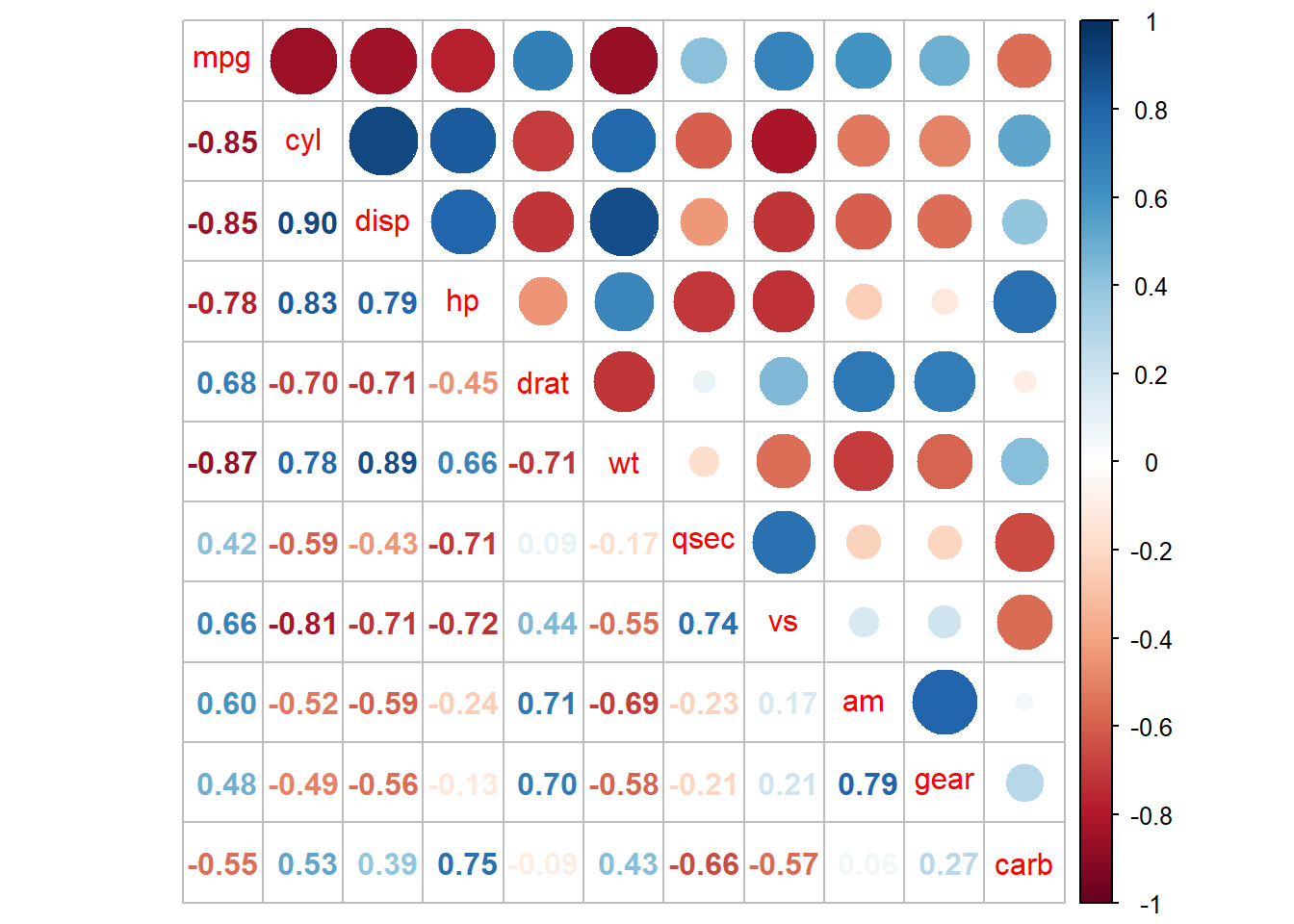## 4.11 Quiz 4

The gapminder foundation was founded to to fight devastating ignorance with a fact-based worldview everyone can understand. Check here for more details: https://www.gapminder.org/about/.

For fun, You can play with wonderful interactive plots from the gapminder foundation here.

Your assignment 1 (since I used the term Quiz and HW so far, I’m using the term assignment for this work. You will have 3-4 assignments in this semester) is a) to read the book “Factfulness” (in either Korean or English) and b) to write a minimum one page essay about what you learn and what you feel after reading the book. Since you need time to read the book for this assignment, please submit this assignment when you submit your “final project” at the end of this semester. So you have plenty(?) of time to submit this assignment. I will announce the instruction for the submission of the assignment 1 later. So just start to read the book.

Ok, that was the assignment 1. Now, let’s talk about your Quiz 4 for this week due on 23:59 Oct 3. For quiz 4, you will need 1) to “replicate” the several plots using your knowledge learned from this week’s reading in R for data science and my lecture. We will use the gapminder data from the gapminder package in R. I believe for now everyone knows that you need to install and load the gapminder package before you do this quiz, right?.

At the very beginning of this lecture notes, I presented the codes for the example using the gapminder data. If you learn more about the gapminder data, please type ?gapminder in your console, check the help documents, and check what each variable means. This week, we will only focus on how to actually create the plots. Next week, we will focus on how to make our plots more pretty and informative.

# This is a gapminder dataset
gapminder
## # A tibble: 1,704 x 6
##    country     continent  year lifeExp      pop gdpPercap
##    <fct>       <fct>     <int>   <dbl>    <int>     <dbl>
##  1 Afghanistan Asia       1952    28.8  8425333      779.
##  2 Afghanistan Asia       1957    30.3  9240934      821.
##  3 Afghanistan Asia       1962    32.0 10267083      853.
##  4 Afghanistan Asia       1967    34.0 11537966      836.
##  5 Afghanistan Asia       1972    36.1 13079460      740.
##  6 Afghanistan Asia       1977    38.4 14880372      786.
##  7 Afghanistan Asia       1982    39.9 12881816      978.
##  8 Afghanistan Asia       1987    40.8 13867957      852.
##  9 Afghanistan Asia       1992    41.7 16317921      649.
## 10 Afghanistan Asia       1997    41.8 22227415      635.
## # ... with 1,694 more rows

The two chunks of in our gapminder example can be combined into one chunk using the pipe operator (%>%) as in the code below. Notice that we don’t have data = gdp anymore because the pipe operator passed the data to the ggplot() function. Again, the pipe operator deliver the output of the left-hand side(lhs) as the input of the right-hand side(rhs). Please read each line of the codes carefully and check how it works.

# Code chunk 1: an example plot using ggplot2
# This plot comes from
# https://www.r-graph-gallery.com/320-the-basis-of-bubble-plot.html

library(gapminder)  # load gapminder package to use the gapminder data
library(tidyverse)  # load core tidyverse packages

# create data
gapminder %>%
filter(year=="2007") %>%     # subset rows ()
dplyr::select(-year) %>%     # subset columns (here remove year with minus sign)
arrange(desc(pop)) %>%       # arrange rows in descending order of pop
mutate(country = factor(country, country)) %>% # create a new factor country
ggplot(mapping = aes(x = gdpPercap, y = lifeExp, size = pop, fill = continent)) +  # aesthetic mappings
geom_point(alpha = 0.5, shape = 21, color = "black") +
scale_size(range = c(.1, 24), name = "Population (M)") +
theme_minimal() +
theme(legend.position = "right") +
labs(
subtitle = "Life Expectancy vs. GDP per Capita",
y = "Life Expectancy",
x = "GDP per Capita",
title = "Scatter Plot",
caption = "Source: gapminder"
)### 4.11.1 Istructions for Quiz 4

1. Replicate the six plots below using ggplot2 package.
2. Add a separate page in your bookdown (I believe all of you already published your own bookdown in Quiz 3) that contains six plots
3. Submit the URL for your updated Bookdown by 23:59 on Oct 3: https://forms.gle/52yhPS6WVS1qdrqP8

Quiz 4-1

To replicate this plot, you need to use filter(year=="2007") to filter only 2007 data.

gapminder %>%
filter(year=="2007") %>%     # subset rows ()
dplyr::select(-year) %>%     # subset columns (here remove year with minus sign)
arrange(desc(pop)) %>%       # arrange rows in descending order of pop
mutate(country = factor(country, country)) %>% # create a new factor country
ggplot(mapping = aes(x = lifeExp, fill = continent)) +  # aesthetic mappings
geom_histogram()
## stat_bin() using bins = 30. Pick better value with binwidth.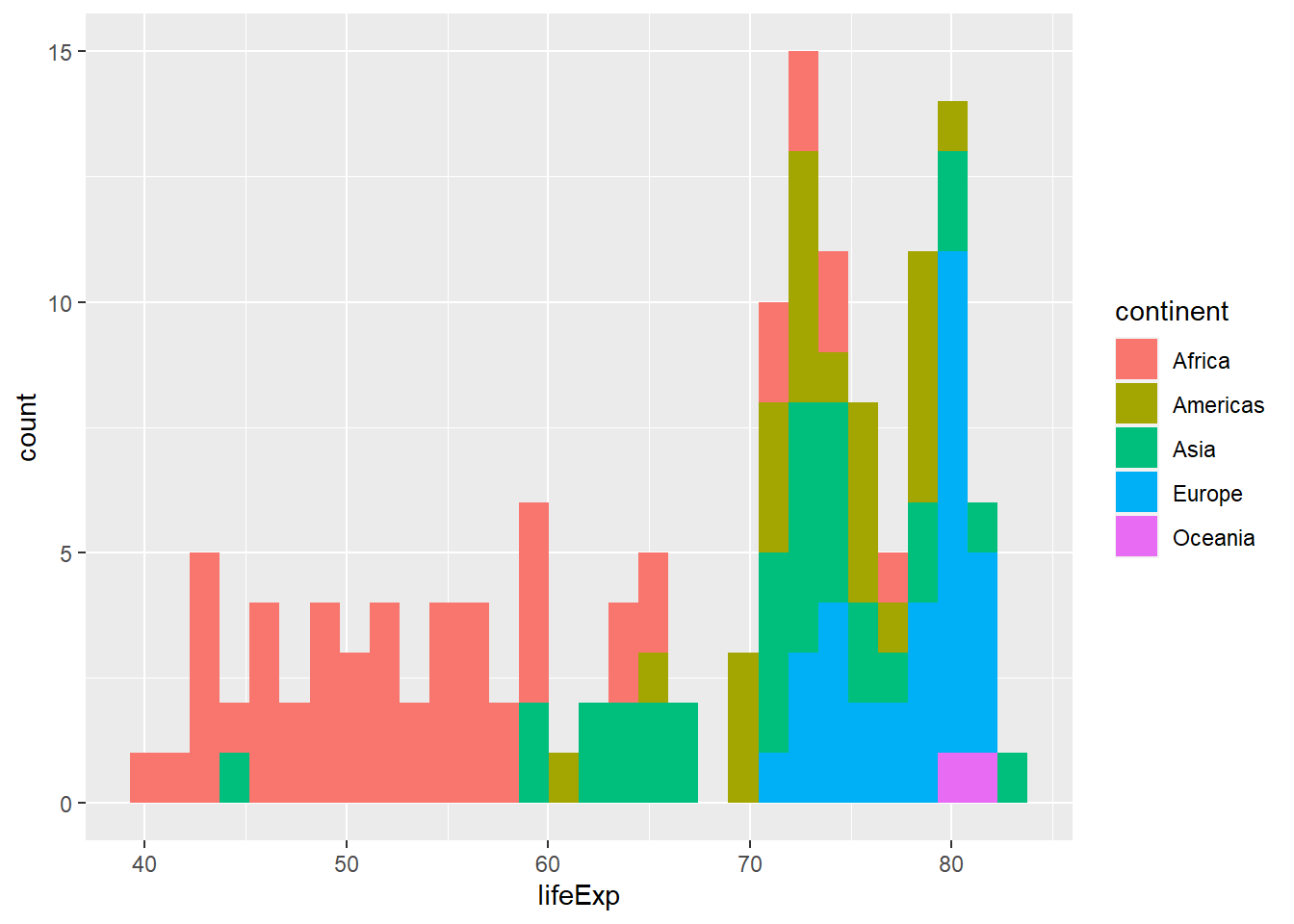Quiz 4-2

To replicate this plot, you need to use filter(year=="2007") to filter only 2007 data.

gapminder %>%
filter(year=="2007") %>%     # subset rows ()
dplyr::select(-year) %>%     # subset columns (here remove year with minus sign)
arrange(desc(pop)) %>%       # arrange rows in descending order of pop
mutate(country = factor(country, country)) %>% # create a new factor country
ggplot(mapping = aes(x = lifeExp, fill = continent)) +  # aesthetic mappings
geom_histogram() +
facet_wrap(vars(continent))
## stat_bin() using bins = 30. Pick better value with binwidth.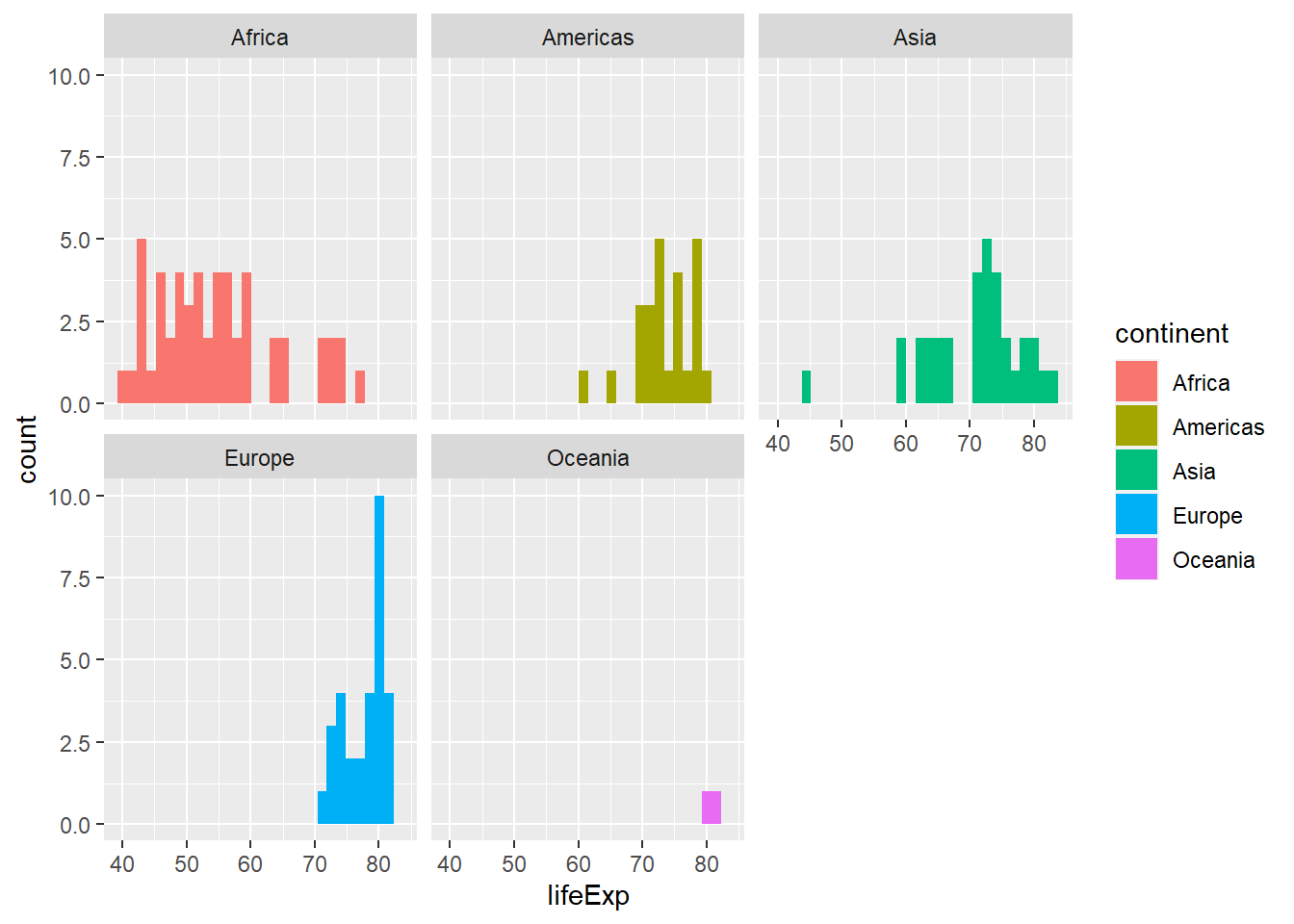Quiz 4-3

To replicate this plot, you need to use filter(year=="2007") to filter only 2007 data.

gapminder %>%
filter(year=="2007") %>%     # subset rows ()
dplyr::select(-year) %>%     # subset columns (here remove year with minus sign)
arrange(desc(pop)) %>%       # arrange rows in descending order of pop
mutate(country = factor(country, country)) %>% # create a new factor country
ggplot(mapping = aes(y = lifeExp, fill = continent)) +  # aesthetic mappings
geom_boxplot()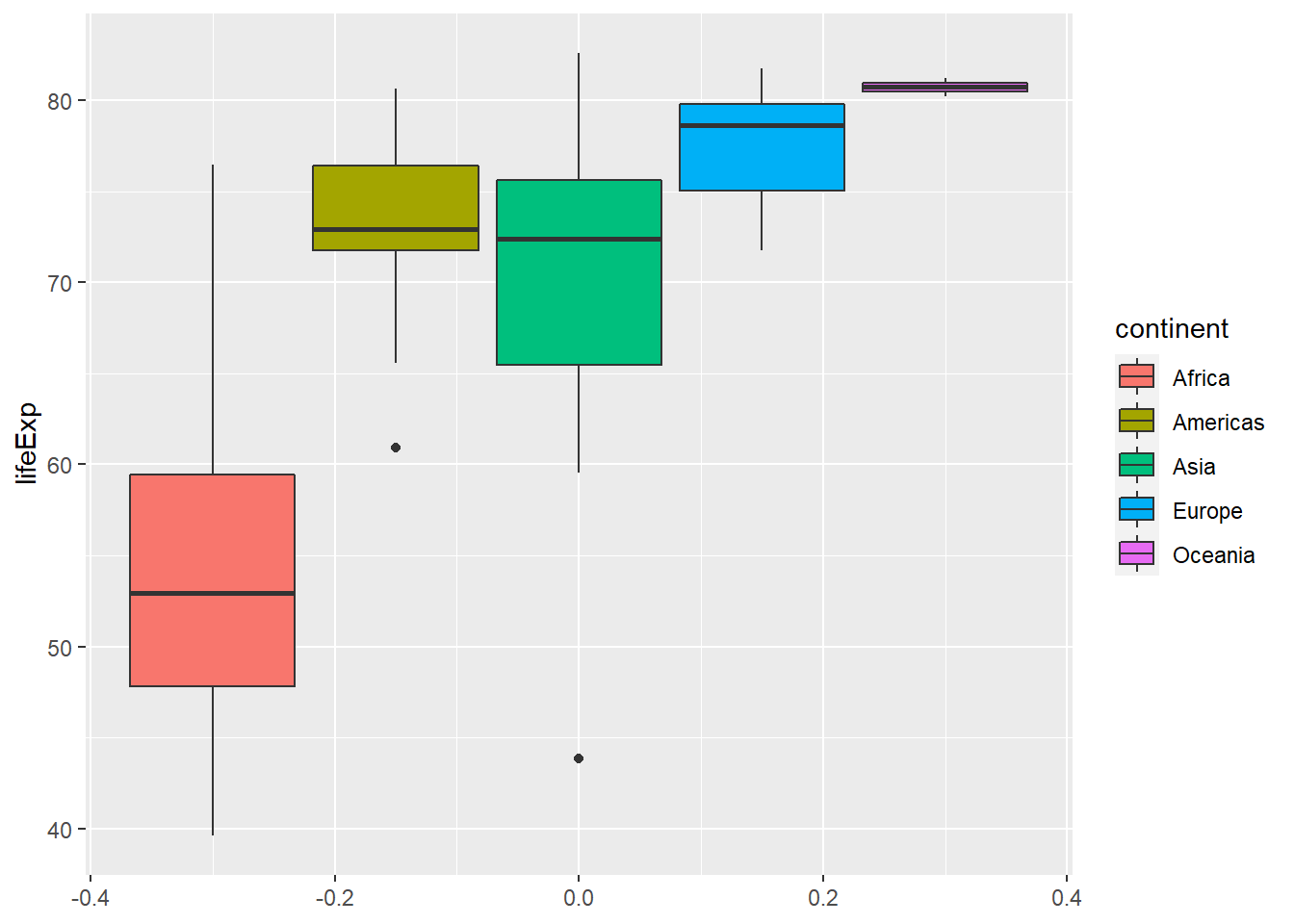Quiz 4-4

To replicate this plot, you need the entire data without filter(year=="2007")

gapminder %>%
ggplot(aes(x = year, y = lifeExp, group = country, color = continent)) +
geom_line()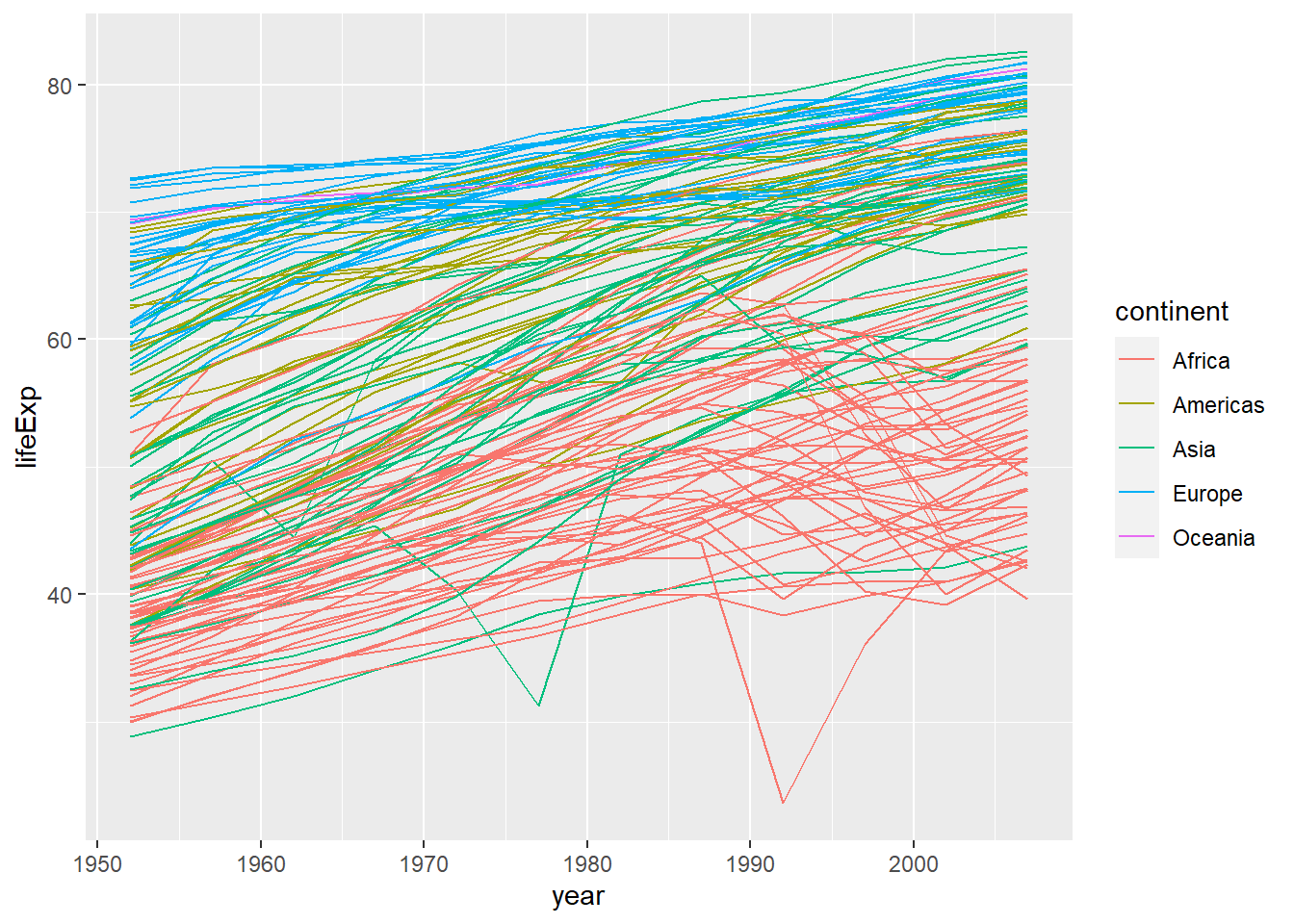Quiz 4-5

To replicate this plot, you need the entire data without filter(year=="2007")

gapminder %>%
ggplot(aes(x = year, y = lifeExp, group = country, color = continent)) +
geom_line()  +
facet_wrap(vars(continent))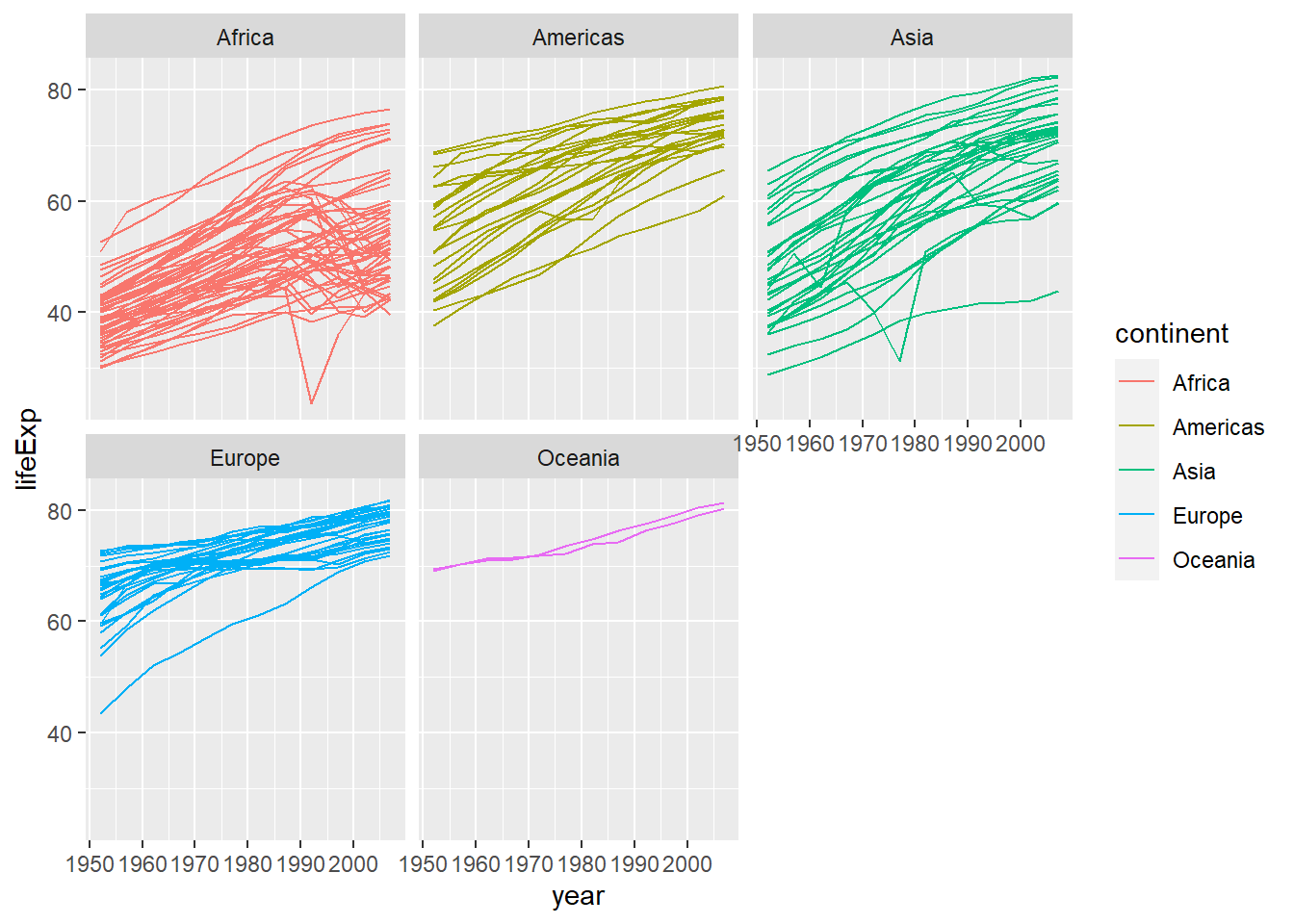Quiz 4-6

To replicate this plot, you need to use filter(year=="2007") to filter only 2007 data.

library(corrplot)
gapminder %>%
filter(year=="2007") %>%
select(lifeExp, pop, gdpPercap) %>%
cor() %>%
corrplot.mixed()